# US8212913B2 - Zoom lens system, imaging device and camera - Google Patents

## Info

Publication number
US8212913B2
US8212913B2 US12/676,048 US67604808A US8212913B2 US 8212913 B2 US8212913 B2 US 8212913B2 US 67604808 A US67604808 A US 67604808A US 8212913 B2 US8212913 B2 US 8212913B2
Authority
US
United States
Prior art keywords
lens unit
lens
zoom lens
wide
lens system
Prior art date
Legal status (The legal status is an assumption and is not a legal conclusion. Google has not performed a legal analysis and makes no representation as to the accuracy of the status listed.)
Active, expires
Application number
US12/676,048
Other versions
US20100194929A1 (en
Inventor
Keiki Yoshitsugu
Shunichiro Yoshinaga
Current Assignee (The listed assignees may be inaccurate. Google has not performed a legal analysis and makes no representation or warranty as to the accuracy of the list.)
Panasonic Corp
Original Assignee
Panasonic Corp
Priority date (The priority date is an assumption and is not a legal conclusion. Google has not performed a legal analysis and makes no representation as to the accuracy of the date listed.)
Filing date
Publication date
Priority to JP2007-236923 priority Critical
Priority to JP2007236926 priority
Priority to JP2007-236922 priority
Priority to JP2007236922 priority
Priority to JP2007236923 priority
Priority to JP2007-236926 priority
Priority to JP2008175473 priority
Priority to JP2008-175473 priority
Priority to PCT/JP2008/002515 priority patent/WO2009034712A1/en
Application filed by Panasonic Corp filed Critical Panasonic Corp
Assigned to PANASONIC CORPORATION reassignment PANASONIC CORPORATION ASSIGNMENT OF ASSIGNORS INTEREST (SEE DOCUMENT FOR DETAILS). Assignors: YAMADA, KATSU, YOSHINAGA, SHUNICHIRO, YOSHITSUGU, KEIKI
Publication of US20100194929A1 publication Critical patent/US20100194929A1/en
Application granted granted Critical
Publication of US8212913B2 publication Critical patent/US8212913B2/en
Application status is Active legal-status Critical

• 238000003384 imaging method Methods 0 claims description title 36
• 230000003287 optical Effects 0 abstract claims description 468
• 230000004323 axial length Effects 0 abstract claims description 27
• 230000002829 reduced Effects 0 abstract description 8
• 235000020127 ayran Nutrition 0 abstract 1
• 230000004075 alteration Effects 0 description 198
• 230000000694 effects Effects 0 description 67
• 210000001747 Pupil Anatomy 0 description 53
• 238000010276 construction Methods 0 description 16
• 230000000875 corresponding Effects 0 description 12
• 238000005549 size reduction Methods 0 description 10
• 206010010071 Coma Diseases 0 description 9
• 239000004973 liquid crystal related substances Substances 0 description 9
• 239000002609 media Substances 0 description 8
• 201000009310 astigmatism Diseases 0 description 7
• 239000002131 composite material Substances 0 description 7
• 230000004308 accommodation Effects 0 description 4
• 238000005452 bending Methods 0 description 4
• 230000001276 controlling effects Effects 0 description 4
• 238000009826 distribution Methods 0 description 4
• 239000000203 mixtures Substances 0 description 4
• 230000002093 peripheral Effects 0 description 4
• 230000035945 sensitivity Effects 0 description 4
• 230000001629 suppression Effects 0 description 4
• 239000007787 solids Substances 0 description 3
• 230000015572 biosynthetic process Effects 0 description 1
• 238000006243 chemical reaction Methods 0 description 1
• 230000003247 decreasing Effects 0 description 1
• 230000000593 degrading Effects 0 description 1
• 238000005755 formation Methods 0 description 1
• 230000014509 gene expression Effects 0 description 1
• 230000001976 improved Effects 0 description 1

## Images

•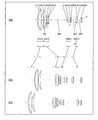•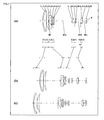•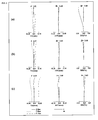•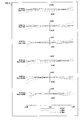•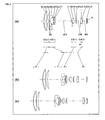••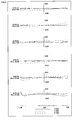•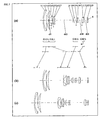•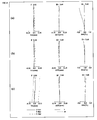•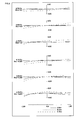•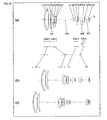••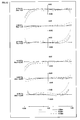•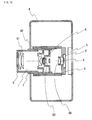•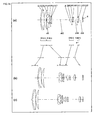••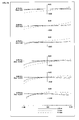•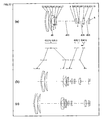•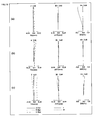•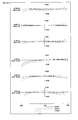•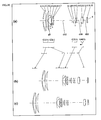•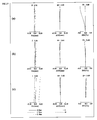•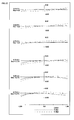•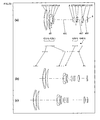•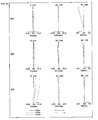••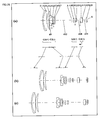•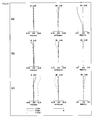•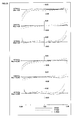••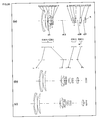•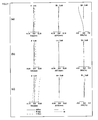•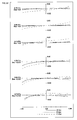•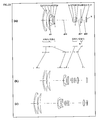•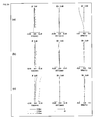•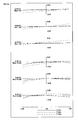•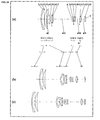•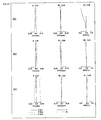•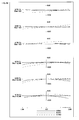•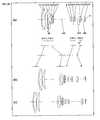•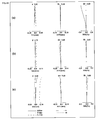•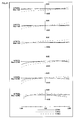•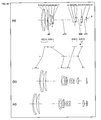•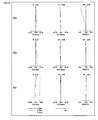•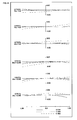•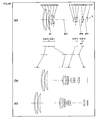•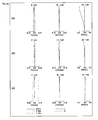•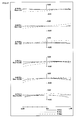•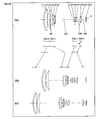•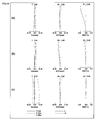•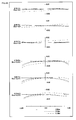•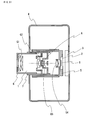•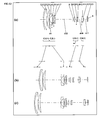•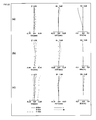•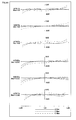•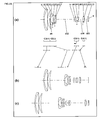••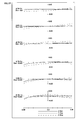•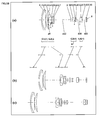•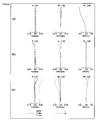•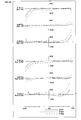•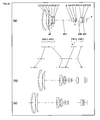•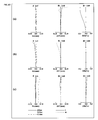•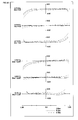•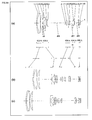•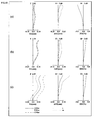•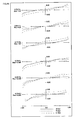•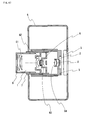## Classifications

• GPHYSICS
• G02OPTICS
• G02BOPTICAL ELEMENTS, SYSTEMS, OR APPARATUS
• G02B15/00Optical objectives with means for varying the magnification
• G02B15/14Optical objectives with means for varying the magnification by axial movement of one or more lenses or groups of lenses relative to the image plane for continuously varying the equivalent focal length of the objective
• G02B15/16Optical objectives with means for varying the magnification by axial movement of one or more lenses or groups of lenses relative to the image plane for continuously varying the equivalent focal length of the objective with interdependent non-linearly related movements between one lens or lens group, and another lens or lens group
• G02B15/163Optical objectives with means for varying the magnification by axial movement of one or more lenses or groups of lenses relative to the image plane for continuously varying the equivalent focal length of the objective with interdependent non-linearly related movements between one lens or lens group, and another lens or lens group having a first movable lens or lens group and a second movable lens or lens group, both in front of a fixed lens or lens group
• G02B15/167Optical objectives with means for varying the magnification by axial movement of one or more lenses or groups of lenses relative to the image plane for continuously varying the equivalent focal length of the objective with interdependent non-linearly related movements between one lens or lens group, and another lens or lens group having a first movable lens or lens group and a second movable lens or lens group, both in front of a fixed lens or lens group having an additional fixed front lens or group of lenses
• G02B15/173Optical objectives with means for varying the magnification by axial movement of one or more lenses or groups of lenses relative to the image plane for continuously varying the equivalent focal length of the objective with interdependent non-linearly related movements between one lens or lens group, and another lens or lens group having a first movable lens or lens group and a second movable lens or lens group, both in front of a fixed lens or lens group having an additional fixed front lens or group of lenses arranged +-+

## Abstract

A zoom lens system according to the present invention, from an object side to an image side, comprises a first lens unit having positive optical power, a second lens unit having negative optical power, a third lens unit having positive optical power, and a fourth lens unit having positive optical power. In zooming, the first to the fourth lens units all move along the optical axis. The conditions (1): 0<√(f4·fW·tan ω)/LW<0.13 and (2): 0.05≦f3/f4≦0.97 are satisfied (where, 16<fT/fW, ω>35; ω is a half view angle at a wide-angle limit; LW is an overall optical axial length of the entire system at a wide-angle limit; f3 is a focal length of the third lens unit; f4 is a focal length of the fourth lens unit; fT is a focal length of the entire system at a telephoto limit; and fW is a focal length of the entire system at a wide-angle limit). As a result, the zoom lens system has a reduced size and still realizes a wide view angle at a wide-angle limit, as well as a remarkably high zooming ratio and high performance.

## Description

TECHNICAL FIELD

The present invention relates to a zoom lens system, an imaging device and a camera. In particular, the present invention relates to: a zoom lens system having a reduced size and still realizing a wide view angle at a wide-angle limit, as well as a remarkably high zooming ratio and high performance; an imaging device employing this zoom lens system; and a thin and compact camera employing this imaging device.

BACKGROUND ART

Remarkably strong demands are present for improved performance of cameras such as digital still cameras and digital video cameras (simply referred to as digital cameras, hereinafter) provided with an image sensor for performing photoelectric conversion. In particular, in order that a single digital camera should be capable of covering a wide focal length range from a wide-angle condition to a high telephoto condition, cameras employing a zoom lens system having a remarkably high zooming ratio are strongly demanded from a convenience point of view. Further, in recent years, zoom lens systems are also desired that have a wide angle range where the photographing field is wide.

As zoom lens systems having high zooming ratios and suitable for the above-mentioned digital cameras, for example, the following zoom lens systems have been proposed.

For example, Japanese Laid-Open Patent Publication No. 2006-171655 discloses an image-taking optical system at least comprising a first lens unit having positive optical power, a second lens unit having negative optical power, a third lens unit having positive optical power, and a fourth lens unit having positive optical power, wherein any of the lens unit intervals is changed so that variable magnification is achieved, and wherein the ratio between the focal length of the third lens unit and the focal length of the fourth lens unit and the ratio between the focal length of the first lens unit and the focal length of the entire optical system at a wide-angle limit are set forth.

Japanese Laid-Open Patent Publication No. 2006-184413 discloses an image-taking optical system at least comprising a first lens unit having positive optical power, a second lens unit having negative optical power, a third lens unit having positive optical power, and a fourth lens unit having positive optical power, wherein at least the first lens unit is moved so that variable magnification is achieved, and wherein the ratio between the distance from the surface located on the most image-taking object side in the first lens unit at a wide-angle limit to the image formation surface and the focal length of the entire optical system at a telephoto limit and the ratio between the focal length of the first lens unit and the focal length of the entire optical system at a wide-angle limit are set forth.

Japanese Laid-Open Patent Publication No. 2006-184416 discloses an image-taking optical system at least comprising a first lens unit having positive optical power, a second lens unit having negative optical power, a third lens unit having positive optical power, and a fourth lens unit having positive optical power, wherein any of the lens unit intervals is changed so that variable magnification is achieved, and wherein the ratio between the focal length of the first lens unit and the focal length of the entire optical system at a wide-angle limit, the ratio between the ratio of the focal length of the second lens unit at a telephoto limit and at a wide-angle limit and the ratio of the focal length of the entire optical system at a telephoto limit and at a wide-angle limit, and the ratio between the magnification of the third lens unit at a telephoto limit and the magnification of the third lens unit at a wide-angle limit are set forth.

Japanese Laid-Open Patent Publication No. 2006-189598 discloses an image-taking optical system at least comprising a first lens unit having positive optical power, a second lens unit having negative optical power, a third lens unit having positive optical power, and a fourth lens unit having positive optical power, wherein the third lens unit at least includes two positive optical power lenses and one negative optical power lens, wherein at least the second, the third, and the fourth lens units are moved so that variable magnification is achieved, and wherein the ratio between the focal length of the first lens unit and the focal length of the entire optical system at a wide-angle limit, the ratio between the focal length of the negative optical power lens in the third lens unit and the focal length of the third lens unit, and the refractive index of the negative optical power lens in the third lens unit are set forth.

Japanese Laid-Open Patent Publication No. 2007-003554 discloses a variable magnification optical system at least comprising a first lens unit having positive optical power, a second lens unit having negative optical power, a third lens unit having positive optical power, and a fourth lens unit having positive optical power, wherein at least the first and the third lens units are moved so that variable magnification is achieved, wherein in this magnification change, the first lens unit is moved to the object side, and wherein the ratio between the amount of relative movement of the second lens unit at the time of magnification change and the focal length of the entire optical system at a wide-angle limit, the ratio between the focal length of the first lens unit and the focal length of the entire optical system at a wide-angle limit, and the ratio between the focal length of the third lens unit and the focal length of the entire optical system at a telephoto limit are set forth.

Japanese Laid-Open Patent Publication No. 2007-010695 discloses a variable magnification optical system at least comprising a first lens unit having positive optical power, a second lens unit having negative optical power, a third lens unit having positive optical power, and a fourth lens unit having positive optical power, wherein at least the first lens unit is moved so that variable magnification is achieved, and wherein the ratio between the focal length of the first lens unit and the focal length of the entire optical system at a wide-angle limit and the average refractive index to the d-line of all lenses in the second lens unit are set forth.

• Patent Document 1: Japanese Laid-Open Patent Publication No. 2006-171655
• Patent Document 2: Japanese Laid-Open Patent Publication No. 2006-184413
• Patent Document 3: Japanese Laid-Open Patent Publication No. 2006-184416
• Patent Document 4: Japanese Laid-Open Patent Publication No. 2006-189598
• Patent Document 5: Japanese Laid-Open Patent Publication No. 2007-003554
• Patent Document 6: Japanese Laid-Open Patent Publication No. 2007-010695
DISCLOSURE OF THE INVENTION Problems to be Solved by the Invention

The optical systems disclosed in the above-mentioned publications have high zooming ratios sufficient for application to digital cameras. Nevertheless, width of the view angle at a wide-angle limit and size reduction are not simultaneously realized. In particular, from the viewpoint of size reduction, requirements in digital cameras of recent years are not satisfied.

Objects of the present invention are to provide: a zoom lens system having a reduced size and still realizing a wide view angle at a wide-angle limit, as well as a remarkably high zooming ratio and high performance; an imaging device employing this zoom lens system; and a thin and compact camera employing this imaging device.

Solution to the Problems

(I) One of the above-mentioned objects is achieved by the following zoom lens system. That is, the present invention relates to

a zoom lens system, in order from an object side to an image side, comprising a first lens unit having positive optical power, a second lens unit having negative optical power, a third lens unit having positive optical power, and a fourth lens unit having positive optical power, wherein

in zooming from a wide-angle limit to a telephoto limit, all of the first lens unit, the second lens unit, the third lens unit and the fourth lens unit move along an optical axis, and wherein

the following conditions (1) and (2) are satisfied:
0<√(f 4 ·f W·tan ω)/L W<0.13  (1)
0.05≦f 3 /f 4≦0.97  (2)

(here, 16<fT/fW and ω>35)

where,

ω is a half view angle (°) at a wide-angle limit,

LW is an overall optical axial length of the entire system at a wide-angle limit (a distance from the most object side surface to the most image side surface),

f3 is a focal length of the third lens unit,

f4 is a focal length of the fourth lens unit,

fT is a focal length of the entire system at a telephoto limit, and

fW is a focal length of the entire system at a wide-angle limit.

One of the above-mentioned objects is achieved by the following imaging device. That is, the present invention relates to

an imaging device capable of outputting an optical image of an object as an electric image signal, comprising:

a zoom lens system that forms the optical image of the object; and

an image sensor that converts the optical image formed by the zoom lens system into the electric image signal, wherein

in the zoom lens system,

the zoom lens system, in order from an object side to an image side, comprises a first lens unit having positive optical power, a second lens unit having negative optical power, a third lens unit having positive optical power, and a fourth lens unit having positive optical power, wherein

in zooming from a wide-angle limit to a telephoto limit, all of the first lens unit, the second lens unit, the third lens unit and the fourth lens unit move along an optical axis, and wherein

the following conditions (1) and (2) are satisfied:
0<√(f 4 ·f W·tan ω)/L W<0.13  (1)
0.0 5≦f 3 /f 4≦0.97  (2)

(here, 16<fT /f W and ω>35)

where,

ω is a half view angle (°) at a wide-angle limit,

LW is an overall optical axial length of the entire system at a wide-angle limit (a distance from the most object side surface to the most image side surface),

f3 is a focal length of the third lens unit,

f4 is a focal length of the fourth lens unit,

fT is a focal length of the entire system at a telephoto limit, and

fW is a focal length of the entire system at a wide-angle limit.

One of the above-mentioned objects is achieved by the following camera. That is, the present invention relates to

a camera for converting an optical image of an object into an electric image signal and then performing at least one of displaying and storing of the converted image signal, comprising

an imaging device including a zoom lens system that forms the optical image of the object and an image sensor that converts the optical image formed by the zoom lens system into the electric image signal, wherein

in the zoom lens system,

the zoom lens system, in order from an object side to an image side, comprises a first lens unit having positive optical power, a second lens unit having negative optical power, a third lens unit having positive optical power, and a fourth lens unit having positive optical power, wherein

in zooming from a wide-angle limit to a telephoto limit, all of the first lens unit, the second lens unit, the third lens unit and the fourth lens unit move along an optical axis, and wherein

the following conditions (1) and (2) are satisfied:
0<√(f 4 ·f W·tan ω)/L W<0.13  (1)
0.05≦f 3 /f 4≦0.97  (2)

(here, 16<fT/fW and ω>35)

where,

ω is a half view angle (°) at a wide-angle limit,

LW is an overall optical axial length of the entire system at a wide-angle limit (a distance from the most object side surface to the most image side surface),

f3 is a focal length of the third lens unit,

f4 is a focal length of the fourth lens unit,

fT is a focal length of the entire system at a telephoto limit, and

fW is a focal length of the entire system at a wide-angle limit.

(II) One of the above-mentioned objects is achieved by the following zoom lens system. That is, the present invention relates to

a zoom lens system, in order from an object side to an image side, comprising a first lens unit having positive optical power, a second lens unit having negative optical power, a third lens unit having positive optical power, and a fourth lens unit having positive optical power, wherein

in zooming from a wide-angle limit to a telephoto limit, all of the first lens unit, the second lens unit, the third lens unit and the fourth lens unit move along an optical axis, wherein

the second lens unit includes a lens element having negative optical power and being arranged on the most object side and a lens element having positive optical power, and wherein

the following condition (3) is satisfied:
(nd 4−1)+(nd 6−1)≦1.8  (3)

(here, 16<fT/fW and ω>35)

where,

nd4 is a refractive index to the d-line of a lens element having negative optical power and being arranged on the most object side in the second lens unit,

nd6 is a refractive index to the d-line of a lens element having positive optical power in the second lens unit,

ω is a half view angle (°) at a wide-angle limit,

fT is a focal length of the entire system at a telephoto limit, and

fW is a focal length of the entire system at a wide-angle limit.

One of the above-mentioned objects is achieved by the following imaging device. That is, the present invention relates to

an imaging device capable of outputting an optical image of an object as an electric image signal, comprising:

a zoom lens system that forms the optical image of the object; and

an image sensor that converts the optical image formed by the zoom lens system into the electric image signal, wherein

in the zoom lens system,

the zoom lens system, in order from an object side to an image side, comprises a first lens unit having positive optical power, a second lens unit having negative optical power, a third lens unit having positive optical power, and a fourth lens unit having positive optical power, wherein

in zooming from a wide-angle limit to a telephoto limit, all of the first lens unit, the second lens unit, the third lens unit and the fourth lens unit move along an optical axis, wherein

the second lens unit includes a lens element having negative optical power and being arranged on the most object side and a lens element having positive optical power, and wherein

the following condition (3) is satisfied:
(nd 4−1)+(nd 6−1)≧1.8  (3)

(here, 16<fT/fW and ω>35)

where,

nd4 is a refractive index to the d-line of a lens element having negative optical power and being arranged on the most object side in the second lens unit,

nd6 is a refractive index to the d-line of a lens element having positive optical power in the second lens unit,

ω is a half view angle (°) at a wide-angle limit,

fT is a focal length of the entire system at a telephoto limit, and

fW is a focal length of the entire system at a wide-angle limit.

One of the above-mentioned objects is achieved by the following camera. That is, the present invention relates to

a camera for converting an optical image of an object into an electric image signal and then performing at least one of displaying and storing of the converted image signal, comprising

an imaging device including a zoom lens system that forms the optical image of the object and an image sensor that converts the optical image formed by the zoom lens system into the electric image signal, wherein

in the zoom lens system,

the zoom lens system, in order from an object side to an image side, comprises a first lens unit having positive optical power, a second lens unit having negative optical power, a third lens unit having positive optical power, and a fourth lens unit having positive optical power, wherein

in zooming from a wide-angle limit to a telephoto limit, all of the first lens unit, the second lens unit, the third lens unit and the fourth lens unit move along an optical axis, wherein

the second lens unit includes a lens element having negative optical power and being arranged on the most object side and a lens element having positive optical power, and wherein

the following condition (3) is satisfied:
(nd 4−1)+(nd 6−1)≧1.8  (3)

(here, 16<fT/fW and ω>35)

where,

nd4 is a refractive index to the d-line of a lens element having negative optical power and being arranged on the most object side in the second lens unit,

nd6 is a refractive index to the d-line of a lens element having positive optical power in the second lens unit,

ω is a half view angle (°) at a wide-angle limit,

fT is a focal length of the entire system at a telephoto limit, and

fW is a focal length of the entire system at a wide-angle limit.

(III) One of the above-mentioned objects is achieved by the following zoom lens system. That is, the present invention relates to

a zoom lens system, in order from an object side to an image side, comprising a first lens unit having positive optical power, a second lens unit having negative optical power, a third lens unit having positive optical power, and a fourth lens unit having positive optical power, wherein

in zooming from a wide-angle limit to a telephoto limit, all of the first lens unit, the second lens unit, the third lens unit and the fourth lens unit move along an optical axis, wherein

the first lens unit includes a lens element having negative optical power and being arranged on the most object side, and at least two lens elements having positive optical power that include a lens element having positive optical power and being arranged on the most image side, and wherein

the following conditions (4) and (5) are satisfied:
nd 1 −nd 2≧0.5  (4)
(nd 1−1)+(nd 3−1)≧1.8  (5)

(here, 16<fT/fW and ω>35)

where,

nd1 is a refractive index to the d-line of a lens element having negative optical power and being arranged on the most object side in the first lens unit,

nd2 is a refractive index to the d-line of a lens element located on the most object side among the lens elements having positive optical power in the first lens unit,

nd3 is a refractive index to the d-line of a lens element having positive optical power and being arranged on the most image side in the first lens unit,

ω is a half view angle (°) at a wide-angle limit,

fT is a focal length of the entire system at a telephoto limit, and

fW is a focal length of the entire system at a wide-angle limit.

One of the above-mentioned objects is achieved by the following imaging device. That is, the present invention relates to

an imaging device capable of outputting an optical image of an object as an electric image signal, comprising:

a zoom lens system that forms the optical image of the object; and

an image sensor that converts the optical image formed by the zoom lens system into the electric image signal, wherein

in the zoom lens system,

the zoom lens system, in order from an object side to an image side, comprises a first lens unit having positive optical power, a second lens unit having negative optical power, a third lens unit having positive optical power, and a fourth lens unit having positive optical power, wherein

in zooming from a wide-angle limit to a telephoto limit, all of the first lens unit, the second lens unit, the third lens unit and the fourth lens unit move along an optical axis, wherein

the first lens unit includes a lens element having negative optical power and being arranged on the most object side, and at least two lens elements having positive optical power that include a lens element having positive optical power and being arranged on the most image side, and wherein

the following conditions (4) and (5) are satisfied:
nd 1 −nd 2≧0.5  (4)
(nd 1−1)+(nd 3−1)≧1.8  (5)

(here, 16<fT/fW and ω>35)

where,

nd1 is a refractive index to the d-line of a lens element having negative optical power and being arranged on the most object side in the first lens unit,

nd2 is a refractive index to the d-line of a lens element located on the most object side among the lens elements having positive optical power in the first lens unit,

nd3 is a refractive index to the d-line of a lens element having positive optical power and being arranged on the most image side in the first lens unit,

ω is a half view angle (°) at a wide-angle limit,

fT is a focal length of the entire system at a telephoto limit, and

fW is a focal length of the entire system at a wide-angle limit.

One of the above-mentioned objects is achieved by the following camera. That is, the present invention relates to

a camera for converting an optical image of an object into an electric image signal and then performing at least one of displaying and storing of the converted image signal, comprising

an imaging device including a zoom lens system that forms the optical image of the object and an image sensor that converts the optical image formed by the zoom lens system into the electric image signal, wherein

in the zoom lens system,

the zoom lens system, in order from an object side to an image side, comprises a first lens unit having positive optical power, a second lens unit having negative optical power, a third lens unit having positive optical power, and a fourth lens unit having positive optical power, wherein

in zooming from a wide-angle limit to a telephoto limit, all of the first lens unit, the second lens unit, the third lens unit and the fourth lens unit move along an optical axis, wherein

the first lens unit includes a lens element having negative optical power and being arranged on the most object side, and at least two lens elements having positive optical power that include a lens element having positive optical power and being arranged on the most image side, and wherein

the following conditions (4) and (5) are satisfied:
nd 1 −nd 2≧0.5  (4)
(nd 1−1)+(nd 3−1)≧1.8  (5)

(here, 16<fT/fW and ω>35)

where,

nd1 is a refractive index to the d-line of a lens element having negative optical power and being arranged on the most object side in the first lens unit,

nd2 is a refractive index to the d-line of a lens element located on the most object side among the lens elements having positive optical power in the first lens unit,

nd3 is a refractive index to the d-line of a lens element having positive optical power and being arranged on the most image side in the first lens unit,

ω is a half view angle (°) at a wide-angle limit,

fT is a focal length of the entire system at a telephoto limit, and

fW is a focal length of the entire system at a wide-angle limit.

(IV) One of the above-mentioned objects is achieved by the following zoom lens system. That is, the present invention relates to

a zoom lens system, in order from an object side to an image side, comprising a first lens unit having positive optical power, a second lens unit having negative optical power, and subsequent lens units, wherein

the subsequent lens units include at least a third lens unit having positive optical power and a fourth lens unit having positive optical power, wherein

in zooming from a wide-angle limit to a telephoto limit, at least the first lens unit, the second lens unit, the third lens unit and the fourth lens unit move along an optical axis, and wherein

the following condition (IV-10) is satisfied:
1.95<m 2T /m 34T<3.50  (IV-10)

(here, 16<fT/fW and ω>35)

where,

m2T is a lateral magnification of the second lens unit at a telephoto limit,

m34T is a lateral magnification at a telephoto limit of a composite lens unit consisting of all lens units located on the image side relative to the second lens unit,

ω is a half view angle (°) at a wide-angle limit,

fT is a focal length of the entire system at a telephoto limit, and

fW is a focal length of the entire system at a wide-angle limit.

One of the above-mentioned objects is achieved by the following imaging device. That is, the present invention relates to

an imaging device capable of outputting an optical image of an object as an electric image signal, comprising:

a zoom lens system that forms the optical image of the object; and

an image sensor that converts the optical image formed by the zoom lens system into the electric image signal, wherein

in the zoom lens system,

the zoom lens system, in order from an object side to an image side, comprises a first lens unit having positive optical power, a second lens unit having negative optical power, and subsequent lens units, wherein

the subsequent lens units include at least a third lens unit having positive optical power and a fourth lens unit having positive optical power, wherein

in zooming from a wide-angle limit to a telephoto limit, at least the first lens unit, the second lens unit, the third lens unit and the fourth lens unit move along an optical axis, and wherein

the following condition (IV-10) is satisfied:
1.95<m 2T /m 34T<3.50  (IV-10)

(here, 16<fT/fW and ω>35)

where,

m2T is a lateral magnification of the second lens unit at a telephoto limit,

m34T is a lateral magnification at a telephoto limit of a composite lens unit consisting of all lens units located on the image side relative to the second lens unit,

ω is a half view angle (°) at a wide-angle limit,

fT is a focal length of the entire system at a telephoto limit, and

fW is a focal length of the entire system at a wide-angle limit.

One of the above-mentioned objects is achieved by the following camera. That is, the present invention relates to

a camera for converting an optical image of an object into an electric image signal and then performing at least one of displaying and storing of the converted image signal, comprising

an imaging device including a zoom lens system that forms the optical image of the object and an image sensor that converts the optical image formed by the zoom lens system into the electric image signal, wherein

in the zoom lens system,

the zoom lens system, in order from an object side to an image side, comprises a first lens unit having positive optical power, a second lens unit having negative optical power, and subsequent lens units, wherein

the subsequent lens units include at least a third lens unit having positive optical power and a fourth lens unit having positive optical power, wherein

in zooming from a wide-angle limit to a telephoto limit, at least the first lens unit, the second lens unit, the third lens unit and the fourth lens unit move along an optical axis, and wherein

the following condition (IV-10) is satisfied:
1.95<m 2T /m 34T<3.50  (IV-10)

(here, 16<fT/fW and ω>35)

where,

m2T is a lateral magnification of the second lens unit at a telephoto limit,

m34T is a lateral magnification at a telephoto limit of a composite lens unit consisting of all lens units located on the image side relative to the second lens unit,

ω is a half view angle (°) at a wide-angle limit,

fT is a focal length of the entire system at a telephoto limit, and

fW is a focal length of the entire system at a wide-angle limit.

Effects of the Invention

The present invention provides a zoom lens system having a reduced size and still realizes a wide view angle at a wide-angle limit, as well as a remarkably high zooming ratio and high performance. Further, according to the present invention, an imaging device employing this zoom lens system and a thin and compact camera employing this imaging device are provided.

BRIEF DESCRIPTION OF THE DRAWINGS

FIG. 1 is a lens arrangement diagram showing an infinity in-focus condition of a zoom lens system according to Embodiment I-1 (Example I-1).

FIG. 2 is a longitudinal aberration diagram of an infinity in-focus condition of a zoom lens system according to Example I-1.

FIG. 3 is a lateral aberration diagram in a basic state where image blur compensation is not performed and in an image blur compensation state at a telephoto limit of a zoom lens system according to Example I-1.

FIG. 4 is a lens arrangement diagram showing an infinity in-focus condition of a zoom lens system according to Embodiment I-2 (Example I-2).

FIG. 5 is a longitudinal aberration diagram of an infinity in-focus condition of a zoom lens system according to Example I-2.

FIG. 6 is a lateral aberration diagram in a basic state where image blur compensation is not performed and in an image blur compensation state at a telephoto limit of a zoom lens system according to Example I-2.

FIG. 7 is a lens arrangement diagram showing an infinity in-focus condition of a zoom lens system according to Embodiment I-3 (Example I-3).

FIG. 8 is a longitudinal aberration diagram of an infinity in-focus condition of a zoom lens system according to Example I-3.

FIG. 9 is a lateral aberration diagram in a basic state where image blur compensation is not performed and in an image blur compensation state at a telephoto limit of a zoom lens system according to Example I-3.

FIG. 10 is a lens arrangement diagram showing an infinity in-focus condition of a zoom lens system according to Embodiment I-4 (Example I-4).

FIG. 11 is a longitudinal aberration diagram of an infinity in-focus condition of a zoom lens system according to Example I-4.

FIG. 12 is a lateral aberration diagram in a basic state where image blur compensation is not performed and in an image blur compensation state at a telephoto limit of a zoom lens system according to Example I-4.

FIG. 13 is a schematic construction diagram of a digital still camera according to Embodiment I-5.

FIG. 14 is a lens arrangement diagram showing an infinity in-focus condition of a zoom lens system according to Embodiment II-1 (Example II-1).

FIG. 15 is a longitudinal aberration diagram of an infinity in-focus condition of a zoom lens system according to Example II-1.

FIG. 16 is a lateral aberration diagram in a basic state where image blur compensation is not performed and in an image blur compensation state at a telephoto limit of a zoom lens system according to Example II-1.

FIG. 17 is a lens arrangement diagram showing an infinity in-focus condition of a zoom lens system according to Embodiment II-2 (Example II-2).

FIG. 18 is a longitudinal aberration diagram of an infinity in-focus condition of a zoom lens system according to Example II-2.

FIG. 19 is a lateral aberration diagram in a basic state where image blur compensation is not performed and in an image blur compensation state at a telephoto limit of a zoom lens system according to Example II-2.

FIG. 20 is a lens arrangement diagram showing an infinity in-focus condition of a zoom lens system according to Embodiment II-3 (Example II-3).

FIG. 21 is a longitudinal aberration diagram of an infinity in-focus condition of a zoom lens system according to Example II-3.

FIG. 22 is a lateral aberration diagram in a basic state where image blur compensation is not performed and in an image blur compensation state at a telephoto limit of a zoom lens system according to Example II-3.

FIG. 23 is a lens arrangement diagram showing an infinity in-focus condition of a zoom lens system according to Embodiment II-4 (Example II-4).

FIG. 24 is a longitudinal aberration diagram of an infinity in-focus condition of a zoom lens system according to Example II-4.

FIG. 25 is a lateral aberration diagram in a basic state where image blur compensation is not performed and in an image blur compensation state at a telephoto limit of a zoom lens system according to Example II-4.

FIG. 26 is a lens arrangement diagram showing an infinity in-focus condition of a zoom lens system according to Embodiment II-5 (Example II-5).

FIG. 27 is a longitudinal aberration diagram of an infinity in-focus condition of a zoom lens system according to Example II-5.

FIG. 28 is a lateral aberration diagram in a basic state where image blur compensation is not performed and in an image blur compensation state at a telephoto limit of a zoom lens system according to Example II-5.

FIG. 29 is a schematic construction diagram of a digital still camera according to Embodiment II-6.

FIG. 30 is a lens arrangement diagram showing an infinity in-focus condition of a zoom lens system according to Embodiment III-1 (Example III-1).

FIG. 31 is a longitudinal aberration diagram of an infinity in-focus condition of a zoom lens system according to Example III-1.

FIG. 32 is a lateral aberration diagram in a basic state where image blur compensation is not performed and in an image blur compensation state at a telephoto limit of a zoom lens system according to Example III-1.

FIG. 33 is a lens arrangement diagram showing an infinity in-focus condition of a zoom lens system according to Embodiment III-2 (Example III-2).

FIG. 34 is a longitudinal aberration diagram of an infinity in-focus condition of a zoom lens system according to Example III-2.

FIG. 35 is a lateral aberration diagram in a basic state where image blur compensation is not performed and in an image blur compensation state at a telephoto limit of a zoom lens system according to Example III-2.

FIG. 36 is a lens arrangement diagram showing an infinity in-focus condition of a zoom lens system according to Embodiment III-3 (Example III-3).

FIG. 37 is a longitudinal aberration diagram of an infinity in-focus condition of a zoom lens system according to Example III-3.

FIG. 38 is a lateral aberration diagram in a basic state where image blur compensation is not performed and in an image blur compensation state at a telephoto limit of a zoom lens system according to Example III-3.

FIG. 39 is a lens arrangement diagram showing an infinity in-focus condition of a zoom lens system according to Embodiment III-4 (Example III-4).

FIG. 40 is a longitudinal aberration diagram of an infinity in-focus condition of a zoom lens system according to Example III-4.

FIG. 41 is a lateral aberration diagram in a basic state where image blur compensation is not performed and in an image blur compensation state at a telephoto limit of a zoom lens system according to Example III-4.

FIG. 42 is a lens arrangement diagram showing an infinity in-focus condition of a zoom lens system according to Embodiment III-5 (Example III-5).

FIG. 43 is a longitudinal aberration diagram of an infinity in-focus condition of a zoom lens system according to Example III-5.

FIG. 44 is a lateral aberration diagram in a basic state where image blur compensation is not performed and in an image blur compensation state at a telephoto limit of a zoom lens system according to Example III-5.

FIG. 45 is a lens arrangement diagram showing an infinity in-focus condition of a zoom lens system according to Embodiment III-6 (Example III-6).

FIG. 46 is a longitudinal aberration diagram of an infinity in-focus condition of a zoom lens system according to Example III-6.

FIG. 47 is a lateral aberration diagram in a basic state where image blur compensation is not performed and in an image blur compensation state at a telephoto limit of a zoom lens system according to Example III-6.

FIG. 48 is a lens arrangement diagram showing an infinity in-focus condition of a zoom lens system according to Embodiment III-7 (Example III-7).

FIG. 49 is a longitudinal aberration diagram of an infinity in-focus condition of a zoom lens system according to Example III-7.

FIG. 50 is a lateral aberration diagram in a basic state where image blur compensation is not performed and in an image blur compensation state at a telephoto limit of a zoom lens system according to Example III-7.

FIG. 51 is a schematic construction diagram of a digital still camera according to Embodiment III-8.

FIG. 52 is a lens arrangement diagram showing an infinity in-focus condition of a zoom lens system according to Embodiment IV-1 (Example IV-1).

FIG. 53 is a longitudinal aberration diagram of an infinity in-focus condition of a zoom lens system according to Example IV-1.

FIG. 54 is a lateral aberration diagram in a basic state where image blur compensation is not performed and in an image blur compensation state at a telephoto limit of a zoom lens system according to Example IV-1.

FIG. 55 is a lens arrangement diagram showing an infinity in-focus condition of a zoom lens system according to Embodiment IV-2 (Example IV-2).

FIG. 56 is a longitudinal aberration diagram of an infinity in-focus condition of a zoom lens system according to Example IV-2.

FIG. 57 is a lateral aberration diagram in a basic state where image blur compensation is not performed and in an image blur compensation state at a telephoto limit of a zoom lens system according to Example IV-2.

FIG. 58 is a lens arrangement diagram showing an infinity in-focus condition of a zoom lens system according to Embodiment IV-3 (Example IV-3).

FIG. 59 is a longitudinal aberration diagram of an infinity in-focus condition of a zoom lens system according to Example IV-3.

FIG. 60 is a lateral aberration diagram in a basic state where image blur compensation is not performed and in an image blur compensation state at a telephoto limit of a zoom lens system according to Example IV-3.

FIG. 61 is a lens arrangement diagram showing an infinity in-focus condition of a zoom lens system according to Embodiment IV-4 (Example IV-4).

FIG. 62 is a longitudinal aberration diagram of an infinity in-focus condition of a zoom lens system according to Example IV-4.

FIG. 63 is a lateral aberration diagram in a basic state where image blur compensation is not performed and in an image blur compensation state at a telephoto limit of a zoom lens system according to Example IV-4.

FIG. 64 is a lens arrangement diagram showing an infinity in-focus condition of a zoom lens system according to Embodiment IV-5 (Example IV-5).

FIG. 65 is a longitudinal aberration diagram of an infinity in-focus condition of a zoom lens system according to Example IV-5.

FIG. 66 is a lateral aberration diagram in a basic state where image blur compensation is not performed and in an image blur compensation state at a telephoto limit of a zoom lens system according to Example IV-5.

FIG. 67 is a schematic construction diagram of a digital still camera according to Embodiment IV-6.

DESCRIPTION OF THE REFERENCE CHARACTERS

• G1 first lens unit
• G2 second lens unit
• G3 third lens unit
• G4 fourth lens unit
• L1 first lens element
• L2 second lens element
• L3 third lens element
• L4 fourth lens element
• L5 fifth lens element
• L6 sixth lens element
• L7 seventh lens element
• L8 eighth lens element
• L9 ninth lens element
• L10 tenth lens element
• L11 eleventh lens element
• L12 twelfth lens element
• L12, L13 plane parallel plate
• A diaphragm
• S image surface
• 1 zoom lens system
• 2 image sensor
• 3 liquid crystal display monitor
• 4 body
• 5 main barrel
• 6 moving barrel
• 7 cylindrical cam
BEST MODE FOR CARRYING OUT THE INVENTION Embodiments I-1 to I-4

FIG. 1 is a lens arrangement diagram of a zoom lens system according to Embodiment I-1. FIG. 4 is a lens arrangement diagram of a zoom lens system according to Embodiment I-2. FIG. 7 is a lens arrangement diagram of a zoom lens system according to Embodiment I-3. FIG. 10 is a lens arrangement diagram of a zoom lens system according to Embodiment I-4.

FIGS. 1, 4, 7 and 10 show respectively a zoom lens system in an infinity in-focus condition. In each figure, part (a) shows a lens configuration at a wide-angle limit (in the minimum focal length condition: focal length fW), part (b) shows a lens configuration at a middle position (in an intermediate focal length condition: focal length fM=√(fW*fT)), and part (c) shows a lens configuration at a telephoto limit (in the maximum focal length condition: focal length fT). Further, in each figure, bent arrows provided between part (a) and part (b) are lines obtained by connecting the positions of the lens units at a wide-angle limit, at a middle position and at a telephoto limit, in order from the top to the bottom. Thus, straight lines are used simply between a wide-angle limit and a middle position and between a middle position and a telephoto limit. That is, these straight lines do not indicate the actual motion of the individual lens units. Moreover, in each figure, an arrow provided to a lens unit indicates focusing from an infinity in-focus condition to a close-object in-focus condition, that is, the moving direction at the time of focusing from an infinity in-focus condition to a close-object in-focus condition.

The zoom lens system according to each embodiment, in order from the object side to the image side, comprises a first lens unit G1 having positive optical power, a second lens unit G2 having negative optical power, a third lens unit G3 having positive optical power, and a fourth lens unit G4 having positive optical power. Then, in zooming from a wide-angle limit to a telephoto limit, the first lens unit G1, the second lens unit G2, the third lens unit G3 and the fourth lens unit G4 all move along the optical axis (this lens configuration is referred to as the basic configuration I of Embodiments I-1 to I-4, hereinafter). In the zoom lens system according to each embodiment, these lens units are arranged into a desired optical power arrangement, so that a remarkably high zooming ratio exceeding 16 and high optical performance are achieved and still size reduction is realized in the entire lens system.

In FIGS. 1, 4, 7 and 10, an asterisk “*” provided to a particular surface indicates that the surface is aspheric. Further, in each figure, a symbol (+) or (−) provided to the sign of each lens unit corresponds to the sign of optical power of the lens unit. Moreover, in each figure, the straight line located on the most right-hand side indicates the position of an image surface S. On the object side relative to the image surface S (between the image surface S and the most image side lens surface of the fourth lens unit G4), a plane parallel plate such as an optical low-pass filter and a face plate of an image sensor is provided. Moreover, in each figure, a diaphragm A is provided between the most image side lens surface of the second lens unit G2 and the most object side lens surface of the third lens unit G3.

As shown in FIG. 1, in the zoom lens system according to Embodiment I-1, the first lens unit G1, in order from the object side to the image side, comprises: a negative meniscus first lens element L1 with the convex surface facing the object side; a bi-convex second lens element L2; and a positive meniscus third lens element L3 with the convex surface facing the object side. Among these, the first lens element L1 and the second lens element L2 are cemented with each other.

In the zoom lens system according to Embodiment I-1, the second lens unit G2, in order from the object side to the image side, comprises: a negative meniscus fourth lens element L4 with the convex surface facing the object side; a bi-concave fifth lens element L5; a bi-convex sixth lens element L6; and a bi-concave seventh lens element L7. Among these, the sixth lens element L6 and the seventh lens element L7 are cemented with each other.

Further, in the zoom lens system according to Embodiment I-1, the third lens unit G3, in order from the object side to the image side, comprises: a positive meniscus eighth lens element L8 with the convex surface facing the object side; a bi-convex ninth lens element L9; and a bi-concave tenth lens element L10. Among these, the ninth lens element L9 and the tenth lens element L10 are cemented with each other.

Further, in the zoom lens system according to Embodiment I-1, the fourth lens unit G4, in order from the object side to the image side, comprises: a bi-convex eleventh lens element L11; and a negative meniscus twelfth lens element L12 with the convex surface facing the image side. The eleventh lens element L11 and the twelfth lens element L12 are cemented with each other.

Here, in the zoom lens system according to Embodiment I-1, a plane parallel plate L13 is provided on the object side relative to the image surface S (between the image surface S and the twelfth lens element L12).

In the zoom lens system according to Embodiment I-1, in zooming from a wide-angle limit to a telephoto limit, the first lens unit G1 and the third lens unit G3 move to the object side, while the second lens unit G2 moves to the image side, that is, such that the position at a wide-angle limit should be located on the object side relative to the position at a telephoto limit. Further, the fourth lens unit G4 moves with locus of a convex to the object side with changing the interval with the third lens unit G3.

As shown in FIG. 4, in the zoom lens system according to Embodiment I-2, the first lens unit G1, in order from the object side to the image side, comprises: a negative meniscus first lens element L1 with the convex surface facing the object side; a positive meniscus second lens element L2 with the convex surface facing the object side; and a positive meniscus third lens element L3 with the convex surface facing the object side. Among these, the first lens element L1 and the second lens element L2 are cemented with each other.

In the zoom lens system according to Embodiment I-2, the second lens unit G2, in order from the object side to the image side, comprises: a negative meniscus fourth lens element L4 with the convex surface facing the object side; a bi-concave fifth lens element L5; a bi-convex sixth lens element L6; and a negative meniscus seventh lens element L7 with the convex surface facing the image side. Among these, the sixth lens element L6 and the seventh lens element L7 are cemented with each other.

Further, in the zoom lens system according to Embodiment I-2, the third lens unit G3, in order from the object side to the image side, comprises: a bi-convex eighth lens element L8; a bi-convex ninth lens element L9; and a bi-concave tenth lens element L10. Among these, the ninth lens element L9 and the tenth lens element L10 are cemented with each other.

Further, in the zoom lens system according to Embodiment I-2, the fourth lens unit G4, in order from the object side to the image side, comprises: a bi-convex eleventh lens element L11; and a negative meniscus twelfth lens element L12 with the convex surface facing the image side. The eleventh lens element L11 and the twelfth lens element L12 are cemented with each other.

Here, in the zoom lens system according to Embodiment I-2, a plane parallel plate L13 is provided on the object side relative to the image surface S (between the image surface S and the twelfth lens element L12).

In the zoom lens system according to Embodiment I-2, in zooming from a wide-angle limit to a telephoto limit, the first lens unit G1 and the third lens unit G3 move to the object side, while the second lens unit G2 moves to the image side, that is, such that the position at a wide-angle limit should be located on the object side relative to the position at a telephoto limit. Further, the fourth lens unit G4 moves with locus of a convex to the object side with changing the interval with the third lens unit G3.

As shown in FIG. 7, in the zoom lens system according to Embodiment I-3, the first lens unit G1, in order from the object side to the image side, comprises: a negative meniscus first lens element L1 with the convex surface facing the object side; a positive meniscus second lens element L2 with the convex surface facing the object side; and a positive meniscus third lens element L3 with the convex surface facing the object side. Among these, the first lens element L1 and the second lens element L2 are cemented with each other.

In the zoom lens system according to Embodiment I-3, the second lens unit G2, in order from the object side to the image side, comprises: a negative meniscus fourth lens element L4 with the convex surface facing the object side; a bi-concave fifth lens element L5; a bi-convex sixth lens element L6; and a bi-concave seventh lens element L7. Among these, the sixth lens element L6 and the seventh lens element L7 are cemented with each other.

Further, in the zoom lens system according to Embodiment I-3, the third lens unit G3, in order from the object side to the image side, comprises: a positive meniscus eighth lens element L8 with the convex surface facing the object side; a bi-convex ninth lens element L9; and a bi-concave tenth lens element L10. Among these, the ninth lens element L9 and the tenth lens element L10 are cemented with each other.

Further, in the zoom lens system according to Embodiment I-3, the fourth lens unit G4, in order from the object side to the image side, comprises: a bi-convex eleventh lens element L11; and a negative meniscus twelfth lens element L12 with the convex surface facing the image side. The eleventh lens element L11 and the twelfth lens element L12 are cemented with each other.

Here, in the zoom lens system according to Embodiment I-3, a plane parallel plate L13 is provided on the object side relative to the image surface S (between the image surface S and the twelfth lens element L12).

In the zoom lens system according to Embodiment I-3, in zooming from a wide-angle limit to a telephoto limit, the first lens unit G1 and the third lens unit G3 move to the object side, while the second lens unit G2 moves to the image side, that is, such that the position at a wide-angle limit should be located on the object side relative to the position at a telephoto limit. Further, the fourth lens unit G4 moves with locus of a convex to the object side with changing the interval with the third lens unit G3.

As shown in FIG. 10, in the zoom lens system according to Embodiment I-4, the first lens unit G1, in order from the object side to the image side, comprises: a negative meniscus first lens element L1 with the convex surface facing the object side; a bi-convex second lens element L2; and a positive meniscus third lens element L3 with the convex surface facing the object side. Among these, the first lens element L1 and the second lens element L2 are cemented with each other.

In the zoom lens system according to Embodiment I-4, the second lens unit G2, in order from the object side to the image side, comprises: a negative meniscus fourth lens element L4 with the convex surface facing the object side; a bi-concave fifth lens element L5; a bi-convex sixth lens element L6; and a bi-concave seventh lens element L7. Among these, the sixth lens element L6 and the seventh lens element L7 are cemented with each other.

Further, in the zoom lens system according to Embodiment I-4, the third lens unit G3, in order from the object side to the image side, comprises: a positive meniscus eighth lens element L8 with the convex surface facing the object side; a bi-convex ninth lens element L9; and a bi-concave tenth lens element L10. Among these, the ninth lens element L9 and the tenth lens element L10 are cemented with each other.

Further, in the zoom lens system according to Embodiment I-4, the fourth lens unit G4, in order from the object side to the image side, comprises: a bi-convex eleventh lens element L11; and a negative meniscus twelfth lens element L12 with the convex surface facing the image side. The eleventh lens element L11 and the twelfth lens element L12 are cemented with each other.

Here, in the zoom lens system according to Embodiment I-4, a plane parallel plate L13 is provided on the object side relative to the image surface S (between the image surface S and the twelfth lens element L12).

In the zoom lens system according to Embodiment I-4, in zooming from a wide-angle limit to a telephoto limit, the first lens unit G1 and the third lens unit G3 move to the object side, while the second lens unit G2 moves to the image side, that is, such that the position at a wide-angle limit should be located on the object side relative to the position at a telephoto limit. Further, the fourth lens unit G4 moves with locus of a convex to the object side with changing the interval with the third lens unit G3.

In the zoom lens system according to each embodiment, in zooming from a wide-angle limit to a telephoto limit, the first lens unit G1, the second lens unit G2, the third lens unit G3 and the fourth lens unit G4 all move along the optical axis. Among these lens units, for example, the third lens unit is moved in a direction perpendicular to the optical axis, so that image blur caused by hand blurring, vibration and the like can be compensated optically.

In the present invention, when the image blur is to be compensated optically, the third lens unit moves in a direction perpendicular to the optical axis as described above, so that image blur is compensated in a state that size increase in the entire zoom lens system is suppressed and a compact construction is realized and that excellent imaging characteristics such as small decentering coma aberration and decentering astigmatism are satisfied.

The following description is given for conditions desired to be satisfied by a zoom lens system having the above-mentioned basic configuration I like the zoom lens system according to Embodiments I-1 to I-4. Here, a plurality of preferable conditions are set forth for the zoom lens system according to each embodiment. A construction that satisfies all the plural conditions is most desirable for the zoom lens system. However, when an individual condition is satisfied, a zoom lens system having the corresponding effect can be obtained.

Further, all conditions described below hold only under the following two premise conditions, unless noticed otherwise.
16<f T /f W
ω>35

where,

fT is a focal length of the entire system at a telephoto limit,

fW is a focal length of the entire system at a wide-angle limit, and

ω is a half view angle (°) at a wide-angle limit.

The zoom lens system having the basic configuration I satisfies the following condition (1).
0<√(f 4 ·f W·tan ω)/L W<0.13  (1)

where,

ω is a half view angle (°) at a wide-angle limit,

LW is an overall optical axial length of the entire system at a wide-angle limit (a distance from the most object side surface to the most image side surface),

f4 is a focal length of the fourth lens unit, and

fW is a focal length of the entire system at a wide-angle limit.

The condition (1) substantially sets forth the focal length of the fourth lens unit. When the value exceeds the upper limit of the condition (1), the optical power of the fourth lens unit is excessively weak, and hence the necessary amount of movement in zooming increases. Thus, it is difficult to achieve a thin lens barrel configuration. Further, when the value exceeds the upper limit of the condition (1), it becomes difficult to achieve a satisfactory peripheral illuminance on the image surface especially at a wide-angle limit.

The zoom lens system having the basic configuration I satisfies the following condition (2) with satisfying the above-mentioned condition (1).
0.05≦f 3 /f 4≦0.97  (2)

where,

f3 is a focal length of the third lens unit, and

f4 is a focal length of the fourth lens unit.

The condition (2) sets forth the ratio between the focal length of the third lens unit and the focal length of the fourth lens unit. When the value exceeds the upper limit of the condition (2), the focal length of the third lens unit is excessively long. Thus, the amount of movement of the third lens unit necessary for achieving a high magnification exceeding 16 increases excessively. Further, when the value exceeds the upper limit of the condition (2), a problem arises that it becomes difficult, for example, for the third lens unit to be moved in a direction perpendicular to the optical axis for blur compensation. In contrast, when the value goes below the lower limit of the condition (2), the focal length of the third lens unit is excessively short. Thus, a large aberration fluctuation arises in zooming so as to cause difficulty in compensation. Further, the absolute values of various kinds of aberration generated in the third lens unit increase excessively, and hence compensation cannot be achieved. Moreover, when the value goes below the lower limit of the condition (2), an excessively high error sensitivity to the inclination between the surfaces in the third lens unit is caused. This causes a problem that assembling of the optical system becomes difficult.

In a zoom lens system having the above-mentioned basic configuration I like each zoom lens system according to Embodiments I-1 to I-4, especially in a case that the second lens unit includes a lens element having negative optical power and being arranged on the most object side and a lens element having positive optical power, it is preferable that the following condition (3) is satisfied.
(nd 4−1)+(nd 6−1)≧1.8  (3)

where,

nd4 is a refractive index to the d-line of a lens element having negative optical power and being arranged on the most object side in the second lens unit, and

nd6 is a refractive index to the d-line of a lens element having positive optical power in the second lens unit.

The condition (3) sets forth a condition desired to be satisfied by lens elements contained in the second lens unit. When the value falls outside the range of the condition (3), compensation of distortion and curvature of field is difficult especially at a wide-angle limit. Thus, this situation is not preferable.

Here, when the following condition (3)′ is satisfied, the above-mentioned effect is achieved more successfully.
(nd 4−1)+(nd 6−1)≧1.9  (3)′

In a zoom lens system having the above-mentioned basic configuration I like each zoom lens system according to Embodiments I-1 to I-4, it is preferable that the following condition (8) is satisfied.
0.15<dG3/dG<0.27  (8)

where,

dG3 is an optical axial center thickness of the third lens unit, and

dG is a sum of the optical axial thicknesses of the first lens unit, the second lens unit, the third lens unit and the fourth lens unit.

The condition (8) sets forth the optical axial thickness of the third lens unit. When the value exceeds the upper limit of the condition (8), the thickness of the third lens unit is excessively great, and hence it is difficult in some cases to achieve a compact lens system. Further, when the value exceeds the upper limit of the condition (8), the thickness of the third lens unit is excessively great, and hence it becomes difficult that, for example, the third lens unit is moved in a direction perpendicular to the optical axis for blur compensation. In contrast, when the value goes below the lower limit of the condition (8), difficulty arises in compensating various kinds of aberration to be compensated by the third lens unit, especially in compensating spherical aberration and coma aberration at a wide-angle limit. Thus, this situation is not preferable.

Here, when at least one of the following conditions (8)′ and (8)″ is satisfied, the above-mentioned effect is achieved more successfully.
0.19<dG3/dG  (8)′
dG3/dG<0.22  (8)′

In a zoom lens system having the above-mentioned basic configuration I like each zoom lens system according to Embodiments I-1 to I-4, it is preferable that the following condition (9) is satisfied.
2.7<√(f 3 2 +f 4 2)/|f 2|<3.6  (9)

where,

f2 is a focal length of the second lens unit,

f3 is a focal length of the third lens unit, and

f4 is a focal length of the fourth lens unit.

The condition (9) sets forth the focal lengths of the lens units. When the value exceeds the upper limit of the condition (9), the absolute value of the optical power of the second lens unit is relatively strong excessively. Thus, compensation of various kinds of aberration, especially, compensation of distortion at a wide-angle limit, becomes difficult. Thus, this situation is not preferable. In contrast, when the value goes below the lower limit of the condition (9), the absolute value of the optical power of the second lens unit is relatively weak excessively. Thus, in a case that a zoom lens system having a high magnification is to be achieved, the necessary amount of movement of the second lens unit is excessively great. Thus, this situation is not preferable.

Here, when at least one of the following conditions (9)′ and (9)″ is satisfied, the above-mentioned effect is achieved more successfully.
2.8<√(f 3 2 +f 4 2)/|f 2|  (9)′
√(f 3 2 +f 4 2)/|f 2|<3.5  (9)′

In a zoom lens system having the above-mentioned basic configuration I like each zoom lens system according to Embodiments I-1 to I-4, it is preferable that the following condition (I-10) is satisfied.
1.95<m 2T /m 34T<3.47  (I-10)

where,

m2T is a lateral magnification of the second lens unit at a telephoto limit, and

m34T is a lateral magnification at a telephoto limit of a composite lens unit consisting of all lens units located on the image side relative to the second lens unit.

The condition (I-10) sets forth the magnification of the lens units at a telephoto limit. When the value exceeds the upper limit of the condition (I-10), the overall length at a telephoto limit is excessively great, and hence difficulty arises in realizing a compact zoom lens system. Further, for example, in a case that the lens units on the image side relative to the second lens unit are moved in a direction perpendicular to the optical axis so that blur compensation is achieved, an excessively large aberration fluctuation is caused. Thus, this situation is not preferable. In contrast, when the value goes below the lower limit of the condition (I-10), similarly, for example, in a case that the lens units on the image side relative to the second lens unit are moved in a direction perpendicular to the optical axis so that blur compensation is achieved, an excessively large aberration fluctuation is caused. Thus, this situation is not preferable.

Here, when at least one of the following conditions (I-10)′ and (I-10)″ is satisfied, the above-mentioned effect is achieved more successfully.
2.2<m 2T /m 34T  (I-10)′
m 2T /m 34T<3.2  (I-10)′

In a zoom lens system having the above-mentioned basic configuration I like each zoom lens system according to Embodiments I-1 to I-4, it is preferable that the following condition (11) is satisfied.
0.037<d1NG/d1G<0.135  (11)

where,

d1NG is an optical axial center thickness of the lens element having negative optical power contained in the first lens unit, and

d1G is an optical axial center thickness of the first lens unit.

The condition (11) sets forth the thickness of the lens element having negative optical power contained in the first lens unit. When the value exceeds the upper limit of the condition (11), the thickness of the entirety of the first lens unit is excessively great, and hence it is difficult to achieve a compact zoom lens system. Thus, this situation is not preferable. In contrast, when the value goes below the lower limit of the condition (11), remarkable difficulty arises in fabricating the lens element having negative optical power contained in the first lens unit. Thus, this situation is not preferable.

Here, when at least one of either condition (11)′ or condition (11)″ and condition (11)′″ is satisfied, the above-mentioned effect is achieved more successfully.
0.075<d1NG/d1G  (11)′
0.100<d1NG/d1G  (11)′
d1NG/d1G<0.110  (11)′

In a zoom lens system having the above-mentioned basic configuration I like each zoom lens system according to Embodiments I-1 to I-4, it is preferable that the following condition (12) is satisfied.
0.11<f W·tan(ω−ω0)<0.15  (12)

where,

fW is a focal length of the entire system at a wide-angle limit,

ω is a half view angle (real half view angle (°)) at a wide-angle limit, and

ω is a paraxial half view angle (°) at a wide-angle limit.

The condition (12) sets forth the difference between the real half view angle and the paraxial half view angle at a wide-angle limit. This condition substantially controls distortion. When the value falls outside the range of the condition (12), distortion is excessively great. Thus, this situation is not preferable.

Here, when at least one of the following conditions (12)′ and (12)″ is satisfied, the above-mentioned effect is achieved more successfully.
0.12<f W·tan(ω−ω0)  (12)′
f W·tan(ω−ω0)<0.14  (12)′

In a zoom lens system having the above-mentioned basic configuration I like each zoom lens system according to Embodiments I-1 to I-4, it is preferable that the following condition (13) is satisfied.
0.17<f 4 /f T<0.30  (13)

where,

f4 is a focal length of the fourth lens unit, and

fT is a focal length of the entire system at a telephoto limit.

The condition (13) sets forth the optical power of the fourth lens unit. When the value exceeds the upper limit of the condition (13), the focal length of the fourth lens unit is excessively long, that is, the optical power is excessively weak. Thus, difficulty arises in appropriately controlling the exit pupil position especially at a wide-angle limit. Accordingly, it is difficult in some cases to achieve a satisfactory image surface illuminance In contrast, when the value goes below the lower limit of the condition (13), the focal length of the fourth lens unit is excessively short, that is, the optical power is excessively strong. Thus, it becomes difficult that large aberration generated in the fourth lens unit is compensated by other lens units. Thus, this situation is not preferable.

Here, when at least one of the following conditions (13)′ and (13)″ is satisfied, the above-mentioned effect is achieved more successfully.
0.19<f 4 /f T  (13)′
f 4 /f T<0.26  (13)′

In a zoom lens system having the above-mentioned basic configuration I like each zoom lens system according to Embodiments I-1 to I-4, it is preferable that the following condition (14) is satisfied.
0.60<|M 1 /M 2|<1.30  (14)

where,

M1 is an amount of movement of the first lens unit in the optical axis direction during zooming from a wide-angle limit to a telephoto limit (movement from the image side to the object side is defined to be positive), and

M2 is an amount of movement of the second lens unit in the optical axis direction during zooming from a wide-angle limit to a telephoto limit (movement from the image side to the object side is defined to be positive).

The condition (14) sets forth the amount of movement of the first lens unit in the optical axis direction. When the value exceeds the upper limit of the condition (14), the amount of movement of the first lens unit is excessively large. Thus, the effective diameter of the first lens unit necessary for achieving a satisfactory F-number at a wide-angle limit increases. This causes difficulty in some cases in achieving a compact zoom lens system. In contrast, when the value goes below the lower limit of the condition (14), the amount of movement of the second lens unit necessary for achieving a satisfactory high magnification is relatively large excessively. Thus, it is difficult in some cases to achieve a compact zoom lens system.

Here, when at least one of the following conditions (14)′ and (14)″ is satisfied, the above-mentioned effect is achieved more successfully.
0.75<|M 1 /M 2|  (14)′
|M 1 /M 2<1.15  (14)′

In a zoom lens system having the above-mentioned basic configuration I like each zoom lens system according to Embodiments I-1 to I-4, it is preferable that the following condition (15) is satisfied.
0.4<|M 3 /M 2|<1.2  (15)

where,

M2 is an amount of movement of the second lens unit in the optical axis direction during zooming from a wide-angle limit to a telephoto limit (movement from the image side to the object side is defined to be positive), and

M3 is an amount of movement of the third lens unit in the optical axis direction during zooming from a wide-angle limit to a telephoto limit (movement from the image side to the object side is defined to be positive).

The condition (15) sets forth the amount of movement of the third lens unit in the optical axis direction. When the value exceeds the upper limit of the condition (15), the amount of movement of the third lens unit is excessively large. Thus, an excessively large aberration fluctuation is generated in the third lens unit during zooming Accordingly, it is difficult in some cases to compensate this aberration by other lens units. In contrast, when the value goes below the lower limit of the condition (15), the amount of movement of the third lens unit is excessively small. Thus, a relatively excessively large amount of movement of the second lens unit is necessary for achieving a high magnification. Accordingly, it is difficult in some cases to achieve a compact zoom lens system.

In a zoom lens system having the above-mentioned basic configuration I like each zoom lens system according to Embodiments I-1 to I-4, it is preferable that the following condition (16) is satisfied.
0.35<(m 2T /m 2W)/(f T /f W)<0.65  (16)

where,

m2T is a lateral magnification of the second lens unit at a telephoto limit,

m2W is a lateral magnification of the second lens unit at a wide-angle limit,

fT is a focal length of the entire system at a telephoto limit, and

fW is a focal length of the entire system at a wide-angle limit.

The condition (16) sets forth a lateral magnification change in the second lens unit and substantially sets forth the degree of variable magnification load to the second lens unit. When the value exceeds the upper limit of the condition (16), the variable magnification load to the second lens unit is excessive. Thus, it is difficult in some cases to compensate various kinds of off-axial aberration, especially, distortion at a wide-angle limit. In contrast, when the value goes below the lower limit of the condition (16), the variable magnification load to the second lens unit is excessively small. Thus, the amount of movement of the third lens unit during zooming necessary for achieving a satisfactory high magnification becomes relatively large. Accordingly, it is difficult in some cases to achieve size reduction of the entire zoom lens system.

Here, when at least one of the following conditions (16)′ and (16)″ is satisfied, the above-mentioned effect is achieved more successfully.
0.40<(m 2T /m 2W)/(f T /f W)  (16)′
(m 2T /m 2W)/(f T /f W)<0.50  (16)′

In a zoom lens system having the above-mentioned basic configuration I like each zoom lens system according to Embodiments I-1 to I-4, it is preferable that the following condition (17) is satisfied.
1.3<m 3T /m 3W<2.2  (17)

where,

m3T is a lateral magnification of the third lens unit at a telephoto limit, and

m3W is a lateral magnification of the third lens unit at a wide-angle limit.

The condition (17) sets forth a lateral magnification change in the third lens unit and substantially sets forth the degree of variable magnification load to the third lens unit. When the value exceeds the upper limit of the condition (17), the variable magnification load to the third lens unit is excessive. Thus, difficulty arises in compensating various kinds of aberration that vary during magnification change, especially, in compensating off-axial aberration. Thus, this situation is not preferable. In contrast, when the value goes below the lower limit of the condition (17), the variable magnification load to the third lens unit is excessively small. Thus, a relatively excessively large amount of movement of the second lens unit is necessary for achieving a high magnification. Accordingly, it is difficult in some cases to achieve a compact zoom lens system.

Here, when at least one of the following conditions (17)′ and (17)″ is satisfied, the above-mentioned effect is achieved more successfully.
1.5<m 3T /m 3W  (17)′
m 3T /m 3W<2.0  (17)′

In a zoom lens system having the above-mentioned basic configuration I like each zoom lens system according to Embodiments I-1 to I-4, it is preferable that the following condition (18) is satisfied.
5.5<√(f 3 2 +f 4 2)/(f W·tan ω)<9.0  (18)

where,

ω is a half view angle (°) at a wide-angle limit,

f3 is a focal length of the third lens unit,

f4 is a focal length of the fourth lens unit, and

fW is a focal length of the entire system at a wide-angle limit.

The condition (18) sets forth the focal lengths of the third lens unit and the fourth lens unit. When the value exceeds the upper limit of the condition (18), the focal lengths of the third lens unit and the fourth lens unit are excessively long. This causes difficulty in some cases in achieving a compact zoom lens system. In contrast, when the value goes below the lower limit of the condition (18), the focal lengths of the third lens unit and the fourth lens unit are excessively short. Thus, aberration compensation capability especially of the third lens unit is excessive. Accordingly, it is difficult in some cases to achieve satisfactory balance of aberration compensation in the entire zoom lens system.

Here, when at least one of the following conditions (18)′ and (18)″ is satisfied, the above-mentioned effect is achieved more successfully.
6.8<√(f 3 2 +f 4 2)/(f W·tan ω)  (18)′
√(f 3 2 +f 4 2)/(f W·tan ω)<7.5  (18)′

In a zoom lens system having the above-mentioned basic configuration I like each zoom lens system according to Embodiments I-1 to I-4, it is preferable that the following condition (19) is satisfied.
3.0<(L T −L W)/(f w·tan ω)<6.0  (19)

where,

ω is a half view angle (°) at a wide-angle limit,

LT is an overall optical axial length of the entire system at a telephoto limit (a distance from the most object side surface to the most image side surface),

LW is an overall optical axial length of the entire system at a wide-angle limit (a distance from the most object side surface to the most image side surface), and

fW is a focal length of the entire system at a wide-angle limit.

The condition (19) sets forth an overall length change during zooming When the value falls outside the range of the condition (19), it is difficult to construct a compact lens barrel mechanism. Thus, this situation is not preferable.

Here, when at least one of the following conditions (19)′ and (19)″ is satisfied, the above-mentioned effect is achieved more successfully.
3.5<(L T −L W)/(f W·tan ω)  (19)′
(L T −L W)/(f W·tan ω)<4.5  (19)′

In a zoom lens system having the above-mentioned basic configuration I like each zoom lens system according to Embodiments I-1 to I-4, it is preferable that the following condition (20) is satisfied.
50<(L T ·f T)/f 4(f W·tan ω)<150  (20)

where,

ω is a half view angle (°) at a wide-angle limit,

LT is an overall optical axial length of the entire system at a telephoto limit (a distance from the most object side surface to the most image side surface),

f4 is a focal length of the fourth lens unit,

fT is a focal length of the entire system at a telephoto limit, and

fW is a focal length of the entire system at a wide-angle limit.

The condition (20) sets forth a suitable overall length at a telephoto limit. When the value exceeds the upper limit of the condition (20), the overall length at a telephoto limit is excessively long, and hence it is difficult in some cases to achieve a compact zoom lens system having a short overall length. Further, when the value exceeds the upper limit of the condition (20), the overall length at a telephoto limit is excessively long. Thus, it becomes difficult to construct a compact lens barrel mechanism. Accordingly, this situation is not preferable. In contrast, when the value goes below the lower limit of the condition (20), the focal length of the fourth lens unit is, relatively, excessively long. Thus, it becomes difficult to control the exit pupil position at a wide-angle limit. Accordingly, it becomes difficult to maintain an appropriate image surface illuminance. Thus, this situation is not preferable.

Here, when at least one of the following conditions (20)′ and (20)″ is satisfied, the above-mentioned effect is achieved more successfully.
80<(L T ·f T)/f 4(f W·tan ω)  (20)′
(L T ·f T)/f 4(f W·tan ω)<125  (20)′

In a zoom lens system having the above-mentioned basic configuration I like each zoom lens system according to Embodiments I-1 to I-4, it is preferable that the following condition (21) is satisfied.
50<(L W ·f T)/f 4(f W·tan ω)<125  (21)

where,

ω is a half view angle (°) at a wide-angle limit,

LW is an overall optical axial length of the entire system at a wide-angle limit (a distance from the most object side surface to the most image side surface),

f4 is a focal length of the fourth lens unit,

fT is a focal length of the entire system at a telephoto limit, and

fW is a focal length of the entire system at a wide-angle limit.

The condition (21) sets forth a suitable overall length at a wide-angle limit. When the value exceeds the upper limit of the condition (21), the overall length at a wide-angle limit is excessively long, and hence it is difficult in some cases to achieve a zoom lens system having a compact accommodation size. In contrast, when the value goes below the lower limit of the condition (21), the focal length of the fourth lens unit is, relatively, excessively long. Thus, it becomes difficult to control the exit pupil position at a wide-angle limit. Accordingly, it becomes difficult to maintain an appropriate image surface illuminance Thus, this situation is not preferable.

Here, when at least one of the following conditions (21)′ and (21)″ is satisfied, the above-mentioned effect is achieved more successfully.
65<(L W ·f T)/f 4(f W·tan ω)  (21)′
(L W ·f T)/f 4(f W·tan ω)<100  (21)′

In a zoom lens system having the above-mentioned basic configuration I like each zoom lens system according to Embodiments I-1 to I-4, it is preferable that the following condition (22) is satisfied.
4.0<f 3 /f w·tan ω<5.2  (22)

where,

ω is a half view angle (°) at a wide-angle limit,

f3 is a focal length of the third lens unit, and

fW is a focal length of the entire system at a wide-angle limit.

The condition (22) sets forth the focal length of the third lens unit. When the value exceeds the upper limit of the condition (22), the focal length of the third lens unit is excessively long. This causes difficulty in some cases in achieving a compact zoom lens system. Further, when the value exceeds the upper limit of the condition (22), the necessary amount of movement in a case that, for example, the third lens unit is moved in a direction perpendicular to the optical axis for blur compensation becomes excessively large. Thus, this situation is not preferable. In contrast, when the value goes below the lower limit of the condition (22), the focal length of the third lens unit is excessively short. Thus, the aberration compensation capability of the third lens unit is excessive, and hence the compensation balance of various kinds of aberration is degraded. This causes difficulty in some cases in achieving a compact zoom lens system.

Here, when at least one of the following conditions (22)′ and (22)″ is satisfied, the above-mentioned effect is achieved more successfully.
4.4<f 3 /f W·tan ω  (22)′
f 3 /f W·tan ω<4.8  (22)′

Here, the lens units constituting the zoom lens system of each embodiment are composed exclusively of refractive type lenses that deflect the incident light by refraction (that is, lenses of a type in which deflection is achieved at the interface between media each having a distinct refractive index). However, the lens type is not limited to this. For example, the lens units may employ diffractive type lenses that deflect the incident light by diffraction; refractive-diffractive hybrid type lenses that deflect the incident light by a combination of diffraction and refraction; or gradient index type lenses that deflect the incident light by distribution of refractive index in the medium.

Further, in each embodiment, a reflecting surface may be arranged in the optical path so that the optical path may be bent before, after or in the middle of the zoom lens system. The bending position may be set up in accordance with the necessity. When the optical path is bent appropriately, the apparent thickness of a camera can be reduced.

Moreover, each embodiment has been described for the case that a plane parallel plate such as an optical low-pass filter is arranged between the last surface of the zoom lens system (the most image side surface of the fourth lens unit) and the image surface S. This low-pass filter may be: a birefringent type low-pass filter made of, for example, a crystal whose predetermined crystal orientation is adjusted; or a phase type low-pass filter that achieves required characteristics of optical cut-off frequency by diffraction.

Embodiment I-5

FIG. 13 is a schematic construction diagram of a digital still camera according to Embodiment I-5. In FIG. 13, the digital still camera comprises: an imaging device having a zoom lens system 1 and an image sensor 2 composed of a CCD; a liquid crystal display monitor 3; and a body 4. The employed zoom lens system 1 is a zoom lens system according to Embodiment I-1. In FIG. 13, the zoom lens system 1 comprises a first lens unit G1, a second lens unit G2, a diaphragm A, a third lens unit G3 and a fourth lens unit G4. In the body 4, the zoom lens system 1 is arranged on the front side, while the image sensor 2 is arranged on the rear side of the zoom lens system 1. On the rear side of the body 4, the liquid crystal display monitor 3 is arranged, while an optical image of a photographic object generated by the zoom lens system 1 is formed on an image surface S.

The lens barrel comprises a main barrel 5, a moving barrel 6 and a cylindrical cam 7. When the cylindrical cam 7 is rotated, the first lens unit G1, the second lens unit G2, the third lens unit G3 and the fourth lens unit G4 move to predetermined positions relative to the image sensor 2, so that magnification change can be achieved ranging from a wide-angle limit to a telephoto limit. The fourth lens unit G4 is movable in an optical axis direction by a motor for focus adjustment.

As such, when the zoom lens system according to Embodiment I-1 is employed in a digital still camera, a small digital still camera is obtained that has a high resolution and high capability of compensating the curvature of field and that has a short overall optical length of lens system at the time of non-use. Here, in the digital still camera shown in FIG. 13, any one of the zoom lens systems according to Embodiments I-2 to I-4 may be employed in place of the zoom lens system according to Embodiment I-1. Further, the optical system of the digital still camera shown in FIG. 13 is applicable also to a digital video camera for moving images. In this case, moving images with high resolution can be acquired in addition to still images.

Further, an imaging device comprising a zoom lens system according to Embodiments I-1 to I-4 described above and an image sensor such as a CCD or a CMOS may be applied to a mobile telephone, a PDA (Personal Digital Assistance), a surveillance camera in a surveillance system, a Web camera, a vehicle-mounted camera or the like.

Embodiments II-1 to II-5

FIG. 14 is a lens arrangement diagram of a zoom lens system according to Embodiment II-1. FIG. 17 is a lens arrangement diagram of a zoom lens system according to Embodiment II-2. FIG. 20 is a lens arrangement diagram of a zoom lens system according to Embodiment II-3. FIG. 23 is a lens arrangement diagram of a zoom lens system according to Embodiment II-4. FIG. 26 is a lens arrangement diagram of a zoom lens system according to Embodiment II-5.

FIGS. 14, 17, 20, 23 and 26 show respectively a zoom lens system in an infinity in-focus condition. In each figure, part (a) shows a lens configuration at a wide-angle limit (in the minimum focal length condition: focal length fW), part (b) shows a lens configuration at a middle position (in an intermediate focal length condition: focal length fM=√(fW*fT)), and part (c) shows a lens configuration at a telephoto limit (in the maximum focal length condition: focal length fT). Further, in each figure, bent arrows provided between part (a) and part (b) are lines obtained by connecting the positions of the lens units at a wide-angle limit, at a middle position and at a telephoto limit, in order from the top to the bottom. Thus, straight lines are used simply between a wide-angle limit and a middle position and between a middle position and a telephoto limit. That is, these straight lines do not indicate the actual motion of the individual lens units. Moreover, in each figure, an arrow provided to a lens unit indicates focusing from an infinity in-focus condition to a close-object in-focus condition, that is, the moving direction at the time of focusing from an infinity in-focus condition to a close-object in-focus condition.

The zoom lens system according to each embodiment, in order from the object side to the image side, comprises a first lens unit G1 having positive optical power, a second lens unit G2 having negative optical power, a third lens unit G3 having positive optical power, and a fourth lens unit G4 having positive optical power. Then, in zooming from a wide-angle limit to a telephoto limit, the first lens unit G1, the second lens unit G2, the third lens unit G3 and the fourth lens unit G4 all move along the optical axis (this lens configuration is referred to as the basic configuration II of Embodiments II-1 to II-5, hereinafter). In the zoom lens system according to each embodiment, these lens units are arranged into a desired optical power arrangement, so that a remarkably high zooming ratio exceeding 16 and high optical performance are achieved and still size reduction is realized in the entire lens system.

In FIGS. 14, 17, 20, 23 and 26, an asterisk “*” provided to a particular surface indicates that the surface is aspheric. Further, in each figure, a symbol (+) or (−) provided to the sign of each lens unit corresponds to the sign of optical power of the lens unit. Moreover, in each figure, the straight line located on the most right-hand side indicates the position of an image surface S. On the object side relative to the image surface S (between the image surface S and the most image side lens surface of the fourth lens unit G4), a plane parallel plate such as an optical low-pass filter and a face plate of an image sensor is provided. Moreover, in each figure, a diaphragm A is provided between the most image side lens surface of the second lens unit G2 and the most object side lens surface of the third lens unit G3.

As shown in FIG. 14, in the zoom lens system according to Embodiment II-1, the first lens unit G1, in order from the object side to the image side, comprises: a negative meniscus first lens element L1 with the convex surface facing the object side; a bi-convex second lens element L2; and a positive meniscus third lens element L3 with the convex surface facing the object side. Among these, the first lens element L1 and the second lens element L2 are cemented with each other.

In the zoom lens system according to Embodiment II-1, the second lens unit G2, in order from the object side to the image side, comprises: a negative meniscus fourth lens element L4 with the convex surface facing the object side; a bi-concave fifth lens element L5; a bi-convex sixth lens element L6; and a bi-concave seventh lens element L7. Among these, the sixth lens element L6 and the seventh lens element L7 are cemented with each other.

Further, in the zoom lens system according to Embodiment II-1, the third lens unit G3, in order from the object side to the image side, comprises: a positive meniscus eighth lens element L8 with the convex surface facing the object side; a bi-convex ninth lens element L9; and a bi-concave tenth lens element L10. Among these, the ninth lens element L9 and the tenth lens element L10 are cemented with each other.

Further, in the zoom lens system according to Embodiment II-1, the fourth lens unit G4, in order from the object side to the image side, comprises: a bi-convex eleventh lens element L11; and a negative meniscus twelfth lens element L12 with the convex surface facing the image side. The eleventh lens element L11 and the twelfth lens element L12 are cemented with each other.

Here, in the zoom lens system according to Embodiment II-1, a plane parallel plate L13 is provided on the object side relative to the image surface S (between the image surface S and the twelfth lens element L12).

In the zoom lens system according to Embodiment II-1, in zooming from a wide-angle limit to a telephoto limit, the first lens unit G1 and the third lens unit G3 move to the object side, while the second lens unit G2 moves to the image side, that is, such that the position at a wide-angle limit should be located on the object side relative to the position at a telephoto limit. Further, the fourth lens unit G4 moves with locus of a convex to the object side with changing the interval with the third lens unit G3.

As shown in FIG. 17, in the zoom lens system according to Embodiment II-2, the first lens unit G1, in order from the object side to the image side, comprises: a negative meniscus first lens element L1 with the convex surface facing the object side; a planer-convex second lens element L2 with the convex surface facing the object side; and a positive meniscus third lens element L3 with the convex surface facing the object side. Among these, the first lens element L1 and the second lens element L2 are cemented with each other.

In the zoom lens system according to Embodiment II-2, the second lens unit G2, in order from the object side to the image side, comprises: a negative meniscus fourth lens element L4 with the convex surface facing the object side; a bi-concave fifth lens element L5; a bi-convex sixth lens element L6; and a bi-concave seventh lens element L7. Among these, the sixth lens element L6 and the seventh lens element L7 are cemented with each other.

Further, in the zoom lens system according to Embodiment II-2, the third lens unit G3, in order from the object side to the image side, comprises: a positive meniscus eighth lens element L8 with the convex surface facing the object side; a bi-convex ninth lens element L9; and a bi-concave tenth lens element L10. Among these, the ninth lens element L9 and the tenth lens element L10 are cemented with each other.

Further, in the zoom lens system according to Embodiment II-2, the fourth lens unit G4, in order from the object side to the image side, comprises: a bi-convex eleventh lens element L11; and a negative meniscus twelfth lens element L12 with the convex surface facing the image side. The eleventh lens element L11 and the twelfth lens element L12 are cemented with each other.

Here, in the zoom lens system according to Embodiment II-2, a plane parallel plate L13 is provided on the object side relative to the image surface S (between the image surface S and the twelfth lens element L12).

In the zoom lens system according to Embodiment II-2, in zooming from a wide-angle limit to a telephoto limit, the first lens unit G1 and the third lens unit G3 move to the object side, while the second lens unit G2 moves to the image side, that is, such that the position at a wide-angle limit should be located on the object side relative to the position at a telephoto limit. Further, the fourth lens unit G4 moves with locus of a convex to the object side with changing the interval with the third lens unit G3.

As shown in FIG. 20, in the zoom lens system according to Embodiment II-3, the first lens unit G1, in order from the object side to the image side, comprises: a negative meniscus first lens element L1 with the convex surface facing the object side; a positive meniscus second lens element L2 with the convex surface facing the object side; and a positive meniscus third lens element L3 with the convex surface facing the object side. Among these, the first lens element L1 and the second lens element L2 are cemented with each other.

In the zoom lens system according to Embodiment II-3, the second lens unit G2, in order from the object side to the image side, comprises: a negative meniscus fourth lens element L4 with the convex surface facing the object side; a bi-concave fifth lens element L5; a bi-convex sixth lens element L6; and a bi-concave seventh lens element L7. Among these, the sixth lens element L6 and the seventh lens element L7 are cemented with each other.

Further, in the zoom lens system according to Embodiment II-3, the third lens unit G3, in order from the object side to the image side, comprises: a positive meniscus eighth lens element L8 with the convex surface facing the object side; a bi-convex ninth lens element L9; and a bi-concave tenth lens element L10. Among these, the ninth lens element L9 and the tenth lens element L10 are cemented with each other.

Further, in the zoom lens system according to Embodiment II-3, the fourth lens unit G4, in order from the object side to the image side, comprises: a bi-convex eleventh lens element L11; and a negative meniscus twelfth lens element L12 with the convex surface facing the image side. The eleventh lens element L11 and the twelfth lens element L12 are cemented with each other.

Here, in the zoom lens system according to Embodiment II-3, a plane parallel plate L13 is provided on the object side relative to the image surface S (between the image surface S and the twelfth lens element L12).

In the zoom lens system according to Embodiment II-3, in zooming from a wide-angle limit to a telephoto limit, the first lens unit G1 and the third lens unit G3 move to the object side, while the second lens unit G2 moves to the image side, that is, such that the position at a wide-angle limit should be located on the object side relative to the position at a telephoto limit. Further, the fourth lens unit G4 moves with locus of a convex to the object side with changing the interval with the third lens unit G3.

As shown in FIG. 23, in the zoom lens system according to Embodiment II-4, the first lens unit G1, in order from the object side to the image side, comprises: a negative meniscus first lens element L1 with the convex surface facing the object side; a positive meniscus second lens element L2 with the convex surface facing the object side; and a positive meniscus third lens element L3 with the convex surface facing the object side. Among these, the first lens element L1 and the second lens element L2 are cemented with each other.

In the zoom lens system according to Embodiment II-4, the second lens unit G2, in order from the object side to the image side, comprises: a negative meniscus fourth lens element L4 with the convex surface facing the object side; a bi-concave fifth lens element L5; and a bi-convex sixth lens element L6.

Further, in the zoom lens system according to Embodiment II-4, the third lens unit G3, in order from the object side to the image side, comprises: a positive meniscus seventh lens element L7 with the convex surface facing the object side; a bi-convex eighth lens element L8; and a bi-concave ninth lens element L9. Among these, the eighth lens element L8 and the ninth lens element L9 are cemented with each other.

Further, in the zoom lens system according to Embodiment II-4, the fourth lens unit G4, in order from the object side to the image side, comprises: a bi-convex tenth lens element L10; and a negative meniscus eleventh lens element L11 with the convex surface facing the image side. The tenth lens element L10 and the eleventh lens element L11 are cemented with each other.

Here, in the zoom lens system according to Embodiment II-4, a plane parallel plate L12 is provided on the object side relative to the image surface S (between the image surface S and the eleventh lens element L11).

In the zoom lens system according to Embodiment II-4, in zooming from a wide-angle limit to a telephoto limit, the first lens unit G1 and the third lens unit G3 move to the object side, while the second lens unit G2 moves to the image side, that is, such that the position at a wide-angle limit should be located on the object side relative to the position at a telephoto limit. Further, the fourth lens unit G4 moves with locus of a convex to the object side with changing the interval with the third lens unit G3.

As shown in FIG. 26, in the zoom lens system according to Embodiment II-5, the first lens unit G1, in order from the object side to the image side, comprises: a negative meniscus first lens element L1 with the convex surface facing the object side; a bi-convex second lens element L2; and a positive meniscus third lens element L3 with the convex surface facing the object side. Among these, the first lens element L1 and the second lens element L2 are cemented with each other.

In the zoom lens system according to Embodiment II-5, the second lens unit G2, in order from the object side to the image side, comprises: a negative meniscus fourth lens element L4 with the convex surface facing the object side; a bi-concave fifth lens element L5; a bi-convex sixth lens element L6; and a bi-concave seventh lens element L7. Among these, the sixth lens element L6 and the seventh lens element L7 are cemented with each other.

Further, in the zoom lens system according to Embodiment II-5, the third lens unit G3, in order from the object side to the image side, comprises: a positive meniscus eighth lens element L8 with the convex surface facing the object side; a bi-convex ninth lens element L9; and a bi-concave tenth lens element L10. Among these, the ninth lens element L9 and the tenth lens element L10 are cemented with each other.

Further, in the zoom lens system according to Embodiment II-5, the fourth lens unit G4, in order from the object side to the image side, comprises: a bi-convex eleventh lens element L11; and a negative meniscus twelfth lens element L12 with the convex surface facing the image side. The eleventh lens element L11 and the twelfth lens element L12 are cemented with each other.

Here, in the zoom lens system according to Embodiment II-5, a plane parallel plate L13 is provided on the object side relative to the image surface S (between the image surface S and the twelfth lens element L12).

In the zoom lens system according to Embodiment II-5, in zooming from a wide-angle limit to a telephoto limit, the first lens unit G1 and the third lens unit G3 move to the object side, while the second lens unit G2 moves to the image side, that is, such that the position at a wide-angle limit should be located on the object side relative to the position at a telephoto limit. Further, the fourth lens unit G4 moves with locus of a convex to the object side with changing the interval with the third lens unit G3.

In the zoom lens system according to each embodiment, in zooming from a wide-angle limit to a telephoto limit, the first lens unit G1, the second lens unit G2, the third lens unit G3 and the fourth lens unit G4 all move along the optical axis. Among these lens units, for example, the third lens unit is moved in a direction perpendicular to the optical axis, so that image blur caused by hand blurring, vibration and the like can be compensated optically.

In the present invention, when the image blur is to be compensated optically, the third lens unit moves in a direction perpendicular to the optical axis as described above, so that image blur is compensated in a state that size increase in the entire zoom lens system is suppressed and a compact construction is realized and that excellent imaging characteristics such as small decentering coma aberration and decentering astigmatism are satisfied.

The following description is given for conditions desired to be satisfied by a zoom lens system having the above-mentioned basic configuration II like the zoom lens system according to Embodiments II-1 to II-5. Here, a plurality of preferable conditions are set forth for the zoom lens system according to each embodiment. A construction that satisfies all the plural conditions is most desirable for the zoom lens system. However, when an individual condition is satisfied, a zoom lens system having the corresponding effect can be obtained.

Further, all conditions described below hold only under the following two premise conditions, unless noticed otherwise.
16<f T /f W
ω>35

where,

fT is a focal length of the entire system at a telephoto limit,

fW is a focal length of the entire system at a wide-angle limit, and

ω is a half view angle (°) at a wide-angle limit.

The zoom lens system having the basic configuration II, in which the second lens unit includes a lens element having negative optical power and being arranged on the most object side and a lens element having positive optical power, satisfies the following condition (3).
(nd 4−1)+(nd 6−1)≧1.8  (3)

where,

nd4 is a refractive index to the d-line of a lens element having negative optical power and being arranged on the most object side in the second lens unit, and

nd6 is a refractive index to the d-line of a lens element having positive optical power in the second lens unit.

The condition (3) sets forth a condition satisfied by lens elements contained in the second lens unit. When the value falls outside the range of the condition (3), distortion and curvature of field cannot be compensated especially at a wide-angle limit.

Here, when the following condition (3)′ is satisfied, the above-mentioned effect is achieved more successfully.
(nd 4−1)+(nd 6−1)≧1.9  (3)′

In a zoom lens system having the above-mentioned basic configuration II like each zoom lens system according to Embodiments II-1 to II-5, it is preferable that the following condition (1) is satisfied.
0<√(f 4 ·f W·tan ω)/L W<0.13  (1)

where,

ω is a half view angle (°) at a wide-angle limit,

LW is an overall optical axial length of the entire system at a wide-angle limit (a distance from the most object side surface to the most image side surface),

f4 is a focal length of the fourth lens unit, and

fW is a focal length of the entire system at a wide-angle limit.

The condition (1) substantially sets forth the focal length of the fourth lens unit. When the value exceeds the upper limit of the condition (1), the optical power of the fourth lens unit is excessively weak, and hence the necessary amount of movement in zooming increases. Thus, it is difficult in some cases to achieve a thin lens barrel configuration. This situation is not preferable. Further, when the value exceeds the upper limit of the condition (1), it becomes difficult in some cases to achieve a satisfactory peripheral illuminance on the image surface especially at a wide-angle limit.

In a zoom lens system having the above-mentioned basic configuration II like each zoom lens system according to Embodiments II-1 to II-5, it is preferable that the following condition (2) is satisfied.
0.05≦f 3 /f 4≦0.97  (2)

where,

f3 is a focal length of the third lens unit, and

f4 is a focal length of the fourth lens unit.

The condition (2) sets forth the ratio between the focal length of the third lens unit and the focal length of the fourth lens unit. When the value exceeds the upper limit of the condition (2), the focal length of the third lens unit is excessively long. Thus, a possibility arises that the amount of movement of the third lens unit necessary for achieving a high magnification exceeding 16 increases excessively. Further, when the value exceeds the upper limit of the condition (2), in some cases, it becomes difficult that, for example, the third lens unit is moved in a direction perpendicular to the optical axis for blur compensation. In contrast, when the value goes below the lower limit of the condition (2), the focal length of the third lens unit is excessively short. Thus, a large aberration fluctuation arises in zooming so as to cause difficulty in compensation. Further, the absolute values of various kinds of aberration generated in the third lens unit increase excessively, and hence compensation becomes difficult. Thus, this situation is not preferable. Moreover, when the value goes below the lower limit of the condition (2), an excessively high error sensitivity to the inclination between the surfaces in the third lens unit is caused. This causes in some cases difficulty in assembling the optical system.

In a zoom lens system having the above-mentioned basic configuration II like each zoom lens system according to Embodiments II-1 to II-5, especially in a case that the first lens unit includes a lens element having negative optical power and being arranged on the most object side and a lens element having positive optical power, it is preferable that the following condition (4) is satisfied.
nd 1 −nd 2≧0.5  (4)

where,

nd1 is a refractive index to the d-line of a lens element having negative optical power and being arranged on the most object side in the first lens unit, and

nd2 is a refractive index to the d-line of a lens element located on the most object side among the lens elements having positive optical power in the first lens unit.

The condition (4) sets forth a condition desired to be satisfied by lens elements contained in the first lens unit. When the value falls outside the range of the condition (4), compensation of chromatic aberration, especially, axial chromatic aberration, at a telephoto limit is difficult. Thus, this situation is not preferable.

Here, when the following condition (4)′ is satisfied, the above-mentioned effect is achieved more successfully.
nd 1 −nd 2≧0.6  (4)′

In a zoom lens system having the above-mentioned basic configuration II like each zoom lens system according to Embodiments II-1 to II-5, especially in a case that the first lens unit includes a lens element having negative optical power and being arranged on the most object side and a lens element having positive optical power and being arranged on the most image side, it is preferable that the following condition (5) is satisfied.
(nd 1−1)+(nd 3−1)≧1.8  (5)

where,

nd1 is a refractive index to the d-line of a lens element having negative optical power and being arranged on the most object side in the first lens unit, and

nd3 is a refractive index to the d-line of a lens element having positive optical power and being arranged on the most image side in the first lens unit.

The condition (5) sets forth a condition desired to be satisfied by lens elements contained in the first lens unit. When the value falls outside the range of the condition (5), compensation of chromatic aberration, especially, axial chromatic aberration, at a telephoto limit is difficult. Thus, this situation is not preferable.

Here, when the following condition (5)′ is satisfied, the above-mentioned effect is achieved more successfully.
(nd 1−1)+(nd 3−1)≧1.9  (5)′

In a zoom lens system having the above-mentioned basic configuration II like each zoom lens system according to Embodiments II-1 to II-5, it is preferable that the following condition (8) is satisfied.
0.15<dG3/dG<0.27  (8)

where,

dG 3 is an optical axial center thickness of the third lens unit, and

dG is a sum of the optical axial thicknesses of the first lens unit, the second lens unit, the third lens unit and the fourth lens unit.

The condition (8) sets forth the optical axial thickness of the third lens unit. When the value exceeds the upper limit of the condition (8), the thickness of the third lens unit is excessively great, and hence it is difficult in some cases to achieve a compact lens system. Further, when the value exceeds the upper limit of the condition (8), the thickness of the third lens unit is excessively great, and hence it becomes difficult that, for example, the third lens unit is moved in a direction perpendicular to the optical axis for blur compensation. In contrast, when the value goes below the lower limit of the condition (8), difficulty arises in compensating various kinds of aberration to be compensated by the third lens unit, especially in compensating spherical aberration and coma aberration at a wide-angle limit. Thus, this situation is not preferable.

Here, when at least one of the following conditions (8)′ and (8)″ is satisfied, the above-mentioned effect is achieved more successfully.
0.19<dG3/dG  (8)′
dG3/dG<0.22  (8)′

In a zoom lens system having the above-mentioned basic configuration II like each zoom lens system according to Embodiments II-1 to II-5, it is preferable that the following condition (9) is satisfied.
2.7<√(f 3 2 +f 4 2)/|f 2|<3.6  (9)

where,

f2 is a focal length of the second lens unit,

f3 is a focal length of the third lens unit, and

f4 is a focal length of the fourth lens unit.

The condition (9) sets forth the focal lengths of the lens units. When the value exceeds the upper limit of the condition (9), the absolute value of the optical power of the second lens unit is relatively strong excessively. Thus, compensation of various kinds of aberration, especially, compensation of distortion at a wide-angle limit, becomes difficult. Thus, this situation is not preferable. In contrast, when the value goes below the lower limit of the condition (9), the absolute value of the optical power of the second lens unit is relatively weak excessively. Thus, in a case that a zoom lens system having a high magnification is to be achieved, the necessary amount of movement of the second lens unit is excessively great. Thus, this situation is not preferable.

Here, when at least one of the following conditions (9)′ and (9)″ is satisfied, the above-mentioned effect is achieved more successfully.
2.8<√(f 3 2 +f 4 2)/|f 2|  (9)′
√(f 3 2 +f 4 2)/|f 2|<3.5  (9)′

In a zoom lens system having the above-mentioned basic configuration II like each zoom lens system according to Embodiments II-1 to II-5, it is preferable that the following condition (II-10) is satisfied.
1.95<m 2T /m 34T<3.47  (II-10)

where,

m2T is a lateral magnification of the second lens unit at a telephoto limit, and

m34T is a lateral magnification at a telephoto limit of a composite lens unit consisting of all lens units located on the image side relative to the second lens unit.

The condition (II-10) sets forth the magnification of the lens units at a telephoto limit. When the value exceeds the upper limit of the condition (II-10), the overall length at a telephoto limit is excessively great, and hence difficulty arises in realizing a compact zoom lens system. Further, for example, in a case that the lens units on the image side relative to the second lens unit are moved in a direction perpendicular to the optical axis so that blur compensation is achieved, an excessively large aberration fluctuation is caused. Thus, this situation is not preferable. In contrast, when the value goes below the lower limit of the condition (II-10), similarly, for example, in a case that the lens units on the image side relative to the second lens unit are moved in a direction perpendicular to the optical axis so that blur compensation is achieved, an excessively large aberration fluctuation is caused. Thus, this situation is not preferable.

Here, when at least one of the following conditions (II-10)′ and (II-10)″ is satisfied, the above-mentioned effect is achieved more successfully.
2.2<m 2T /m 34T  (II-10)′
m 2T /m 34T<3.2  (II-10)′

In a zoom lens system having the above-mentioned basic configuration II like each zoom lens system according to Embodiments II-1 to II-5, it is preferable that the following condition (11) is satisfied.
0.037<d1NG/d1G<0.135  (11)

where,

d1NG is an optical axial center thickness of the lens element having negative optical power contained in the first lens unit, and

d1G is an optical axial center thickness of the first lens unit.

The condition (11) sets forth the thickness of the lens element having negative optical power contained in the first lens unit. When the value exceeds the upper limit of the condition (11), the thickness of the entirety of the first lens unit is excessively great, and hence it is difficult to achieve a compact zoom lens system. Thus, this situation is not preferable. In contrast, when the value goes below the lower limit of the condition (11), remarkable difficulty arises in fabricating the lens element having negative optical power contained in the first lens unit. Thus, this situation is not preferable.

Here, when at least one of either condition (11)′ or condition (11)″ and condition (11)′″ is satisfied, the above-mentioned effect is achieved more successfully.
0.075<d1NG/d1G  (11)′
0.100<d1NG/d1G  (11)′
d1NG/d1G<0.110  (11)′

In a zoom lens system having the above-mentioned basic configuration II like each zoom lens system according to Embodiments II-1 to II-5, it is preferable that the following condition (12) is satisfied.
0.11<f W·tan(ω−ω0)<0.15  (12)

where,

fW is a focal length of the entire system at a wide-angle limit,

ω is a half view angle (real half view angle (°)) at a wide-angle limit, and

ω0 is a paraxial half view angle (°) at a wide-angle limit.

The condition (12) sets forth the difference between the real half view angle and the paraxial half view angle at a wide-angle limit. This condition substantially controls distortion. When the value falls outside the range of the condition (12), distortion is excessively great. Thus, this situation is not preferable.

Here, when at least one of the following conditions (12)′ and (12)″ is satisfied, the above-mentioned effect is achieved more successfully.
0.12<f W·tan(ω−ω0)  (12)′
f W·tan(ω−ω0)<0.14  (12)′

In a zoom lens system having the above-mentioned basic configuration II like each zoom lens system according to Embodiments II-1 to II-5, it is preferable that the following condition (13) is satisfied.
0.17<f 4 /f T<0.30  (13)

where,

f4 is a focal length of the fourth lens unit, and

fT is a focal length of the entire system at a telephoto limit.

The condition (13) sets forth the optical power of the fourth lens unit. When the value exceeds the upper limit of the condition (13), the focal length of the fourth lens unit is excessively long, that is, the optical power is excessively weak. Thus, difficulty arises in appropriately controlling the exit pupil position especially at a wide-angle limit. Accordingly, it is difficult in some cases to achieve a satisfactory image surface illuminance In contrast, when the value goes below the lower limit of the condition (13), the focal length of the fourth lens unit is excessively short, that is, the optical power is excessively strong. Thus, it becomes difficult that large aberration generated in the fourth lens unit is compensated by other lens units. Thus, this situation is not preferable.

Here, when at least one of the following conditions (13)′ and (13)″ is satisfied, the above-mentioned effect is achieved more successfully.
0.19<f 4 /f T  (13)′
f 4 /f T<0.26  (13)′

In a zoom lens system having the above-mentioned basic configuration II like each zoom lens system according to Embodiments II-1 to II-5, it is preferable that the following condition (14) is satisfied.
0.60<|M 1 /M 2|<1.30  (14)

where,

M1 is an amount of movement of the first lens unit in the optical axis direction during zooming from a wide-angle limit to a telephoto limit (movement from the image side to the object side is defined to be positive), and

M2 is an amount of movement of the second lens unit in the optical axis direction during zooming from a wide-angle limit to a telephoto limit (movement from the image side to the object side is defined to be positive).

The condition (14) sets forth the amount of movement of the first lens unit in the optical axis direction. When the value exceeds the upper limit of the condition (14), the amount of movement of the first lens unit is excessively large. Thus, the effective diameter of the first lens unit necessary for achieving a satisfactory F-number at a wide-angle limit increases. This causes difficulty in some cases in achieving a compact zoom lens system. In contrast, when the value goes below the lower limit of the condition (14), the amount of movement of the second lens unit necessary for achieving a satisfactory high magnification is relatively large excessively. Thus, it is difficult in some cases to achieve a compact zoom lens system.

Here, when at least one of the following conditions (14)′ and (14)″ is satisfied, the above-mentioned effect is achieved more successfully.
0.75<|M 1 /M 2|  (14)′
|M 1 /M 2|<1.15  (14)′

In a zoom lens system having the above-mentioned basic configuration II like each zoom lens system according to Embodiments II-1 to II-5, it is preferable that the following condition (15) is satisfied.
0.4<|M 3 /M 2|<1.2  (15)

where,

M2 is an amount of movement of the second lens unit in the optical axis direction during zooming from a wide-angle limit to a telephoto limit (movement from the image side to the object side is defined to be positive), and

M3 is an amount of movement of the third lens unit in the optical axis direction during zooming from a wide-angle limit to a telephoto limit (movement from the image side to the object side is defined to be positive).

The condition (15) sets forth the amount of movement of the third lens unit in the optical axis direction. When the value exceeds the upper limit of the condition (15), the amount of movement of the third lens unit is excessively large. Thus, an excessively large aberration fluctuation is generated in the third lens unit during zooming Accordingly, it is difficult in some cases to compensate this aberration by other lens units. In contrast, when the value goes below the lower limit of the condition (15), the amount of movement of the third lens unit is excessively small. Thus, a relatively excessively large amount of movement of the second lens unit is necessary for achieving a high magnification. Accordingly, it is difficult in some cases to achieve a compact zoom lens system.

In a zoom lens system having the above-mentioned basic configuration II like each zoom lens system according to Embodiments II-1 to II-5, it is preferable that the following condition (16) is satisfied.
0.35<(m 2T /m 2W)/(f T /f W)<0.65  (16)

where,

m2T is a lateral magnification of the second lens unit at a telephoto limit,

m2W is a lateral magnification of the second lens unit at a wide-angle limit,

fT is a focal length of the entire system at a telephoto limit, and

fW is a focal length of the entire system at a wide-angle limit.

The condition (16) sets forth a lateral magnification change in the second lens unit and substantially sets forth the degree of variable magnification load to the second lens unit. When the value exceeds the upper limit of the condition (16), the variable magnification load to the second lens unit is excessive. Thus, it is difficult in some cases to compensate various kinds of off-axial aberration, especially, distortion at a wide-angle limit. In contrast, when the value goes below the lower limit of the condition (16), the variable magnification load to the second lens unit is excessively small. Thus, the amount of movement of the third lens unit during zooming necessary for achieving a satisfactory high magnification becomes relatively large. Accordingly, it is difficult in some cases to achieve size reduction of the entire zoom lens system.

Here, when at least one of the following conditions (16)′ and (16)″ is satisfied, the above-mentioned effect is achieved more successfully.
0.40<(m 2T /m 2W)/(f T /f W)  (16)′
(m 2T /m 2W)/(f T /f W)<0.50  (16)′

In a zoom lens system having the above-mentioned basic configuration II like each zoom lens system according to Embodiments II-1 to II-5, it is preferable that the following condition (17) is satisfied.
1.3<m 3T /m 3W<2.2  (17)

where,

m3T is a lateral magnification of the third lens unit at a telephoto limit, and

m3W is a lateral magnification of the third lens unit at a wide-angle limit.

The condition (17) sets forth a lateral magnification change in the third lens unit and substantially sets forth the degree of variable magnification load to the third lens unit. When the value exceeds the upper limit of the condition (17), the variable magnification load to the third lens unit is excessive. Thus, difficulty arises in compensating various kinds of aberration that vary during magnification change, especially, in compensating off-axial aberration. Thus, this situation is not preferable. In contrast, when the value goes below the lower limit of the condition (17), the variable magnification load to the third lens unit is excessively small. Thus, a relatively excessively large amount of movement of the second lens unit is necessary for achieving a high magnification. Accordingly, it is difficult in some cases to achieve a compact zoom lens system.

Here, when at least one of the following conditions (17)′ and (17)″ is satisfied, the above-mentioned effect is achieved more successfully.
1.5<m 3T /m 3W  (17)′
m 3T /m 3W<2.0  (17)′

In a zoom lens system having the above-mentioned basic configuration II like each zoom lens system according to Embodiments II-1 to II-5, it is preferable that the following condition (18) is satisfied.
5.5<√(f 3 2 +f 4 2)/(f W·tan ω)<9.0  (18)

where,

ω is a half view angle (°) at a wide-angle limit,

f3 is a focal length of the third lens unit,

f4 is a focal length of the fourth lens unit, and

fW is a focal length of the entire system at a wide-angle limit.

The condition (18) sets forth the focal lengths of the third lens unit and the fourth lens unit. When the value exceeds the upper limit of the condition (18), the focal lengths of the third lens unit and the fourth lens unit are excessively long. This causes difficulty in some cases in achieving a compact zoom lens system. In contrast, when the value goes below the lower limit of the condition (18), the focal lengths of the third lens unit and the fourth lens unit are excessively short. Thus, aberration compensation capability especially of the third lens unit is excessive. Accordingly, it is difficult in some cases to achieve satisfactory balance of aberration compensation in the entire zoom lens system.

Here, when at least one of the following conditions (18)′ and (18)″ is satisfied, the above-mentioned effect is achieved more successfully.
6.8<√(f 3 2 +f 4 2)/(f W·tan ω)  (18)′
√(f 3 2 +f 4 2)/(f W·tan ω)<7.5  (18)′

In a zoom lens system having the above-mentioned basic configuration II like each zoom lens system according to Embodiments II-1 to II-5, it is preferable that the following condition (19) is satisfied.
3.0<(L T −L W)/(f W·tan ω)<6.0  (19)

where,

ω is a half view angle (°) at a wide-angle limit,

LT is an overall optical axial length of the entire system at a telephoto limit (a distance from the most object side surface to the most image side surface),

LW is an overall optical axial length of the entire system at a wide-angle limit (a distance from the most object side surface to the most image side surface), and

fW is a focal length of the entire system at a wide-angle limit.

The condition (19) sets forth an overall length change during zooming When the value falls outside the range of the condition (19), it is difficult to construct a compact lens barrel mechanism. Thus, this situation is not preferable.

Here, when at least one of the following conditions (19)′ and (19)″ is satisfied, the above-mentioned effect is achieved more successfully.
3.5<(L T −L W)/(f W·tan ω)  (19)′
(L T −L W)/(f W·tan ω)<4.5  (19)′

In a zoom lens system having the above-mentioned basic configuration II like each zoom lens system according to Embodiments II-1 to II-5, it is preferable that the following condition (20) is satisfied.
50<(L T ·f T)/f 4(f W·tan ω)<150  (20)

where,

ω is a half view angle (°) at a wide-angle limit,

LT is an overall optical axial length of the entire system at a telephoto limit (a distance from the most object side surface to the most image side surface),

f4 is a focal length of the fourth lens unit,

fT is a focal length of the entire system at a telephoto limit, and

fW is a focal length of the entire system at a wide-angle limit.

The condition (20) sets forth a suitable overall length at a telephoto limit. When the value exceeds the upper limit of the condition (20), the overall length at a telephoto limit is excessively long, and hence it is difficult in some cases to achieve a compact zoom lens system having a short overall length. Further, when the value exceeds the upper limit of the condition (20), the overall length at a telephoto limit is excessively long. Thus, it becomes difficult to construct a compact lens barrel mechanism. Accordingly, this situation is not preferable. In contrast, when the value goes below the lower limit of the condition (20), the focal length of the fourth lens unit is, relatively, excessively long. Thus, it becomes difficult to control the exit pupil position at a wide-angle limit. Accordingly, it becomes difficult to maintain an appropriate image surface illuminance. Thus, this situation is not preferable.

Here, when at least one of the following conditions (20)′ and (20)″ is satisfied, the above-mentioned effect is achieved more successfully.
80<(L T −f T)/f 4(f W·tan ω)  (20)′
(L T −f T)/f 4(f W·tan ω)<125  (20)′

In a zoom lens system having the above-mentioned basic configuration II like each zoom lens system according to Embodiments II-1 to II-5, it is preferable that the following condition (21) is satisfied.
50<(L W ·f T)/f 4(f W·tan ω)<125  (21)

where,

ω is a half view angle (°) at a wide-angle limit,

LW is an overall optical axial length of the entire system at a wide-angle limit (a distance from the most object side surface to the most image side surface),

f4 is a focal length of the fourth lens unit,

fT is a focal length of the entire system at a telephoto limit, and

fW is a focal length of the entire system at a wide-angle limit.

The condition (21) sets forth a suitable overall length at a wide-angle limit. When the value exceeds the upper limit of the condition (21), the overall length at a wide-angle limit is excessively long, and hence it is difficult in some cases to achieve a zoom lens system having a compact accommodation size. In contrast, when the value goes below the lower limit of the condition (21), the focal length of the fourth lens unit is, relatively, excessively long. Thus, it becomes difficult to control the exit pupil position at a wide-angle limit. Accordingly, it becomes difficult to maintain an appropriate image surface illuminance Thus, this situation is not preferable.

Here, when at least one of the following conditions (21)′ and (21)″ is satisfied, the above-mentioned effect is achieved more successfully.
65<(L W ·f T)/f 4(f W·tan ω)  (21)′
(L W ·f T)/f 4(f W·tan ω)<100  (21)′

In a zoom lens system having the above-mentioned basic configuration II like each zoom lens system according to Embodiments II-1 to II-5, it is preferable that the following condition (22) is satisfied.
4.0<f 3 /f W·tan ω<5.2  (22)

where,

ω is a half view angle (°) at a wide-angle limit,

f3 is a focal length of the third lens unit, and

fW is a focal length of the entire system at a wide-angle limit.

The condition (22) sets forth the focal length of the third lens unit. When the value exceeds the upper limit of the condition (22), the focal length of the third lens unit is excessively long. This causes difficulty in some cases in achieving a compact zoom lens system. Further, when the value exceeds the upper limit of the condition (22), the necessary amount of movement in a case that, for example, the third lens unit is moved in a direction perpendicular to the optical axis for blur compensation becomes excessively large. Thus, this situation is not preferable. In contrast, when the value goes below the lower limit of the condition (22), the focal length of the third lens unit is excessively short. Thus, the aberration compensation capability of the third lens unit is excessive, and hence the compensation balance of various kinds of aberration is degraded. This causes difficulty in some cases in achieving a compact zoom lens system.

Here, when at least one of the following conditions (22)′ and (22)″ is satisfied, the above-mentioned effect is achieved more successfully.
4.4<f 3 /f W·tan ω  (22)′
f 3 /f W·tan ω<4.8  (22)′

Here, the lens units constituting the zoom lens system of each embodiment are composed exclusively of refractive type lenses that deflect the incident light by refraction (that is, lenses of a type in which deflection is achieved at the interface between media each having a distinct refractive index). However, the lens type is not limited to this. For example, the lens units may employ diffractive type lenses that deflect the incident light by diffraction; refractive-diffractive hybrid type lenses that deflect the incident light by a combination of diffraction and refraction; or gradient index type lenses that deflect the incident light by distribution of refractive index in the medium.

Further, in each embodiment, a reflecting surface may be arranged in the optical path so that the optical path may be bent before, after or in the middle of the zoom lens system. The bending position may be set up in accordance with the necessity. When the optical path is bent appropriately, the apparent thickness of a camera can be reduced.

Moreover, each embodiment has been described for the case that a plane parallel plate such as an optical low-pass filter is arranged between the last surface of the zoom lens system (the most image side surface of the fourth lens unit) and the image surface S. This low-pass filter may be: a birefringent type low-pass filter made of, for example, a crystal whose predetermined crystal orientation is adjusted; or a phase type low-pass filter that achieves required characteristics of optical cut-off frequency by diffraction.

Embodiment II-6

FIG. 29 is a schematic construction diagram of a digital still camera according to Embodiment II-6. In FIG. 29, the digital still camera comprises: an imaging device having a zoom lens system 1 and an image sensor 2 composed of a CCD; a liquid crystal display monitor 3; and a body 4. The employed zoom lens system 1 is a zoom lens system according to Embodiment II-1. In FIG. 29, the zoom lens system 1 comprises a first lens unit G1, a second lens unit G2, a diaphragm A, a third lens unit G3 and a fourth lens unit G4. In the body 4, the zoom lens system 1 is arranged on the front side, while the image sensor 2 is arranged on the rear side of the zoom lens system 1. On the rear side of the body 4, the liquid crystal display monitor 3 is arranged, while an optical image of a photographic object generated by the zoom lens system 1 is formed on an image surface S.

The lens barrel comprises a main barrel 5, a moving barrel 6 and a cylindrical cam 7. When the cylindrical cam 7 is rotated, the first lens unit G1, the second lens unit G2, the third lens unit G3 and the fourth lens unit G4 move to predetermined positions relative to the image sensor 2, so that magnification change can be achieved ranging from a wide-angle limit to a telephoto limit. The fourth lens unit G4 is movable in an optical axis direction by a motor for focus adjustment.

As such, when the zoom lens system according to Embodiment II-1 is employed in a digital still camera, a small digital still camera is obtained that has a high resolution and high capability of compensating the curvature of field and that has a short overall optical length of lens system at the time of non-use. Here, in the digital still camera shown in FIG. 29, any one of the zoom lens systems according to Embodiments II-2 to II-5 may be employed in place of the zoom lens system according to Embodiment II-1. Further, the optical system of the digital still camera shown in FIG. 29 is applicable also to a digital video camera for moving images. In this case, moving images with high resolution can be acquired in addition to still images.

Further, an imaging device comprising a zoom lens system according to Embodiments II-1 to II-5 described above and an image sensor such as a CCD or a CMOS may be applied to a mobile telephone, a PDA (Personal Digital Assistance), a surveillance camera in a surveillance system, a Web camera, a vehicle-mounted camera or the like.

Embodiments III-1 to III-7

FIG. 30 is a lens arrangement diagram of a zoom lens system according to Embodiment III-1. FIG. 33 is a lens arrangement diagram of a zoom lens system according to Embodiment III-2. FIG. 36 is a lens arrangement diagram of a zoom lens system according to Embodiment III-3. FIG. 39 is a lens arrangement diagram of a zoom lens system according to Embodiment III-4. FIG. 42 is a lens arrangement diagram of a zoom lens system according to Embodiment III-5. FIG. 45 is a lens arrangement diagram of a zoom lens system according to Embodiment III-6. FIG. 48 is a lens arrangement diagram of a zoom lens system according to Embodiment III-7.

FIGS. 30, 33, 36, 39, 42, 45 and 48 show respectively a zoom lens system in an infinity in-focus condition. In each figure, part (a) shows a lens configuration at a wide-angle limit (in the minimum focal length condition: focal length fW), part (b) shows a lens configuration at a middle position (in an intermediate focal length condition: focal length fM=√(fW*fT)), and part (c) shows a lens configuration at a telephoto limit (in the maximum focal length condition: focal length fT). Further, in each figure, bent arrows provided between part (a) and part (b) are lines obtained by connecting the positions of the lens units at a wide-angle limit, at a middle position and at a telephoto limit, in order from the top to the bottom. Thus, straight lines are used simply between a wide-angle limit and a middle position and between a middle position and a telephoto limit. That is, these straight lines do not indicate the actual motion of the individual lens units. Moreover, in each figure, an arrow provided to a lens unit indicates focusing from an infinity in-focus condition to a close-object in-focus condition, that is, the moving direction at the time of focusing from an infinity in-focus condition to a close-object in-focus condition.

The zoom lens system according to each embodiment, in order from the object side to the image side, comprises a first lens unit G1 having positive optical power, a second lens unit G2 having negative optical power, a third lens unit G3 having positive optical power, and a fourth lens unit G4 having positive optical power. Then, in zooming from a wide-angle limit to a telephoto limit, the first lens unit G1, the second lens unit G2, the third lens unit G3 and the fourth lens unit G4 all move along the optical axis (this lens configuration is referred to as the basic configuration III of Embodiments III-1 to III-7, hereinafter). In the zoom lens system according to each embodiment, these lens units are arranged into a desired optical power arrangement, so that a remarkably high zooming ratio exceeding 16 and high optical performance are achieved and still size reduction is realized in the entire lens system.

In FIGS. 30, 33, 36, 39, 42, 45 and 48, an asterisk “*” provided to a particular surface indicates that the surface is aspheric. Further, in each figure, a symbol (+) or (−) provided to the sign of each lens unit corresponds to the sign of optical power of the lens unit. Moreover, in each figure, the straight line located on the most right-hand side indicates the position of an image surface S. On the object side relative to the image surface S (between the image surface S and the most image side lens surface of the fourth lens unit G4), a plane parallel plate such as an optical low-pass filter and a face plate of an image sensor is provided. Moreover, in each figure, a diaphragm A is provided between the most image side lens surface of the second lens unit G2 and the most object side lens surface of the third lens unit G3.

As shown in FIG. 30, in the zoom lens system according to Embodiment III-1, the first lens unit G1, in order from the object side to the image side, comprises: a negative meniscus first lens element L1 with the convex surface facing the object side; a bi-convex second lens element L2; and a positive meniscus third lens element L3 with the convex surface facing the object side. Among these, the first lens element L1 and the second lens element L2 are cemented with each other.

In the zoom lens system according to Embodiment III-1, the second lens unit G2, in order from the object side to the image side, comprises: a negative meniscus fourth lens element L4 with the convex surface facing the object side; a bi-concave fifth lens element L5; a bi-convex sixth lens element L6; and a bi-concave seventh lens element L7. Among these, the sixth lens element L6 and the seventh lens element L7 are cemented with each other.

Further, in the zoom lens system according to Embodiment III-1, the third lens unit G3, in order from the object side to the image side, comprises: a positive meniscus eighth lens element L8 with the convex surface facing the object side; a bi-convex ninth lens element L9; and a bi-concave tenth lens element L10. Among these, the ninth lens element L9 and the tenth lens element L10 are cemented with each other.

Further, in the zoom lens system according to Embodiment III-1, the fourth lens unit G4, in order from the object side to the image side, comprises: a bi-convex eleventh lens element L11; and a negative meniscus twelfth lens element L12 with the convex surface facing the image side. The eleventh lens element L11 and the twelfth lens element L12 are cemented with each other.

Here, in the zoom lens system according to Embodiment III-1, a plane parallel plate L13 is provided on the object side relative to the image surface S (between the image surface S and the twelfth lens element L12).

In the zoom lens system according to Embodiment III-1, in zooming from a wide-angle limit to a telephoto limit, the first lens unit G1 and the third lens unit G3 move to the object side, while the second lens unit G2 moves to the image side, that is, such that the position at a wide-angle limit should be located on the object side relative to the position at a telephoto limit. Further, the fourth lens unit G4 moves with locus of a convex to the object side with changing the interval with the third lens unit G3.

As shown in FIG. 33, in the zoom lens system according to Embodiment III-2, the first lens unit G1, in order from the object side to the image side, comprises: a negative meniscus first lens element L1 with the convex surface facing the object side; a positive meniscus second lens element L2 with the convex surface facing the object side; and a positive meniscus third lens element L3 with the convex surface facing the object side. Among these, the first lens element L1 and the second lens element L2 are cemented with each other.

In the zoom lens system according to Embodiment III-2, the second lens unit G2, in order from the object side to the image side, comprises: a negative meniscus fourth lens element L4 with the convex surface facing the object side; a bi-concave fifth lens element L5; and a bi-convex sixth lens element L6.

Further, in the zoom lens system according to Embodiment III-2, the third lens unit G3, in order from the object side to the image side, comprises: a positive meniscus seventh lens element L7 with the convex surface facing the object side; a bi-convex eighth lens element L8; and a bi-concave ninth lens element L9. Among these, the eighth lens element L8 and the ninth lens element L9 are cemented with each other.

Further, in the zoom lens system according to Embodiment III-2, the fourth lens unit G4, in order from the object side to the image side, comprises: a bi-convex tenth lens element L10; and a negative meniscus eleventh lens element L11 with the convex surface facing the image side. The tenth lens element L10 and the eleventh lens element L11 are cemented with each other.

Here, in the zoom lens system according to Embodiment III-2, a plane parallel plate L12 is provided on the object side relative to the image surface S (between the image surface S and the eleventh lens element L11).

In the zoom lens system according to Embodiment III-2, in zooming from a wide-angle limit to a telephoto limit, the first lens unit G1 and the third lens unit G3 move to the object side, while the second lens unit G2 moves to the image side, that is, such that the position at a wide-angle limit should be located on the object side relative to the position at a telephoto limit. Further, the fourth lens unit G4 moves with locus of a convex to the object side with changing the interval with the third lens unit G3.

As shown in FIG. 36, in the zoom lens system according to Embodiment III-3, the first lens unit G1, in order from the object side to the image side, comprises: a negative meniscus first lens element L1 with the convex surface facing the object side; a positive meniscus second lens element L2 with the convex surface facing the object side; and a positive meniscus third lens element L3 with the convex surface facing the object side. Among these, the first lens element L1 and the second lens element L2 are cemented with each other.

Further, in the zoom lens system according to Embodiment III-3, the second lens unit G2, in order from the object side to the image side, comprises: a negative meniscus fourth lens element L4 with the convex surface facing the object side; a bi-concave fifth lens element L5; a bi-convex sixth lens element L6; and a bi-concave seventh lens element L7. Among these, the sixth lens element L6 and the seventh lens element L7 are cemented with each other.

Further, in the zoom lens system according to Embodiment III-3, the third lens unit G3, in order from the object side to the image side, comprises: a positive meniscus eighth lens element L8 with the convex surface facing the object side; a bi-convex ninth lens element L9; and a bi-concave tenth lens element L10. Among these, the ninth lens element L9 and the tenth lens element L10 are cemented with each other.

Further, in the zoom lens system according to Embodiment III-3, the fourth lens unit G4, in order from the object side to the image side, comprises: a bi-convex eleventh lens element L11; and a negative meniscus twelfth lens element L12 with the convex surface facing the image side. The eleventh lens element L11 and the twelfth lens element L12 are cemented with each other.

Here, in the zoom lens system according to Embodiment III-3, a plane parallel plate L13 is provided on the object side relative to the image surface S (between the image surface S and the twelfth lens element L12).

In the zoom lens system according to Embodiment III-3, in zooming from a wide-angle limit to a telephoto limit, the first lens unit G1 and the third lens unit G3 move to the object side, while the second lens unit G2 moves to the image side, that is, such that the position at a wide-angle limit should be located on the object side relative to the position at a telephoto limit. Further, the fourth lens unit G4 moves with locus of a convex to the object side with changing the interval with the third lens unit G3.

As shown in FIG. 39, in the zoom lens system according to Embodiment III-4, the first lens unit G1, in order from the object side to the image side, comprises: a negative meniscus first lens element L1 with the convex surface facing the object side; a bi-convex second lens element L2; and a positive meniscus third lens element L3 with the convex surface facing the object side. Among these, the first lens element L1 and the second lens element L2 are cemented with each other.

Further, in the zoom lens system according to Embodiment III-4, the second lens unit G2, in order from the object side to the image side, comprises: a negative meniscus fourth lens element L4 with the convex surface facing the object side; a bi-concave fifth lens element L5; a bi-convex sixth lens element L6; and a bi-concave seventh lens element L7. Among these, the sixth lens element L6 and the seventh lens element L7 are cemented with each other.

Further, in the zoom lens system according to Embodiment III-4, the third lens unit G3, in order from the object side to the image side, comprises: a positive meniscus eighth lens element L8 with the convex surface facing the object side; a bi-convex ninth lens element L9; and a bi-concave tenth lens element L10. Among these, the ninth lens element L9 and the tenth lens element L10 are cemented with each other.

Further, in the zoom lens system according to Embodiment III-4, the fourth lens unit G4, in order from the object side to the image side, comprises: a bi-convex eleventh lens element L11; and a negative meniscus twelfth lens element L12 with the convex surface facing the image side. The eleventh lens element L11 and the twelfth lens element L12 are cemented with each other.

Here, in the zoom lens system according to Embodiment III-4, a plane parallel plate L13 is provided on the object side relative to the image surface S (between the image surface S and the twelfth lens element L12).

In the zoom lens system according to Embodiment III-4, in zooming from a wide-angle limit to a telephoto limit, the first lens unit G1 and the third lens unit G3 move to the object side, while the second lens unit G2 moves to the image side, that is, such that the position at a wide-angle limit should be located on the object side relative to the position at a telephoto limit. Further, the fourth lens unit G4 moves with locus of a convex to the object side with changing the interval with the third lens unit G3.

As shown in FIG. 42, in the zoom lens system according to Embodiment III-5, the first lens unit G1, in order from the object side to the image side, comprises: a negative meniscus first lens element L1 with the convex surface facing the object side; a bi-convex second lens element L2; and a positive meniscus third lens element L3 with the convex surface facing the object side. Among these, the first lens element L1 and the second lens element L2 are cemented with each other.

Further, in the zoom lens system according to Embodiment III-5, the second lens unit G2, in order from the object side to the image side, comprises: a negative meniscus fourth lens element L4 with the convex surface facing the object side; a bi-concave fifth lens element L5; a bi-convex sixth lens element L6; and a negative meniscus seventh lens element L7 with the convex surface facing the image side. Among these, the sixth lens element L6 and the seventh lens element L7 are cemented with each other.

Further, in the zoom lens system according to Embodiment III-5, the third lens unit G3, in order from the object side to the image side, comprises: a positive meniscus eighth lens element L8 with the convex surface facing the object side; a bi-convex ninth lens element L9; and a bi-concave tenth lens element L10. Among these, the ninth lens element L9 and the tenth lens element L10 are cemented with each other.

Further, in the zoom lens system according to Embodiment III-5, the fourth lens unit G4, in order from the object side to the image side, comprises: a bi-convex eleventh lens element L11; and a negative meniscus twelfth lens element L12 with the convex surface facing the image side. The eleventh lens element L11 and the twelfth lens element L12 are cemented with each other.

Here, in the zoom lens system according to Embodiment III-5, a plane parallel plate L13 is provided on the object side relative to the image surface S (between the image surface S and the twelfth lens element L12).

In the zoom lens system according to Embodiment III-5, in zooming from a wide-angle limit to a telephoto limit, the first lens unit G1 and the third lens unit G3 move to the object side, while the second lens unit G2 moves to the image side, that is, such that the position at a wide-angle limit should be located on the object side relative to the position at a telephoto limit. Further, the fourth lens unit G4 moves with locus of a convex to the object side with changing the interval with the third lens unit G3.

As shown in FIG. 45, in the zoom lens system according to Embodiment III-6, the first lens unit G1, in order from the object side to the image side, comprises: a negative meniscus first lens element L1 with the convex surface facing the object side; a bi-convex second lens element L2; and a positive meniscus third lens element L3 with the convex surface facing the object side. Among these, the first lens element L1 and the second lens element L2 are cemented with each other.

In the zoom lens system according to Embodiment III-6, the second lens unit G2, in order from the object side to the image side, comprises: a negative meniscus fourth lens element L4 with the convex surface facing the object side; a bi-concave fifth lens element L5; and a bi-convex sixth lens element L6.

Further, in the zoom lens system according to Embodiment III-6, the third lens unit G3, in order from the object side to the image side, comprises: a positive meniscus seventh lens element L7 with the convex surface facing the object side; a bi-convex eighth lens element L8; and a bi-concave ninth lens element L9. Among these, the eighth lens element L8 and the ninth lens element L9 are cemented with each other.

Further, in the zoom lens system according to Embodiment III-6, the fourth lens unit G4, in order from the object side to the image side, comprises: a bi-convex tenth lens element L10; and a negative meniscus eleventh lens element L11 with the convex surface facing the image side. The tenth lens element L10 and the eleventh lens element L11 are cemented with each other.

Here, in the zoom lens system according to Embodiment III-6, a plane parallel plate L12 is provided on the object side relative to the image surface S (between the image surface S and the eleventh lens element L11).

In the zoom lens system according to Embodiment III-6, in zooming from a wide-angle limit to a telephoto limit, the first lens unit G1 and the third lens unit G3 move to the object side, while the second lens unit G2 moves to the image side, that is, such that the position at a wide-angle limit should be located on the object side relative to the position at a telephoto limit. Further, the fourth lens unit G4 moves with locus of a convex to the object side with changing the interval with the third lens unit G3.

As shown in FIG. 48, in the zoom lens system according to Embodiment III-7, the first lens unit G1, in order from the object side to the image side, comprises: a negative meniscus first lens element L1 with the convex surface facing the object side; a positive meniscus second lens element L2 with the convex surface facing the object side; and a positive meniscus third lens element L3 with the convex surface facing the object side. Among these, the first lens element L1 and the second lens element L2 are cemented with each other.

In the zoom lens system according to Embodiment III-7, the second lens unit G2, in order from the object side to the image side, comprises: a negative meniscus fourth lens element L4 with the convex surface facing the object side; a bi-concave fifth lens element L5; and a positive meniscus sixth lens element L6 with the convex surface facing the object side.

Further, in the zoom lens system according to Embodiment III-7, the third lens unit G3, in order from the object side to the image side, comprises: a positive meniscus seventh lens element L7 with the convex surface facing the object side; a bi-convex eighth lens element L8; and a bi-concave ninth lens element L9. Among these, the eighth lens element L8 and the ninth lens element L9 are cemented with each other.

Further, in the zoom lens system according to Embodiment III-7, the fourth lens unit G4, in order from the object side to the image side, comprises: a bi-convex tenth lens element L10; and a negative meniscus eleventh lens element L11 with the convex surface facing the image side. The tenth lens element L10 and the eleventh lens element L11 are cemented with each other.

Here, in the zoom lens system according to Embodiment III-7, a plane parallel plate L12 is provided on the object side relative to the image surface S (between the image surface S and the eleventh lens element L11).

In the zoom lens system according to Embodiment III-7, in zooming from a wide-angle limit to a telephoto limit, the first lens unit G1 and the third lens unit G3 move to the object side, while the second lens unit G2 moves to the image side, that is, such that the position at a wide-angle limit should be located on the object side relative to the position at a telephoto limit. Further, the fourth lens unit G4 moves with locus of a convex to the object side with changing the interval with the third lens unit G3.

In the zoom lens system according to each embodiment, in zooming from a wide-angle limit to a telephoto limit, the first lens unit G1, the second lens unit G2, the third lens unit G3 and the fourth lens unit G4 all move along the optical axis. Among these lens units, for example, the third lens unit is moved in a direction perpendicular to the optical axis, so that image blur caused by hand blurring, vibration and the like can be compensated optically.

In the present invention, when the image blur is to be compensated optically, the third lens unit moves in a direction perpendicular to the optical axis as described above, so that image blur is compensated in a state that size increase in the entire zoom lens system is suppressed and a compact construction is realized and that excellent imaging characteristics such as small decentering coma aberration and decentering astigmatism are satisfied.

The following description is given for conditions desired to be satisfied by a zoom lens system having the above-mentioned basic configuration III like the zoom lens system according to Embodiments III-1 to III-7. Here, a plurality of preferable conditions are set forth for the zoom lens system according to each embodiment. A construction that satisfies all the plural conditions is most desirable for the zoom lens system. However, when an individual condition is satisfied, a zoom lens system having the corresponding effect can be obtained.

Further, all conditions described below hold only under the following two premise conditions, unless noticed otherwise.
16<f T /f W
ω>35

where,

fT is a focal length of the entire system at a telephoto limit,

fW is a focal length of the entire system at a wide-angle limit, and

ω is a half view angle (°) at a wide-angle limit.

The zoom lens system having the basic configuration III, in which the first lens unit includes a lens element having negative optical power and being arranged on the most object side, and at least two lens elements having positive optical power that include a lens element having positive optical power and being arranged on the most image side, satisfies the following condition (4).
nd 1 −nd 2≧0.5  (4)

where,

nd1 is a refractive index to the d-line of a lens element having negative optical power and being arranged on the most object side in the first lens unit, and

nd2 is a refractive index to the d-line of a lens element located on the most object side among the lens elements having positive optical power in the first lens unit.

The condition (4) sets forth a condition satisfied by lens elements contained in the first lens unit. When the value falls outside the range of the condition (4), chromatic aberration, especially, axial chromatic aberration, at a telephoto limit cannot be compensated.

Here, when the following condition (4)′ is satisfied, the above-mentioned effect is achieved more successfully.
nd 1 −nd 2≧0.6  (4)′

The zoom lens system having the basic configuration III, in which the first lens unit includes a lens element having negative optical power and being arranged on the most object side, and at least two lens elements having positive optical power that include a lens element having positive optical power and being arranged on the most image side, satisfies the following condition (5) with satisfying the above-mentioned condition (4).
(nd 1−1)+(nd 3−1)≧1.8  (5)

where,

nd1 is a refractive index to the d-line of a lens element having negative optical power and being arranged on the most object side in the first lens unit, and

nd3 is a refractive index to the d-line of a lens element having positive optical power and being arranged on the most image side in the first lens unit.

The condition (5) also sets forth a condition satisfied by lens elements contained in the first lens unit. When the value falls outside the range of the condition (5), chromatic aberration, especially, axial chromatic aberration, at a telephoto limit cannot be compensated.

Here, when the following condition (5)′ is satisfied, the above-mentioned effect is achieved more successfully.
(nd 1−1)+(nd 3−1)≧1.9  (5)′

In a zoom lens system having the above-mentioned basic configuration III like each zoom lens system according to Embodiments III-1 to III-7, it is preferable that the following condition (1) is satisfied.
0<√(f 4 ·f W·tan ω)/L W<0.13  (1)

where,

ω is a half view angle (°) at a wide-angle limit,

LW is an overall optical axial length of the entire system at a wide-angle limit (a distance from the most object side surface to the most image side surface),

f4 is a focal length of the fourth lens unit, and

fW is a focal length of the entire system at a wide-angle limit.

The condition (1) substantially sets forth the focal length of the fourth lens unit. When the value exceeds the upper limit of the condition (1), the optical power of the fourth lens unit is excessively weak, and hence the necessary amount of movement in zooming increases. Thus, it is difficult in some cases to achieve a thin lens barrel configuration. This situation is not preferable. Further, when the value exceeds the upper limit of the condition (1), it becomes difficult in some cases to achieve a satisfactory peripheral illuminance on the image surface especially at a wide-angle limit.

In a zoom lens system having the above-mentioned basic configuration III like each zoom lens system according to Embodiments III-1 to III-7, it is preferable that the following condition (2) is satisfied.
0.05≦f 3 /f 4≦0.97  (2)

where,

f3 is a focal length of the third lens unit, and

f4 is a focal length of the fourth lens unit.

The condition (2) sets forth the ratio between the focal length of the third lens unit and the focal length of the fourth lens unit. When the value exceeds the upper limit of the condition (2), the focal length of the third lens unit is excessively long. Thus, a possibility arises that the amount of movement of the third lens unit necessary for achieving a high magnification exceeding 16 increases excessively. This situation is not preferable. Further, when the value exceeds the upper limit of the condition (2), in some cases, it becomes difficult that, for example, the third lens unit is moved in a direction perpendicular to the optical axis for blur compensation. In contrast, when the value goes below the lower limit of the condition (2), the focal length of the third lens unit is excessively short. Thus, a large aberration fluctuation arises in zooming so as to cause difficulty in compensation. Further, the absolute values of various kinds of aberration generated in the third lens unit increase excessively, and hence compensation becomes difficult. Thus, this situation is not preferable. Moreover, when the value goes below the lower limit of the condition (2), an excessively high error sensitivity to the inclination between the surfaces in the third lens unit is caused. This causes in some cases difficulty in assembling the optical system.

In a zoom lens system having the above-mentioned basic configuration III like each zoom lens system according to Embodiments III-1 to III-7, especially in a case that the second lens unit includes a lens element having negative optical power and being arranged on the most object side and a lens element having positive optical power, it is preferable that the following condition (3) is satisfied.
(nd 4−1)+(nd 6−1)≧1.8  (3)

where,

nd4 is a refractive index to the d-line of a lens element having negative optical power and being arranged on the most object side in the second lens unit, and

nd6 is a refractive index to the d-line of a lens element having positive optical power in the second lens unit.

The condition (3) sets forth a condition desired to be satisfied by lens elements contained in the second lens unit. When the value falls outside the range of the condition (3), compensation of distortion and curvature of field is difficult especially at a wide-angle limit. Thus, this situation is not preferable.

Here, when the following condition (3)′ is satisfied, the above-mentioned effect is achieved more successfully.
(nd 4−1)+(nd 6−1)≧1.9  (3)′

In a zoom lens system having the above-mentioned basic configuration III like each zoom lens system according to Embodiments III-1 to III-7, it is preferable that the following condition (8) is satisfied.
0.15<dG3/dG<0.27  (8)

where,

dG3 is an optical axial center thickness of the third lens unit, and

dG is a sum of the optical axial thicknesses of the first lens unit, the second lens unit, the third lens unit and the fourth lens unit.

The condition (8) sets forth the optical axial thickness of the third lens unit. When the value exceeds the upper limit of the condition (8), the thickness of the third lens unit is excessively great, and hence it is difficult in some cases to achieve a compact lens system. Further, when the value exceeds the upper limit of the condition (8), the thickness of the third lens unit is excessively great, and hence it becomes difficult that, for example, the third lens unit is moved in a direction perpendicular to the optical axis for blur compensation. In contrast, when the value goes below the lower limit of the condition (8), difficulty arises in compensating various kinds of aberration to be compensated by the third lens unit, especially in compensating spherical aberration and coma aberration at a wide-angle limit. Thus, this situation is not preferable.

Here, when at least one of the following conditions (8)′ and (8)″ is satisfied, the above-mentioned effect is achieved more successfully.
0.19<dG3/dG  (8)′
dG3/dG<0.22  (8)′

In a zoom lens system having the above-mentioned basic configuration III like each zoom lens system according to Embodiments III-1 to III-7, it is preferable that the following condition (9) is satisfied.
2.7<√(f 3 2 +f 4 2)/|f 2|<3.6  (9)

where,

f2 is a focal length of the second lens unit,

f3 is a focal length of the third lens unit, and

f4 is a focal length of the fourth lens unit.

The condition (9) sets forth the focal lengths of the lens units. When the value exceeds the upper limit of the condition (9), the absolute value of the optical power of the second lens unit is relatively strong excessively. Thus, compensation of various kinds of aberration, especially, compensation of distortion at a wide-angle limit, becomes difficult. Thus, this situation is not preferable. In contrast, when the value goes below the lower limit of the condition (9), the absolute value of the optical power of the second lens unit is relatively weak excessively. Thus, in a case that a zoom lens system having a high magnification is to be achieved, the necessary amount of movement of the second lens unit is excessively great. Thus, this situation is not preferable.

Here, when at least one of the following conditions (9)′ and (9)″ is satisfied, the above-mentioned effect is achieved more successfully.
2.8<√(f 3 2 +f 4 2)/|f 2|  (9)′
√(f 3 2 +f 4 2)/|f 2|<3.5  (9)′

In a zoom lens system having the above-mentioned basic configuration III like each zoom lens system according to Embodiments III-1 to III-7, it is preferable that the following condition (III-10) is satisfied.
1.95<m 2T /m 34T<3.47  (III-10)

where,

m2T is a lateral magnification of the second lens unit at a telephoto limit, and

m34T is a lateral magnification at a telephoto limit of a composite lens unit consisting of all lens units located on the image side relative to the second lens unit.

The condition (III-10) sets forth the magnification of the lens units at a telephoto limit. When the value exceeds the upper limit of the condition (III-10), the overall length at a telephoto limit is excessively great, and hence difficulty arises in realizing a compact zoom lens system. Further, for example, in a case that the lens units on the image side relative to the second lens unit are moved in a direction perpendicular to the optical axis so that blur compensation is achieved, an excessively large aberration fluctuation is caused. Thus, this situation is not preferable. In contrast, when the value goes below the lower limit of the condition (III-10), similarly, for example, in a case that the lens units on the image side relative to the second lens unit are moved in a direction perpendicular to the optical axis so that blur compensation is achieved, an excessively large aberration fluctuation is caused. Thus, this situation is not preferable.

Here, when at least one of the following conditions (III-10)′ and (III-10)″ is satisfied, the above-mentioned effect is achieved more successfully.
2.2<m 2T /m 34T  (III-10)′
m 2T /m 34T<3.2  (III-10)′

In a zoom lens system having the above-mentioned basic configuration III like each zoom lens system according to Embodiments III-1 to III-7, it is preferable that the following condition (11) is satisfied.
0.037<d1NG/d1G<0.135  (11)

where,

d1NG is an optical axial center thickness of the lens element having negative optical power contained in the first lens unit, and

d1G is an optical axial center thickness of the first lens unit.

The condition (11) sets forth the thickness of the lens element having negative optical power contained in the first lens unit. When the value exceeds the upper limit of the condition (11), the thickness of the entirety of the first lens unit is excessively great, and hence it is difficult to achieve a compact zoom lens system. Thus, this situation is not preferable. In contrast, when the value goes below the lower limit of the condition (11), remarkable difficulty arises in fabricating the lens element having negative optical power contained in the first lens unit. Thus, this situation is not preferable.

Here, when at least one of either condition (11)′ or condition (11)″ and condition (11)′″ is satisfied, the above-mentioned effect is achieved more successfully.
0.075<d1NG/d1G  (11)′
0.100<d1NG/d1G  (11)′
d1NG/d1G<0.110  (11)′

In a zoom lens system having the above-mentioned basic configuration III like each zoom lens system according to Embodiments III-1 to III-7, it is preferable that the following condition (12) is satisfied.
0.11<f W·tan(ω−ω0)<0.15  (12)

where,

fW is a focal length of the entire system at a wide-angle limit,

ω is a half view angle (real half view angle (°)) at a wide-angle limit, and

ω0 is a paraxial half view angle (°) at a wide-angle limit.

The condition (12) sets forth the difference between the real half view angle and the paraxial half view angle at a wide-angle limit. This condition substantially controls distortion. When the value falls outside the range of the condition (12), distortion is excessively great. Thus, this situation is not preferable.

Here, when at least one of the following conditions (12)′ and (12)″ is satisfied, the above-mentioned effect is achieved more successfully.
0.12<f W·tan(ω−ω0)  (12)′
f W·tan(ω−ω0)<0.14  (12)′

In a zoom lens system having the above-mentioned basic configuration III like each zoom lens system according to Embodiments III-1 to III-7, it is preferable that the following condition (13) is satisfied.
0.17<f 4 /f T<0.30  (13)

where,

f4 is a focal length of the fourth lens unit, and

fT is a focal length of the entire system at a telephoto limit.

The condition (13) sets forth the optical power of the fourth lens unit. When the value exceeds the upper limit of the condition (13), the focal length of the fourth lens unit is excessively long, that is, the optical power is excessively weak. Thus, difficulty arises in appropriately controlling the exit pupil position especially at a wide-angle limit. Accordingly, it is difficult in some cases to achieve a satisfactory image surface illuminance In contrast, when the value goes below the lower limit of the condition (13), the focal length of the fourth lens unit is excessively short, that is, the optical power is excessively strong. Thus, it becomes difficult that large aberration generated in the fourth lens unit is compensated by other lens units. Thus, this situation is not preferable.

Here, when at least one of the following conditions (13)′ and (13)″ is satisfied, the above-mentioned effect is achieved more successfully.
0.19<f 4 /f T  (13)′
f 4 /f T<0.26  (13)′

In a zoom lens system having the above-mentioned basic configuration III like each zoom lens system according to Embodiments III-1 to III-7, it is preferable that the following condition (14) is satisfied.
0.06<|M 1 /M 2|<1.30  (14)

where,

M1 is an amount of movement of the first lens unit in the optical axis direction during zooming from a wide-angle limit to a telephoto limit (movement from the image side to the object side is defined to be positive), and

M2 is an amount of movement of the second lens unit in the optical axis direction during zooming from a wide-angle limit to a telephoto limit (movement from the image side to the object side is defined to be positive).

The condition (14) sets forth the amount of movement of the first lens unit in the optical axis direction. When the value exceeds the upper limit of the condition (14), the amount of movement of the first lens unit is excessively large. Thus, the effective diameter of the first lens unit necessary for achieving a satisfactory F-number at a wide-angle limit increases. This causes difficulty in some cases in achieving a compact zoom lens system. In contrast, when the value goes below the lower limit of the condition (14), the amount of movement of the second lens unit necessary for achieving a satisfactory high magnification is relatively large excessively. Thus, it is difficult in some cases to achieve a compact zoom lens system.

Here, when at least one of the following conditions (14)′ and (14)″ is satisfied, the above-mentioned effect is achieved more successfully.
0.75<|M 1 /M 2|  (14)′
|M 1 /M 2|<1.15  (14)′

In a zoom lens system having the above-mentioned basic configuration III like each zoom lens system according to Embodiments III-1 to III-7, it is preferable that the following condition (15) is satisfied.
0.4<|M 3 /M 2|<1.2  (15)

where,

M2 is an amount of movement of the second lens unit in the optical axis direction during zooming from a wide-angle limit to a telephoto limit (movement from the image side to the object side is defined to be positive), and

M3 is an amount of movement of the third lens unit in the optical axis direction during zooming from a wide-angle limit to a telephoto limit (movement from the image side to the object side is defined to be positive).

The condition (15) sets forth the amount of movement of the third lens unit in the optical axis direction. When the value exceeds the upper limit of the condition (15), the amount of movement of the third lens unit is excessively large. Thus, an excessively large aberration fluctuation is generated in the third lens unit during zooming Accordingly, it is difficult in some cases to compensate this aberration by other lens units. In contrast, when the value goes below the lower limit of the condition (15), the amount of movement of the third lens unit is excessively small. Thus, a relatively excessively large amount of movement of the second lens unit is necessary for achieving a high magnification. Accordingly, it is difficult in some cases to achieve a compact zoom lens system.

In a zoom lens system having the above-mentioned basic configuration III like each zoom lens system according to Embodiments III-1 to III-7, it is preferable that the following condition (16) is satisfied.
0.35<(m 2T /m 2W)/(f T /f W)<0.65  (16)

where,

m2T is a lateral magnification of the second lens unit at a telephoto limit,

m2W is a lateral magnification of the second lens unit at a wide-angle limit,

fT is a focal length of the entire system at a telephoto limit, and

fW is a focal length of the entire system at a wide-angle limit.

The condition (16) sets forth a lateral magnification change in the second lens unit and substantially sets forth the degree of variable magnification load to the second lens unit. When the value exceeds the upper limit of the condition (16), the variable magnification load to the second lens unit is excessive. Thus, it is difficult in some cases to compensate various kinds of off-axial aberration, especially, distortion at a wide-angle limit. In contrast, when the value goes below the lower limit of the condition (16), the variable magnification load to the second lens unit is excessively small. Thus, the amount of movement of the third lens unit during zooming necessary for achieving a satisfactory high magnification becomes relatively large. Accordingly, it is difficult in some cases to achieve size reduction of the entire zoom lens system.

Here, when at least one of the following conditions (16)′ and (16)″ is satisfied, the above-mentioned effect is achieved more successfully.
0.40<(m 2T /m 2W)/(f T /f W)  (16)′
(m 2T /m 2W)/(f T /f W)<0.50  (16)′

In a zoom lens system having the above-mentioned basic configuration III like each zoom lens system according to Embodiments III-1 to III-7, it is preferable that the following condition (17) is satisfied.
1.3<m 3T /m 3W<2.2  (17)

where,

m3T is a lateral magnification of the third lens unit at a telephoto limit, and

m3W is a lateral magnification of the third lens unit at a wide-angle limit.

The condition (17) sets forth a lateral magnification change in the third lens unit and substantially sets forth the degree of variable magnification load to the third lens unit. When the value exceeds the upper limit of the condition (17), the variable magnification load to the third lens unit is excessive. Thus, difficulty arises in compensating various kinds of aberration that vary during magnification change, especially, in compensating off-axial aberration. Thus, this situation is not preferable. In contrast, when the value goes below the lower limit of the condition (17), the variable magnification load to the third lens unit is excessively small. Thus, a relatively excessively large amount of movement of the second lens unit is necessary for achieving a high magnification. Accordingly, it is difficult in some cases to achieve a compact zoom lens system.

Here, when at least one of the following conditions (17)′ and (17)″ is satisfied, the above-mentioned effect is achieved more successfully.
1.5<m 3T /m 3W  (17)′
m 3T /m 3W<2.0  (17)′

In a zoom lens system having the above-mentioned basic configuration III like each zoom lens system according to Embodiments III-1 to III-7, it is preferable that the following condition (18) is satisfied.
5.5<√(f 3 2 +f 4 2)/(f W·tan ω)<9.0  (18)

where,

ω is a half view angle (°) at a wide-angle limit,

f3 is a focal length of the third lens unit,

f4 is a focal length of the fourth lens unit, and

fW is a focal length of the entire system at a wide-angle limit.

The condition (18) sets forth the focal lengths of the third lens unit and the fourth lens unit. When the value exceeds the upper limit of the condition (18), the focal lengths of the third lens unit and the fourth lens unit are excessively long. This causes difficulty in some cases in achieving a compact zoom lens system. In contrast, when the value goes below the lower limit of the condition (18), the focal lengths of the third lens unit and the fourth lens unit are excessively short. Thus, aberration compensation capability especially of the third lens unit is excessive. Accordingly, it is difficult in some cases to achieve satisfactory balance of aberration compensation in the entire zoom lens system.

Here, when at least one of the following conditions (18)′ and (18)″ is satisfied, the above-mentioned effect is achieved more successfully.
6.8<√(f 3 2 +f 4 2)/(f W·tan ω)  (18)′
√(f 3 2 +f 4 2)/(f W·tan ω)<7.5  (18)′

In a zoom lens system having the above-mentioned basic configuration III like each zoom lens system according to Embodiments III-1 to III-7, it is preferable that the following condition (19) is satisfied.
3.0<(L T −L W)/(f W·tan ω)<6.0  (19)

where,

ω is a half view angle (°) at a wide-angle limit,

LT is an overall optical axial length of the entire system at a telephoto limit (a distance from the most object side surface to the most image side surface),

LW is an overall optical axial length of the entire system at a wide-angle limit (a distance from the most object side surface to the most image side surface), and

fW is a focal length of the entire system at a wide-angle limit.

The condition (19) sets forth an overall length change during zooming When the value falls outside the range of the condition (19), it is difficult to construct a compact lens barrel mechanism. Thus, this situation is not preferable.

Here, when at least one of the following conditions (19)′ and (19)″ is satisfied, the above-mentioned effect is achieved more successfully.
3.5<(L T −L W)/(f W·tan ω)  (19)′
(L T −L W)/(f W·tan ω)<4.5  (19)′

In a zoom lens system having the above-mentioned basic configuration III like each zoom lens system according to Embodiments III-1 to III-7, it is preferable that the following condition (20) is satisfied.
50<(L T ·f T)/f 4(f W·tan ω)<150  (20)

where,

ω is a half view angle (°) at a wide-angle limit,

LT is an overall optical axial length of the entire system at a telephoto limit (a distance from the most object side surface to the most image side surface),

f4 is a focal length of the fourth lens unit,

fT is a focal length of the entire system at a telephoto limit, and

fW is a focal length of the entire system at a wide-angle limit.

The condition (20) sets forth a suitable overall length at a telephoto limit. When the value exceeds the upper limit of the condition (20), the overall length at a telephoto limit is excessively long, and hence it is difficult in some cases to achieve a compact zoom lens system having a short overall length. Further, when the value exceeds the upper limit of the condition (20), the overall length at a telephoto limit is excessively long. Thus, it becomes difficult to construct a compact lens barrel mechanism. Accordingly, this situation is not preferable. In contrast, when the value goes below the lower limit of the condition (20), the focal length of the fourth lens unit is, relatively, excessively long. Thus, it becomes difficult to control the exit pupil position at a wide-angle limit. Accordingly, it becomes difficult to maintain an appropriate image surface illuminance. Thus, this situation is not preferable.

Here, when at least one of the following conditions (20)′ and (20)″ is satisfied, the above-mentioned effect is achieved more successfully.
80<(L T ·f T)/f 4(f W·tan ω)  (20)′
(L T ·f T)/f 4(f W·tan ω)<125  (20)′

In a zoom lens system having the above-mentioned basic configuration III like each zoom lens system according to Embodiments III-1 to III-7, it is preferable that the following condition (21) is satisfied.
50<(L W ·f T)/f 4(f W·tan ω)<125  (21)

where,

ω is a half view angle (°) at a wide-angle limit,

LW is an overall optical axial length of the entire system at a wide-angle limit (a distance from the most object side surface to the most image side surface),

f4 is a focal length of the fourth lens unit,

fT is a focal length of the entire system at a telephoto limit, and

fW is a focal length of the entire system at a wide-angle limit.

The condition (21) sets forth a suitable overall length at a wide-angle limit. When the value exceeds the upper limit of the condition (21), the overall length at a wide-angle limit is excessively long, and hence it is difficult in some cases to achieve a zoom lens system having a compact accommodation size. In contrast, when the value goes below the lower limit of the condition (21), the focal length of the fourth lens unit is, relatively, excessively long. Thus, it becomes difficult to control the exit pupil position at a wide-angle limit. Accordingly, it becomes difficult to maintain an appropriate image surface illuminance Thus, this situation is not preferable.

Here, when at least one of the following conditions (21)′ and (21)″ is satisfied, the above-mentioned effect is achieved more successfully.
65<(L W ·f T)/f 4(f W·tan ω)  (21)′
(L W ·f T)/f 4(f W·tan ω)<100  (21)′

In a zoom lens system having the above-mentioned basic configuration III like each zoom lens system according to Embodiments III-1 to III-7, it is preferable that the following condition (22) is satisfied.
4.0<f 3 /f W·tan ω<5.2  (22)

where,

ω is a half view angle (°) at a wide-angle limit,

f3 is a focal length of the third lens unit, and

fW is a focal length of the entire system at a wide-angle limit.

The condition (22) sets forth the focal length of the third lens unit. When the value exceeds the upper limit of the condition (22), the focal length of the third lens unit is excessively long. This causes difficulty in some cases in achieving a compact zoom lens system. Further, when the value exceeds the upper limit of the condition (22), the necessary amount of movement in a case that, for example, the third lens unit is moved in a direction perpendicular to the optical axis for blur compensation becomes excessively large. Thus, this situation is not preferable. In contrast, when the value goes below the lower limit of the condition (22), the focal length of the third lens unit is excessively short. Thus, the aberration compensation capability of the third lens unit is excessive, and hence the compensation balance of various kinds of aberration is degraded. This causes difficulty in some cases in achieving a compact zoom lens system.

Here, when at least one of the following conditions (22)′ and (22)″ is satisfied, the above-mentioned effect is achieved more successfully.
4.4<f 3 /f W·tan ω  (22)′
f 3 /f W·tan ω<4.8  (22)′

Here, the lens units constituting the zoom lens system of each embodiment are composed exclusively of refractive type lenses that deflect the incident light by refraction (that is, lenses of a type in which deflection is achieved at the interface between media each having a distinct refractive index). However, the lens type is not limited to this. For example, the lens units may employ diffractive type lenses that deflect the incident light by diffraction; refractive-diffractive hybrid type lenses that deflect the incident light by a combination of diffraction and refraction; or gradient index type lenses that deflect the incident light by distribution of refractive index in the medium.

Further, in each embodiment, a reflecting surface may be arranged in the optical path so that the optical path may be bent before, after or in the middle of the zoom lens system. The bending position may be set up in accordance with the necessity. When the optical path is bent appropriately, the apparent thickness of a camera can be reduced.

Moreover, each embodiment has been described for the case that a plane parallel plate such as an optical low-pass filter is arranged between the last surface of the zoom lens system (the most image side surface of the fourth lens unit) and the image surface S. This low-pass filter may be: a birefringent type low-pass filter made of, for example, a crystal whose predetermined crystal orientation is adjusted; or a phase type low-pass filter that achieves required characteristics of optical cut-off frequency by diffraction.

Embodiment III-8

FIG. 51 is a schematic construction diagram of a digital still camera according to Embodiment III-8. In FIG. 51, the digital still camera comprises: an imaging device having a zoom lens system 1 and an image sensor 2 composed of a CCD; a liquid crystal display monitor 3; and a body 4. The employed zoom lens system 1 is a zoom lens system according to Embodiment III-1. In FIG. 51, the zoom lens system 1 comprises a first lens unit G1, a second lens unit G2, a diaphragm A, a third lens unit G3 and a fourth lens unit G4. In the body 4, the zoom lens system 1 is arranged on the front side, while the image sensor 2 is arranged on the rear side of the zoom lens system 1. On the rear side of the body 4, the liquid crystal display monitor 3 is arranged, while an optical image of a photographic object generated by the zoom lens system 1 is formed on an image surface S.

The lens barrel comprises a main barrel 5, a moving barrel 6 and a cylindrical cam 7. When the cylindrical cam 7 is rotated, the first lens unit G1, the second lens unit G2, the third lens unit G3 and the fourth lens unit G4 move to predetermined positions relative to the image sensor 2, so that magnification change can be achieved ranging from a wide-angle limit to a telephoto limit. The fourth lens unit G4 is movable in an optical axis direction by a motor for focus adjustment.

As such, when the zoom lens system according to Embodiment III-1 is employed in a digital still camera, a small digital still camera is obtained that has a high resolution and high capability of compensating the curvature of field and that has a short overall optical length of lens system at the time of non-use. Here, in the digital still camera shown in FIG. 51, any one of the zoom lens systems according to Embodiments III-2 to III-7 may be employed in place of the zoom lens system according to Embodiment III-1. Further, the optical system of the digital still camera shown in FIG. 51 is applicable also to a digital video camera for moving images. In this case, moving images with high resolution can be acquired in addition to still images.

Further, an imaging device comprising a zoom lens system according to Embodiments III-1 to III-7 described above and an image sensor such as a CCD or a CMOS may be applied to a mobile telephone, a PDA (Personal Digital Assistance), a surveillance camera in a surveillance system, a Web camera, a vehicle-mounted camera or the like.

Embodiments IV-1 to IV-5

FIG. 52 is a lens arrangement diagram of a zoom lens system according to Embodiment IV-1. FIG. 55 is a lens arrangement diagram of a zoom lens system according to Embodiment IV-2. FIG. 58 is a lens arrangement diagram of a zoom lens system according to Embodiment IV-3. FIG. 61 is a lens arrangement diagram of a zoom lens system according to Embodiment IV-4. FIG. 64 is a lens arrangement diagram of a zoom lens system according to Embodiment IV-5.

FIGS. 52, 55, 58, 61 and 64 show respectively a zoom lens system in an infinity in-focus condition. In each figure, part (a) shows a lens configuration at a wide-angle limit (in the minimum focal length condition: focal length fW), part (b) shows a lens configuration at a middle position (in an intermediate focal length condition: focal length fM=√(fW*fT)), and part (c) shows a lens configuration at a telephoto limit (in the maximum focal length condition: focal length fT). Further, in each figure, bent arrows provided between part (a) and part (b) are lines obtained by connecting the positions of the lens units at a wide-angle limit, at a middle position and at a telephoto limit, in order from the top to the bottom. Thus, straight lines are used simply between a wide-angle limit and a middle position and between a middle position and a telephoto limit. That is, these straight lines do not indicate the actual motion of the individual lens units. Moreover, in each figure, an arrow provided to a lens unit indicates focusing from an infinity in-focus condition to a close-object in-focus condition, that is, the moving direction at the time of focusing from an infinity in-focus condition to a close-object in-focus condition.

The zoom lens system according to Embodiments IV-1 to IV-4, in order from the object side to the image side, comprises a first lens unit G1 having positive optical power, a second lens unit G2 having negative optical power, a third lens unit G3 having positive optical power, and a fourth lens unit G4 having positive optical power. Then, in zooming from a wide-angle limit to a telephoto limit, the first lens unit G1, the second lens unit G2, the third lens unit G3 and the fourth lens unit G4 all move along the optical axis. While the zoom lens system according to Embodiment IV-5, in order from the object side to the image side, comprises a first lens unit G1 having positive optical power, a second lens unit G2 having negative optical power, a third lens unit G3 having positive optical power, a fourth lens unit G4 having positive optical power, and a fifth lens unit G5 having positive optical power. Then, in zooming from a wide-angle limit to a telephoto limit, the first lens unit G1, the second lens unit G2, the third lens unit G3 and the fourth lens unit G4 among these lens units move along the optical axis (lens configuration, in order from an object side to an image side, comprising a first lens unit having positive optical power, a second lens unit having negative optical power, and subsequent lens units, in which the subsequent lens units include at least a third lens unit having positive optical power and a fourth lens unit having positive optical power, and in which in zooming from a wide-angle limit to a telephoto limit, at least the first lens unit, the second lens unit, the third lens unit and the fourth lens unit move along an optical axis, is referred to as the basic configuration IV of Embodiments IV-1 to IV-5, hereinafter). In the zoom lens system according to each embodiment, these lens units are arranged into a desired optical power arrangement, so that a remarkably high zooming ratio exceeding 16 and high optical performance are achieved and still size reduction is realized in the entire lens system.

In Embodiment IV-5, the fifth lens unit G5 being a subsequent lens unit has positive optical power. However, the present invention is not limited to this. The fifth lens unit G5 can have negative optical power. The number of the subsequent lens units is not limited as long as the subsequent lens units include at least the third lens unit having positive optical power and the fourth lens unit having positive optical power. The number of the subsequent lens units can be suitably set with setting positive optical power or negative optical power for each lens unit. Further, in Embodiment IV-5, in zooming from a wide-angle limit to a telephoto limit, the fifth lens unit G5 being a subsequent lens unit does not move along the optical axis. However, whether the subsequent lens units are moved along the optical axis or not in zooming can be suitably set in accordance with the aimed configuration of the entire zoom lens system and the like.

In FIGS. 52, 55, 58, 61 and 64, an asterisk “*” provided to a particular surface indicates that the surface is aspheric. Further, in each figure, a symbol (+) or (−) provided to the sign of each lens unit corresponds to the sign of optical power of the lens unit. Moreover, in each figure, the straight line located on the most right-hand side indicates the position of an image surface S. On the object side relative to the image surface S (Embodiments IV-1 to IV-4: between the image surface S and the most image side lens surface of the fourth lens unit G4, Embodiment IV-5: between the image surface S and the most image side lens surface of the fifth lens unit G5), a plane parallel plate such as an optical low-pass filter and a face plate of an image sensor is provided. Moreover, in each figure, a diaphragm A is provided between the most image side lens surface of the second lens unit G2 and the most object side lens surface of the third lens unit G3.

As shown in FIG. 52, in the zoom lens system according to Embodiment IV-1, the first lens unit G1, in order from the object side to the image side, comprises: a negative meniscus first lens element L1 with the convex surface facing the object side; a bi-convex second lens element L2; and a positive meniscus third lens element L3 with the convex surface facing the object side. Among these, the first lens element L1 and the second lens element L2 are cemented with each other.

In the zoom lens system according to Embodiment IV-1, the second lens unit G2, in order from the object side to the image side, comprises: a negative meniscus fourth lens element L4 with the convex surface facing the object side; a bi-concave fifth lens element L5; a bi-convex sixth lens element L6; and a bi-concave seventh lens element L7. Among these, the sixth lens element L6 and the seventh lens element L7 are cemented with each other.

Further, in the zoom lens system according to Embodiment IV-1, the third lens unit G3, in order from the object side to the image side, comprises: a positive meniscus eighth lens element L8 with the convex surface facing the object side; a bi-convex ninth lens element L9; and a bi-concave tenth lens element L10. Among these, the ninth lens element L9 and the tenth lens element L10 are cemented with each other.

Further, in the zoom lens system according to Embodiment IV-1, the fourth lens unit G4, in order from the object side to the image side, comprises: a bi-convex eleventh lens element L11; and a negative meniscus twelfth lens element L12 with the convex surface facing the image side. The eleventh lens element L11 and the twelfth lens element L12 are cemented with each other.

Here, in the zoom lens system according to Embodiment IV-1, a plane parallel plate L13 is provided on the object side relative to the image surface S (between the image surface S and the twelfth lens element L12).

In the zoom lens system according to Embodiment IV-1, in zooming from a wide-angle limit to a telephoto limit, the first lens unit G1 and the third lens unit G3 move to the object side, while the second lens unit G2 moves to the image side, that is, such that the position at a wide-angle limit should be located on the object side relative to the position at a telephoto limit. Further, the fourth lens unit G4 moves with locus of a convex to the object side with changing the interval with the third lens unit G3.

As shown in FIG. 55, in the zoom lens system according to Embodiment IV-2, the first lens unit G1, in order from the object side to the image side, comprises: a negative meniscus first lens element L1 with the convex surface facing the object side; a positive meniscus second lens element L2 with the convex surface facing the object side; and a positive meniscus third lens element L3 with the convex surface facing the object side. Among these, the first lens element L1 and the second lens element L2 are cemented with each other.

In the zoom lens system according to Embodiment IV-2, the second lens unit G2, in order from the object side to the image side, comprises: a negative meniscus fourth lens element L4 with the convex surface facing the object side; a bi-concave fifth lens element L5; a bi-convex sixth lens element L6; and a bi-concave seventh lens element L7. Among these, the sixth lens element L6 and the seventh lens element L7 are cemented with each other.

Further, in the zoom lens system according to Embodiment IV-2, the third lens unit G3, in order from the object side to the image side, comprises: a bi-convex eighth lens element L8; a bi-convex ninth lens element L9; and a bi-concave tenth lens element L10. Among these, the ninth lens element L9 and the tenth lens element L10 are cemented with each other.

Further, in the zoom lens system according to Embodiment IV-2, the fourth lens unit G4, in order from the object side to the image side, comprises: a bi-convex eleventh lens element L11; and a negative meniscus twelfth lens element L12 with the convex surface facing the image side. The eleventh lens element L11 and the twelfth lens element L12 are cemented with each other.

Here, in the zoom lens system according to Embodiment IV-2, a plane parallel plate L13 is provided on the object side relative to the image surface S (between the image surface S and the twelfth lens element L12).

In the zoom lens system according to Embodiment IV-2, in zooming from a wide-angle limit to a telephoto limit, the first lens unit G1 and the third lens unit G3 move to the object side, while the second lens unit G2 moves to the image side, that is, such that the position at a wide-angle limit should be located on the object side relative to the position at a telephoto limit. Further, the fourth lens unit G4 moves with locus of a convex to the object side with changing the interval with the third lens unit G3.

As shown in FIG. 58, in the zoom lens system according to Embodiment IV-3, the first lens unit G1, in order from the object side to the image side, comprises: a negative meniscus first lens element L1 with the convex surface facing the object side; a bi-convex second lens element L2; and a positive meniscus third lens element L3 with the convex surface facing the object side. Among these, the first lens element L1 and the second lens element L2 are cemented with each other.

In the zoom lens system according to Embodiment IV-3, the second lens unit G2, in order from the object side to the image side, comprises: a negative meniscus fourth lens element L4 with the convex surface facing the object side; a bi-concave fifth lens element L5; a bi-convex sixth lens element L6; and a bi-concave seventh lens element L7. Among these, the sixth lens element L6 and the seventh lens element L7 are cemented with each other.

Further, in the zoom lens system according to Embodiment IV-3, the third lens unit G3, in order from the object side to the image side, comprises: a positive meniscus eighth lens element L8 with the convex surface facing the object side; a bi-convex ninth lens element L9; and a bi-concave tenth lens element L10. Among these, the ninth lens element L9 and the tenth lens element L10 are cemented with each other.

Further, in the zoom lens system according to Embodiment IV-3, the fourth lens unit G4, in order from the object side to the image side, comprises: a bi-convex eleventh lens element L11; and a negative meniscus twelfth lens element L12 with the convex surface facing the image side. The eleventh lens element L11 and the twelfth lens element L12 are cemented with each other.

Here, in the zoom lens system according to Embodiment IV-3, a plane parallel plate L13 is provided on the object side relative to the image surface S (between the image surface S and the twelfth lens element L12).

In the zoom lens system according to Embodiment IV-3, in zooming from a wide-angle limit to a telephoto limit, the first lens unit G1 and the third lens unit G3 move to the object side, while the second lens unit G2 moves to the image side, that is, such that the position at a wide-angle limit should be located on the object side relative to the position at a telephoto limit. Further, the fourth lens unit G4 moves with locus of a convex to the object side with changing the interval with the third lens unit G3.

As shown in FIG. 61, in the zoom lens system according to Embodiment IV-4, the first lens unit G1, in order from the object side to the image side, comprises: a negative meniscus first lens element L1 with the convex surface facing the object side; a bi-convex second lens element L2; and a positive meniscus third lens element L3 with the convex surface facing the object side. Among these, the first lens element L1 and the second lens element L2 are cemented with each other.

In the zoom lens system according to Embodiment IV-4, the second lens unit G2, in order from the object side to the image side, comprises: a negative meniscus fourth lens element L4 with the convex surface facing the object side; a bi-concave fifth lens element L5; a bi-convex sixth lens element L6; and a bi-concave seventh lens element L7. Among these, the sixth lens element L6 and the seventh lens element L7 are cemented with each other.

Further, in the zoom lens system according to Embodiment IV-4, the third lens unit G3, in order from the object side to the image side, comprises: a positive meniscus eighth lens element L8 with the convex surface facing the object side; a bi-convex ninth lens element L9; and a bi-concave tenth lens element L10. Among these, the ninth lens element L9 and the tenth lens element L10 are cemented with each other.

Further, in the zoom lens system according to Embodiment IV-4, the fourth lens unit G4, in order from the object side to the image side, comprises: a bi-convex eleventh lens element L11; and a negative meniscus twelfth lens element L12 with the convex surface facing the image side. The eleventh lens element L11 and the twelfth lens element L12 are cemented with each other.

Here, in the zoom lens system according to Embodiment IV-4, a plane parallel plate L13 is provided on the object side relative to the image surface S (between the image surface S and the twelfth lens element L12).

In the zoom lens system according to Embodiment IV-4, in zooming from a wide-angle limit to a telephoto limit, the first lens unit G1 and the third lens unit G3 move to the object side, while the second lens unit G2 moves to the image side, that is, such that the position at a wide-angle limit should be located on the object side relative to the position at a telephoto limit. Further, the fourth lens unit G4 moves with locus of a convex to the object side with changing the interval with the third lens unit G3.

As shown in FIG. 64, in the zoom lens system according to Embodiment IV-5, the first lens unit G1, in order from the object side to the image side, comprises: a negative meniscus first lens element L1 with the convex surface facing the object side; a bi-convex second lens element L2; and a positive meniscus third lens element L3 with the convex surface facing the object side. Among these, the first lens element L1 and the second lens element L2 are cemented with each other.

In the zoom lens system according to Embodiment IV-5, the second lens unit G2, in order from the object side to the image side, comprises: a negative meniscus fourth lens element L4 with the convex surface facing the object side; a bi-concave fifth lens element L5; and a positive meniscus sixth lens element L6 with the convex surface facing the object side.

Further, in the zoom lens system according to Embodiment IV-5, the third lens unit G3, in order from the object side to the image side, comprises: a positive meniscus seventh lens element L7 with the convex surface facing the object side; a bi-convex eighth lens element L8; and a bi-concave ninth lens element L9. Among these, the eighth lens element L8 and the ninth lens element L9 are cemented with each other.

Further, in the zoom lens system according to Embodiment IV-5, the fourth lens unit G4, in order from the object side to the image side, comprises: a bi-convex tenth lens element L10; and a negative meniscus eleventh lens element L11 with the convex surface facing the image side. The tenth lens element L10 and the eleventh lens element L11 are cemented with each other.

Further, in the zoom lens system according to Embodiment IV-5, the fifth lens unit G5 comprises solely a positive meniscus twelfth lens element L12 with the concave surface facing the object side.

Here, in the zoom lens system according to Embodiment IV-5, a plane parallel plate L13 is provided on the object side relative to the image surface S (between the image surface S and the twelfth lens element L12).

In the zoom lens system according to Embodiment IV-5, in zooming from a wide-angle limit to a telephoto limit, the first lens unit G1 and the third lens unit G3 move to the object side, while the second lens unit G2 moves to the image side, that is, such that the position at a wide-angle limit should be located on the object side relative to the position at a telephoto limit. The fourth lens unit G4 moves with locus of a convex to the object side with changing the interval with the third lens unit G3. Further, the fifth lens unit G5 is fixed to the image surface S.

In the zoom lens system according to each embodiment, in zooming from a wide-angle limit to a telephoto limit, at least the first lens unit G1, the second lens unit G2, the third lens unit G3 and the fourth lens unit G4 move along the optical axis. Among these lens units, for example, the third lens unit is moved in a direction perpendicular to the optical axis, so that image blur caused by hand blurring, vibration and the like can be compensated optically.

In the present invention, when the image blur is to be compensated optically, the third lens unit moves in a direction perpendicular to the optical axis as described above, so that image blur is compensated in a state that size increase in the entire zoom lens system is suppressed and a compact construction is realized and that excellent imaging characteristics such as small decentering coma aberration and decentering astigmatism are satisfied.

The following description is given for conditions desired to be satisfied by a zoom lens system having the above-mentioned basic configuration IV like the zoom lens system according to Embodiments IV-1 to IV-5. Here, a plurality of preferable conditions are set forth for the zoom lens system according to each embodiment. A construction that satisfies all the plural conditions is most desirable for the zoom lens system. However, when an individual condition is satisfied, a zoom lens system having the corresponding effect can be obtained.

Further, all conditions described below hold only under the following two premise conditions, unless noticed otherwise.
16<f T /f W
ω>35

where,

fT is a focal length of the entire system at a telephoto limit,

fW is a focal length of the entire system at a wide-angle limit, and

ω is a half view angle (°) at a wide-angle limit.

The zoom lens system having the basic configuration IV satisfies the following condition (IV-10).
1.95<m 2T /m 34T<3.50  (IV-10)

(here, 16<fT/fW and ω>35)

where,

m2T is a lateral magnification of the second lens unit at a telephoto limit, and

m34T is a lateral magnification at a telephoto limit of a composite lens unit consisting of all lens units located on the image side relative to the second lens unit.

The condition (IV-10) sets forth the magnification of the lens units at a telephoto limit. When the value exceeds the upper limit of the condition (IV-10), the overall length at a telephoto limit is excessively great, and hence a compact zoom lens system cannot be realized. Further, for example, in a case that the lens units on the image side relative to the second lens unit are moved in a direction perpendicular to the optical axis so that blur compensation is achieved, an excessively large aberration fluctuation is caused. In contrast, when the value goes below the lower limit of the condition (IV-10), similarly, for example, in a case that the lens units on the image side relative to the second lens unit are moved in a direction perpendicular to the optical axis so that blur compensation is achieved, an excessively large aberration fluctuation is caused.

Here, when at least one of condition (IV-10)′ and either condition (IV-10)″ or condition (IV-10)′″ is satisfied, the above-mentioned effect is achieved more successfully.
2.2 0<m 2T /m 34T  (IV-10)′
m 2T /m 34T<3.47  (IV-10)′
m 2T /m 34T<3.20  (IV-10)′

In a zoom lens system having the above-mentioned basic configuration IV like each zoom lens system according to Embodiments IV-1 to IV-5, it is preferable that the following condition (1) is satisfied.
0<√(f 4 ·f W·tan ω)/L W<0.13  (1)

where,

ω is a half view angle (°) at a wide-angle limit,

LW is an overall optical axial length of the entire system at a wide-angle limit (a distance from the most object side surface to the most image side surface),

f4 is a focal length of the fourth lens unit, and

fW is a focal length of the entire system at a wide-angle limit.

The condition (1) substantially sets forth the focal length of the fourth lens unit. When the value exceeds the upper limit of the condition (1), the optical power of the fourth lens unit is excessively weak, and hence the necessary amount of movement in zooming increases. Thus, it is difficult in some cases to achieve a thin lens barrel configuration. This situation is not preferable. Further, when the value exceeds the upper limit of the condition (1), it becomes difficult in some cases to achieve a satisfactory peripheral illuminance on the image surface especially at a wide-angle limit.

In a zoom lens system having the above-mentioned basic configuration IV like each zoom lens system according to Embodiments IV-1 to IV-5, it is preferable that the following condition (2) is satisfied.
0.05≦f 3 /f 4≦0.97  (2)

where,

f3 is a focal length of the third lens unit, and

f4 is a focal length of the fourth lens unit.

The condition (2) sets forth the ratio between the focal length of the third lens unit and the focal length of the fourth lens unit. When the value exceeds the upper limit of the condition (2), the focal length of the third lens unit is excessively long. Thus, a possibility arises that the amount of movement of the third lens unit necessary for achieving a high magnification exceeding 16 increases excessively. Further, when the value exceeds the upper limit of the condition (2), in some cases, it becomes difficult that, for example, the third lens unit is moved in a direction perpendicular to the optical axis for blur compensation. In contrast, when the value goes below the lower limit of the condition (2), the focal length of the third lens unit is excessively short. Thus, a large aberration fluctuation arises in zooming so as to cause difficulty in compensation. Further, the absolute values of various kinds of aberration generated in the third lens unit increase excessively, and hence compensation becomes difficult. Thus, this situation is not preferable. Moreover, when the value goes below the lower limit of the condition (2), an excessively high error sensitivity to the inclination between the surfaces in the third lens unit is caused. This causes in some cases difficulty in assembling the optical system.

In a zoom lens system having the above-mentioned basic configuration IV like each zoom lens system according to Embodiments IV-1 to IV-5, especially in a case that the second lens unit includes a lens element having negative optical power and being arranged on the most object side and a lens element having positive optical power, it is preferable that the following condition (3) is satisfied.
(nd 4−1)+(nd 6−1)≧1.8  (3)

where,

nd4 is a refractive index to the d-line of a lens element having negative optical power and being arranged on the most object side in the second lens unit, and

nd6 is a refractive index to the d-line of a lens element having positive optical power in the second lens unit.

The condition (3) sets forth a condition desired to be satisfied by lens elements contained in the second lens unit. When the value falls outside the range of the condition (3), compensation of distortion and curvature of field is difficult especially at a wide-angle limit. Thus, this situation is not preferable.

Here, when the following condition (3)′ is satisfied, the above-mentioned effect is achieved more successfully.
(nd 4−1)+(nd 6−1)≧1.9  (3)′

In a zoom lens system having the above-mentioned basic configuration IV like each zoom lens system according to Embodiments IV-1 to IV-5, especially in a case that the first lens unit includes a lens element having negative optical power and being arranged on the most object side and a lens element having positive optical power, it is preferable that the following condition (4) is satisfied.
nd 1 −nd 2≧0.5  (4)

where,

nd1 is a refractive index to the d-line of a lens element having negative optical power and being arranged on the most object side in the first lens unit, and

nd2 is a refractive index to the d-line of a lens element located on the most object side among the lens elements having positive optical power in the first lens unit.

The condition (4) sets forth a condition desired to be satisfied by lens elements contained in the first lens unit. When the value falls outside the range of the condition (4), compensation of chromatic aberration, especially, axial chromatic aberration, at a telephoto limit is difficult. Thus, this situation is not preferable.

Here, when the following condition (4)′ is satisfied, the above-mentioned effect is achieved more successfully.
nd 1 −nd 2≧0.6  (4)′

In a zoom lens system having the above-mentioned basic configuration IV like each zoom lens system according to Embodiments IV-1 to IV-5, especially in a case that the first lens unit includes a lens element having negative optical power and being arranged on the most object side and a lens element having positive optical power and being arranged on the most image side, it is preferable that the following condition (5) is satisfied.
(nd 1−1)+(nd 3−1)≧1.8  (5)

where,

nd1 is a refractive index to the d-line of a lens element having negative optical power and being arranged on the most object side in the first lens unit, and

nd3 is a refractive index to the d-line of a lens element having positive optical power and being arranged on the most image side in the first lens unit.

The condition (5) sets forth a condition desired to be satisfied by lens elements contained in the first lens unit. When the value falls outside the range of the condition (5), compensation of chromatic aberration, especially, axial chromatic aberration, at a telephoto limit is difficult. Thus, this situation is not preferable.

Here, when the following condition (5)′ is satisfied, the above-mentioned effect is achieved more successfully.
(nd 1−1)+(nd 3−1)≧1.9  (5)′

In a zoom lens system having the above-mentioned basic configuration IV like each zoom lens system according to Embodiments IV-1 to IV-5, it is preferable that the following condition (8) is satisfied.
0.15<dG3/dG<0.27  (8)

where,

dG3 is an optical axial center thickness of the third lens unit, and

dG is a sum of the optical axial thicknesses of the first lens unit, the second lens unit, the third lens unit and the fourth lens unit.

The condition (8) sets forth the optical axial thickness of the third lens unit. When the value exceeds the upper limit of the condition (8), the thickness of the third lens unit is excessively great, and hence it is difficult in some cases to achieve a compact lens system. Further, when the value exceeds the upper limit of the condition (8), the thickness of the third lens unit is excessively great, and hence it becomes difficult that, for example, the third lens unit is moved in a direction perpendicular to the optical axis for blur compensation. In contrast, when the value goes below the lower limit of the condition (8), difficulty arises in compensating various kinds of aberration to be compensated by the third lens unit, especially in compensating spherical aberration and coma aberration at a wide-angle limit. Thus, this situation is not preferable.

Here, when at least one of the following conditions (8)′ and (8)″ is satisfied, the above-mentioned effect is achieved more successfully.
0.19<dG3/dG  (8)′
dG3/dG<0.22  (8)′

In a zoom lens system having the above-mentioned basic configuration IV like each zoom lens system according to Embodiments IV-1 to IV-5, it is preferable that the following condition (9) is satisfied.
2.7<√(f 3 2 +f 4 2)/|f 2|<3.6  (9)

where,

f2 is a focal length of the second lens unit,

f3 is a focal length of the third lens unit, and

f4 is a focal length of the fourth lens unit.

The condition (9) sets forth the focal lengths of the lens units. When the value exceeds the upper limit of the condition (9), the absolute value of the optical power of the second lens unit is relatively strong excessively. Thus, compensation of various kinds of aberration, especially, compensation of distortion at a wide-angle limit, becomes difficult. Thus, this situation is not preferable. In contrast, when the value goes below the lower limit of the condition (9), the absolute value of the optical power of the second lens unit is relatively weak excessively. Thus, in a case that a zoom lens system having a high magnification is to be achieved, the necessary amount of movement of the second lens unit is excessively great. Thus, this situation is not preferable.

Here, when at least one of the following conditions (9)′ and (9)″ is satisfied, the above-mentioned effect is achieved more successfully.
2.8<√(f 3 2 +f 4 2)/|f 2|  (9)′
√(f 3 2 +f 4 2)/|f 2|<3.5  (9)′

In a zoom lens system having the above-mentioned basic configuration IV like each zoom lens system according to Embodiments IV-1 to IV-5, it is preferable that the following condition (11) is satisfied.
0.037<d1NG/d1G<0.135  (11)

where,

d1NG is an optical axial center thickness of the lens element having negative optical power contained in the first lens unit, and

d1G is an optical axial center thickness of the first lens unit.

The condition (11) sets forth the thickness of the lens element having negative optical power contained in the first lens unit. When the value exceeds the upper limit of the condition (11), the thickness of the entirety of the first lens unit is excessively great, and hence it is difficult to achieve a compact zoom lens system. Thus, this situation is not preferable. In contrast, when the value goes below the lower limit of the condition (11), remarkable difficulty arises in fabricating the lens element having negative optical power contained in the first lens unit. Thus, this situation is not preferable.

Here, when at least one of either condition (11)′ or condition (11)″ and condition (11)′″ is satisfied, the above-mentioned effect is achieved more successfully.
0.075<d1NG/d1G  (11)′
0.100<d1NG/d1G  (11)′
d1NG/d1G<0.110  (11)′

In a zoom lens system having the above-mentioned basic configuration IV like each zoom lens system according to Embodiments IV-1 to IV-5, it is preferable that the following condition (12) is satisfied.
0.11<f W·tan(ω−ω0)<0.15  (12)

where,

fW is a focal length of the entire system at a wide-angle limit,

ω is a half view angle (real half view angle (°)) at a wide-angle limit, and

ω0 is a paraxial half view angle (°) at a wide-angle limit.

The condition (12) sets forth the difference between the real half view angle and the paraxial half view angle at a wide-angle limit. This condition substantially controls distortion. When the value falls outside the range of the condition (12), distortion is excessively great. Thus, this situation is not preferable.

Here, when at least one of the following conditions (12)′ and (12)″ is satisfied, the above-mentioned effect is achieved more successfully.
0.12<f W·tan(ω−ω0)  (12)′
f W·tan(ω−ω0)<0.14  (12)′

In a zoom lens system having the above-mentioned basic configuration IV like each zoom lens system according to Embodiments IV-1 to IV-5, it is preferable that the following condition (13) is satisfied.
0.17<f 4 /f T<0.30  (13)

where,

f4 is a focal length of the fourth lens unit, and

fT is a focal length of the entire system at a telephoto limit.

The condition (13) sets forth the optical power of the fourth lens unit. When the value exceeds the upper limit of the condition (13), the focal length of the fourth lens unit is excessively long, that is, the optical power is excessively weak. Thus, difficulty arises in appropriately controlling the exit pupil position especially at a wide-angle limit. Accordingly, it is difficult in some cases to achieve a satisfactory image surface illuminance In contrast, when the value goes below the lower limit of the condition (13), the focal length of the fourth lens unit is excessively short, that is, the optical power is excessively strong. Thus, it becomes difficult that large aberration generated in the fourth lens unit is compensated by other lens units. Thus, this situation is not preferable.

Here, when at least one of the following conditions (13)′ and (13)″ is satisfied, the above-mentioned effect is achieved more successfully.
0.19<f 4 /f T  (13)′
f 4 /f T<0.26  (13)′

In a zoom lens system having the above-mentioned basic configuration IV like each zoom lens system according to Embodiments IV-1 to IV-5, it is preferable that the following condition (14) is satisfied.
0.60<|M 1 /M 2|<1.30  (14)

where,

M1 is an amount of movement of the first lens unit in the optical axis direction during zooming from a wide-angle limit to a telephoto limit (movement from the image side to the object side is defined to be positive), and

M2 is an amount of movement of the second lens unit in the optical axis direction during zooming from a wide-angle limit to a telephoto limit (movement from the image side to the object side is defined to be positive).

The condition (14) sets forth the amount of movement of the first lens unit in the optical axis direction. When the value exceeds the upper limit of the condition (14), the amount of movement of the first lens unit is excessively large. Thus, the effective diameter of the first lens unit necessary for achieving a satisfactory F-number at a wide-angle limit increases. This causes difficulty in some cases in achieving a compact zoom lens system. In contrast, when the value goes below the lower limit of the condition (14), the amount of movement of the second lens unit necessary for achieving a satisfactory high magnification is relatively large excessively. Thus, it is difficult in some cases to achieve a compact zoom lens system.

Here, when at least one of the following conditions (14)′ and (14)″ is satisfied, the above-mentioned effect is achieved more successfully.
0.75<|M 1 /M 2|  (14)′
|M 1 /M 2|<1.15  (14)′

In a zoom lens system having the above-mentioned basic configuration IV like each zoom lens system according to Embodiments IV-1 to IV-5, it is preferable that the following condition (15) is satisfied.
0.4<|M 3 /M 2|<1.2  (15)

where,

M2 is an amount of movement of the second lens unit in the optical axis direction during zooming from a wide-angle limit to a telephoto limit (movement from the image side to the object side is defined to be positive), and

M3 is an amount of movement of the third lens unit in the optical axis direction during zooming from a wide-angle limit to a telephoto limit (movement from the image side to the object side is defined to be positive).

The condition (15) sets forth the amount of movement of the third lens unit in the optical axis direction. When the value exceeds the upper limit of the condition (15), the amount of movement of the third lens unit is excessively large. Thus, an excessively large aberration fluctuation is generated in the third lens unit during zooming Accordingly, it is difficult in some cases to compensate this aberration by other lens units. In contrast, when the value goes below the lower limit of the condition (15), the amount of movement of the third lens unit is excessively small. Thus, a relatively excessively large amount of movement of the second lens unit is necessary for achieving a high magnification. Accordingly, it is difficult in some cases to achieve a compact zoom lens system.

In a zoom lens system having the above-mentioned basic configuration IV like each zoom lens system according to Embodiments IV-1 to IV-5, it is preferable that the following condition (16) is satisfied.
0.35<(m 2T /m 2W)/(f T /f W)<0.65  (16)

where,

m2T is a lateral magnification of the second lens unit at a telephoto limit,

m2W is a lateral magnification of the second lens unit at a wide-angle limit,

fT is a focal length of the entire system at a telephoto limit, and

fW is a focal length of the entire system at a wide-angle limit.

The condition (16) sets forth a lateral magnification change in the second lens unit and substantially sets forth the degree of variable magnification load to the second lens unit. When the value exceeds the upper limit of the condition (16), the variable magnification load to the second lens unit is excessive. Thus, it is difficult in some cases to compensate various kinds of off-axial aberration, especially, distortion at a wide-angle limit. In contrast, when the value goes below the lower limit of the condition (16), the variable magnification load to the second lens unit is excessively small. Thus, the amount of movement of the third lens unit during zooming necessary for achieving a satisfactory high magnification becomes relatively large. Accordingly, it is difficult in some cases to achieve size reduction of the entire zoom lens system.

Here, when at least one of the following conditions (16)′ and (16)″ is satisfied, the above-mentioned effect is achieved more successfully.
0.40<(m 2T /m 2W)/(f T /f W)  (16)′
(m 2T /m 2W)/(f T /f W)<0.50  (16)′

In a zoom lens system having the above-mentioned basic configuration IV like each zoom lens system according to Embodiments IV-1 to IV-5, it is preferable that the following condition (17) is satisfied.
1.3<m 3T /m 3W<2.2  (17)

where,

m3T is a lateral magnification of the third lens unit at a telephoto limit, and

m3W is a lateral magnification of the third lens unit at a wide-angle limit.

The condition (17) sets forth a lateral magnification change in the third lens unit and substantially sets forth the degree of variable magnification load to the third lens unit. When the value exceeds the upper limit of the condition (17), the variable magnification load to the third lens unit is excessive. Thus, difficulty arises in compensating various kinds of aberration that vary during magnification change, especially, in compensating off-axial aberration. Thus, this situation is not preferable. In contrast, when the value goes below the lower limit of the condition (17), the variable magnification load to the third lens unit is excessively small. Thus, a relatively excessively large amount of movement of the second lens unit is necessary for achieving a high magnification. Accordingly, it is difficult in some cases to achieve a compact zoom lens system.

Here, when at least one of the following conditions (17)′ and (17)″ is satisfied, the above-mentioned effect is achieved more successfully.
1.5<m 3T /m 3W  (17)′
m 3T /m 3W<2.0  (17)′

In a zoom lens system having the above-mentioned basic configuration IV like each zoom lens system according to Embodiments IV-1 to IV-5, it is preferable that the following condition (18) is satisfied.
5.5<√(f 3 2 +f 4 2)/(f W·tan ω)<9.0  (18)

where,

ω is a half view angle (°) at a wide-angle limit,

f3 is a focal length of the third lens unit,

f4 is a focal length of the fourth lens unit, and

fW is a focal length of the entire system at a wide-angle limit.

The condition (18) sets forth the focal lengths of the third lens unit and the fourth lens unit. When the value exceeds the upper limit of the condition (18), the focal lengths of the third lens unit and the fourth lens unit are excessively long. This causes difficulty in some cases in achieving a compact zoom lens system. In contrast, when the value goes below the lower limit of the condition (18), the focal lengths of the third lens unit and the fourth lens unit are excessively short. Thus, aberration compensation capability especially of the third lens unit is excessive. Accordingly, it is difficult in some cases to achieve satisfactory balance of aberration compensation in the entire zoom lens system.

Here, when at least one of the following conditions (18)′ and (18)″ is satisfied, the above-mentioned effect is achieved more successfully.
6.8<√(f 3 2 +f 4 2)/(f W·tan ω)  (18)′
√(f 3 2 +f 4 2)/(f W·tan ω)<7.5  (18)′

In a zoom lens system having the above-mentioned basic configuration IV like each zoom lens system according to Embodiments IV-1 to IV-5, it is preferable that the following condition (19) is satisfied.
3.0<(L T −L W)/(f W·tan ω)<6.0  (19)

where,

ω is a half view angle (°) at a wide-angle limit,

LT is an overall optical axial length of the entire system at a telephoto limit (a distance from the most object side surface to the most image side surface),

LW is an overall optical axial length of the entire system at a wide-angle limit (a distance from the most object side surface to the most image side surface), and

fW is a focal length of the entire system at a wide-angle limit.

The condition (19) sets forth an overall length change during zooming When the value falls outside the range of the condition (19), it is difficult to construct a compact lens barrel mechanism. Thus, this situation is not preferable.

Here, when at least one of the following conditions (19)′ and (19)″ is satisfied, the above-mentioned effect is achieved more successfully.
3.5<(L T −L W)/(f W·tan ω)  (19)′
(L T −L W)/(f W·tan ω)<4.5  (19)′

In a zoom lens system having the above-mentioned basic configuration IV like each zoom lens system according to Embodiments IV-1 to IV-5, it is preferable that the following condition (20) is satisfied.
50<(L T ·f T)/f 4(f W·tan ω)<150  (20)

where,

ω is a half view angle (°) at a wide-angle limit,

LT is an overall optical axial length of the entire system at a telephoto limit (a distance from the most object side surface to the most image side surface),

f4 is a focal length of the fourth lens unit,

fT is a focal length of the entire system at a telephoto limit, and

fW is a focal length of the entire system at a wide-angle limit.

The condition (20) sets forth a suitable overall length at a telephoto limit. When the value exceeds the upper limit of the condition (20), the overall length at a telephoto limit is excessively long, and hence it is difficult in some cases to achieve a compact zoom lens system having a short overall length. Further, when the value exceeds the upper limit of the condition (20), the overall length at a telephoto limit is excessively long. Thus, it becomes difficult to construct a compact lens barrel mechanism. Accordingly, this situation is not preferable. In contrast, when the value goes below the lower limit of the condition (20), the focal length of the fourth lens unit is, relatively, excessively long. Thus, it becomes difficult to control the exit pupil position at a wide-angle limit. Accordingly, it becomes difficult to maintain an appropriate image surface illuminance. Thus, this situation is not preferable.

Here, when at least one of the following conditions (20)′ and (20)″ is satisfied, the above-mentioned effect is achieved more successfully.
80<(L T −f T)/f 4(f W·tan ω)  (20)′
(L T ·f T)/f 4(f W·tan ω)<125  (20)′

In a zoom lens system having the above-mentioned basic configuration IV like each zoom lens system according to Embodiments IV-1 to IV-5, it is preferable that the following condition (21) is satisfied.
50<(L W ·f T)/f 4(f W·tan ω)<125  (21)

where,

ω is a half view angle (° at a wide-angle limit,

LW is an overall optical axial length of the entire system at a wide-angle limit (a distance from the most object side surface to the most image side surface),

f4 is a focal length of the fourth lens unit,

fT is a focal length of the entire system at a telephoto limit, and

fW is a focal length of the entire system at a wide-angle limit.

The condition (21) sets forth a suitable overall length at a wide-angle limit. When the value exceeds the upper limit of the condition (21), the overall length at a wide-angle limit is excessively long, and hence it is difficult in some cases to achieve a zoom lens system having a compact accommodation size. In contrast, when the value goes below the lower limit of the condition (21), the focal length of the fourth lens unit is, relatively, excessively long. Thus, it becomes difficult to control the exit pupil position at a wide-angle limit. Accordingly, it becomes difficult to maintain an appropriate image surface illuminance Thus, this situation is not preferable.

Here, when at least one of the following conditions (21)′ and (21)″ is satisfied, the above-mentioned effect is achieved more successfully.
65<(L W ·f T)/f 4(f W·tan ω)  (21)′
(L W ·f T)/f 4(f W·tan ω)<100  (21)′

In a zoom lens system having the above-mentioned basic configuration IV like each zoom lens system according to Embodiments IV-1 to IV-5, it is preferable that the following condition (22) is satisfied.
4.0<f 3 /f W·tan ω<5.2  (22)

where,

ω is a half view angle (°) at a wide-angle limit,

f3 is a focal length of the third lens unit, and

fW is a focal length of the entire system at a wide-angle limit.

The condition (22) sets forth the focal length of the third lens unit. When the value exceeds the upper limit of the condition (22), the focal length of the third lens unit is excessively long. This causes difficulty in some cases in achieving a compact zoom lens system. Further, when the value exceeds the upper limit of the condition (22), the necessary amount of movement in a case that, for example, the third lens unit is moved in a direction perpendicular to the optical axis for blur compensation becomes excessively large. Thus, this situation is not preferable. In contrast, when the value goes below the lower limit of the condition (22), the focal length of the third lens unit is excessively short. Thus, the aberration compensation capability of the third lens unit is excessive, and hence the compensation balance of various kinds of aberration is degraded. This causes difficulty in some cases in achieving a compact zoom lens system.

Here, when at least one of the following conditions (22)′ and (22)″ is satisfied, the above-mentioned effect is achieved more successfully.
4.4<f 3 /f W·tan ω  (22)′
f 3 /f W·tan ω<4.8  (22)′

Here, the lens units constituting the zoom lens system of each embodiment are composed exclusively of refractive type lenses that deflect the incident light by refraction (that is, lenses of a type in which deflection is achieved at the interface between media each having a distinct refractive index). However, the lens type is not limited to this. For example, the lens units may employ diffractive type lenses that deflect the incident light by diffraction; refractive-diffractive hybrid type lenses that deflect the incident light by a combination of diffraction and refraction; or gradient index type lenses that deflect the incident light by distribution of refractive index in the medium.

Further, in each embodiment, a reflecting surface may be arranged in the optical path so that the optical path may be bent before, after or in the middle of the zoom lens system. The bending position may be set up in accordance with the necessity. When the optical path is bent appropriately, the apparent thickness of a camera can be reduced.

Moreover, each embodiment has been described for the case that a plane parallel plate such as an optical low-pass filter is arranged between the last surface of the zoom lens system (Embodiments IV-1 to IV-4: the most image side surface of the fourth lens unit, Embodiment IV-5: the most image side surface of the fifth lens unit) and the image surface S. This low-pass filter may be: a birefringent type low-pass filter made of, for example, a crystal—whose predetermined crystal orientation is adjusted; or a phase type low-pass filter that achieves required characteristics of optical cut-off frequency by diffraction.

Embodiment IV-6

FIG. 67 is a schematic construction diagram of a digital still camera according to Embodiment IV-6. In FIG. 67, the digital still camera comprises: an imaging device having a zoom lens system 1 and an image sensor 2 composed of a CCD; a liquid crystal display monitor 3; and a body 4. The employed zoom lens system 1 is a zoom lens system according to Embodiment IV-1. In FIG. 67, the zoom lens system 1 comprises a first lens unit G1, a second lens unit G2, a diaphragm A, a third lens unit G3 and a fourth lens unit G4. In the body 4, the zoom lens system 1 is arranged on the front side, while the image sensor 2 is arranged on the rear side of the zoom lens system 1. On the rear side of the body 4, the liquid crystal display monitor 3 is arranged, while an optical image of a photographic object generated by the zoom lens system 1 is formed on an image surface S.

The lens barrel comprises a main barrel 5, a moving barrel 6 and a cylindrical cam 7. When the cylindrical cam 7 is rotated, the first lens unit G1, the second lens unit G2, the third lens unit G3 and the fourth lens unit G4 move to predetermined positions relative to the image sensor 2, so that magnification change can be achieved ranging from a wide-angle limit to a telephoto limit. The fourth lens unit G4 is movable in an optical axis direction by a motor for focus adjustment.

As such, when the zoom lens system according to Embodiment IV-1 is employed in a digital still camera, a small digital still camera is obtained that has a high resolution and high capability of compensating the curvature of field and that has a short overall optical length of lens system at the time of non-use. Here, in the digital still camera shown in FIG. 67, any one of the zoom lens systems according to Embodiments IV-2 to IV-5 may be employed in place of the zoom lens system according to Embodiment IV-1. Further, the optical system of the digital still camera shown in FIG. 67 is applicable also to a digital video camera for moving images. In this case, moving images with high resolution can be acquired in addition to still images.

Further, an imaging device comprising a zoom lens system according to Embodiments IV-1 to IV-5 described above and an image sensor such as a CCD or a CMOS may be applied to a mobile telephone, a PDA (Personal Digital Assistance), a surveillance camera in a surveillance system, a Web camera, a vehicle-mounted camera or the like.

Numerical examples are described below in which the zoom lens systems according to Embodiments I-1 to I-4, II-1 to II-5, III-1 to III-7 and IV-1 to IV-5 are implemented. In the numerical examples, the units of the length in the tables are all “mm”, while the units of the view angle are all “°”. Moreover, in the numerical examples, r is the radius of curvature, d is the axial distance, nd is the refractive index to the d-line, and vd is the Abbe number to the d-line. In the numerical examples, the surfaces marked with * are aspheric surfaces, and the aspheric surface configuration is defined by the following expression.

$Z = h 2 / r 1 + 1 - ( 1 + κ ) ⁢ ( h / r ) 2 + A ⁢ ⁢ 4 ⁢ h 4 + A ⁢ ⁢ 6 ⁢ h 6 + A ⁢ ⁢ 8 ⁢ h 8 + A ⁢ ⁢ 10 ⁢ h 10 + A ⁢ ⁢ 12 ⁢ h 12$
Here, κ is the conic constant, and A4, A6, A8, A10 and A12 are a fourth-order, sixth-order, eighth-order, tenth-order and twelfth-order aspherical coefficients, respectively.

FIG. 2 is a longitudinal aberration diagram of a zoom lens system according to Example I-1. FIG. 5 is a longitudinal aberration diagram of a zoom lens system according to Example I-2. FIG. 8 is a longitudinal aberration diagram of a zoom lens system according to Example I-3. FIG. 11 is a longitudinal aberration diagram of a zoom lens system according to Example I-4.

FIG. 15 is a longitudinal aberration diagram of a zoom lens system according to Example II-1. FIG. 18 is a longitudinal aberration diagram of a zoom lens system according to Example II-2. FIG. 21 is a longitudinal aberration diagram of a zoom lens system according to Example II-3. FIG. 24 is a longitudinal aberration diagram of a zoom lens system according to Example II-4. FIG. 27 is a longitudinal aberration diagram of a zoom lens system according to Example II-5.

FIG. 31 is a longitudinal aberration diagram of a zoom lens system according to Example III-1. FIG. 34 is a longitudinal aberration diagram of a zoom lens system according to Example III-2. FIG. 37 is a longitudinal aberration diagram of a zoom lens system according to Example III-3. FIG. 40 is a longitudinal aberration diagram of a zoom lens system according to Example III-4. FIG. 43 is a longitudinal aberration diagram of a zoom lens system according to Example III-5. FIG. 46 is a longitudinal aberration diagram of a zoom lens system according to Example III-6. FIG. 49 is a longitudinal aberration diagram of a zoom lens system according to Example III-7.

FIG. 53 is a longitudinal aberration diagram of a zoom lens system according to Example IV-1. FIG. 56 is a longitudinal aberration diagram of a zoom lens system according to Example IV-2. FIG. 59 is a longitudinal aberration diagram of a zoom lens system according to Example IV-3. FIG. 62 is a longitudinal aberration diagram of a zoom lens system according to Example IV-4. FIG. 65 is a longitudinal aberration diagram of a zoom lens system according to Example IV-5.

In each longitudinal aberration diagram, part (a) shows the aberration at a wide-angle limit, part (b) shows the aberration at a middle position, and part (c) shows the aberration at a telephoto limit. Each longitudinal aberration diagram, in order from the left-hand side, shows the spherical aberration (SA (mm)), the astigmatism (AST (mm)) and the distortion (DIS (%)). In each spherical aberration diagram, the vertical axis indicates the F-number (in each FIG., indicated as F), and the solid line, the short dash line and the long dash line indicate the characteristics to the d-line, the F-line and the C-line, respectively. In each astigmatism diagram, the vertical axis indicates the image height (in each FIG, indicated as H), and the solid line and the dash line indicate the characteristics to the sagittal plane (in each FIG., indicated as “s”) and the meridional plane (in each FIG, indicated as “m”), respectively. In each distortion diagram, the vertical axis indicates the image height (in each FIG., indicated as H).

Further, FIG. 3 is a lateral aberration diagram at a telephoto limit of a zoom lens system according to Example I-1. FIG. 6 is a lateral aberration diagram at a telephoto limit of a zoom lens system according to Example I-2. FIG. 9 is a lateral aberration diagram at a telephoto limit of a zoom lens system according to Example I-3. FIG. 12 is a lateral aberration diagram at a telephoto limit of a zoom lens system according to Example I-4.

Further, FIG. 16 is a lateral aberration diagram at a telephoto limit of a zoom lens system according to Example II-1. FIG. 19 is a lateral aberration diagram at a telephoto limit of a zoom lens system according to Example II-2. FIG. 22 is a lateral aberration diagram at a telephoto limit of a zoom lens system according to Example II-3. FIG. 25 is a lateral aberration diagram at a telephoto limit of a zoom lens system according to Example II-4. FIG. 28 is a lateral aberration diagram at a telephoto limit of a zoom lens system according to Example II-5.

Further, FIG. 32 is a lateral aberration diagram at a telephoto limit of a zoom lens system according to Example III-1. FIG. 35 is a lateral aberration diagram at a telephoto limit of a zoom lens system according to Example III-2. FIG. 38 is a lateral aberration diagram at a telephoto limit of a zoom lens system according to Example III-3. FIG. 41 is a lateral aberration diagram at a telephoto limit of a zoom lens system according to Example III-4. FIG. 44 is a lateral aberration diagram at a telephoto limit of a zoom lens system according to Example III-5. FIG. 47 is a lateral aberration diagram at a telephoto limit of a zoom lens system according to Example III-6. FIG. 50 is a lateral aberration diagram at a telephoto limit of a zoom lens system according to Example III-7.

Further, FIG. 54 is a lateral aberration diagram at a telephoto limit of a zoom lens system according to Example IV-1. FIG. 57 is a lateral aberration diagram at a telephoto limit of a zoom lens system according to Example IV-2. FIG. 60 is a lateral aberration diagram at a telephoto limit of a zoom lens system according to Example IV-3. FIG. 63 is a lateral aberration diagram at a telephoto limit of a zoom lens system according to Example IV-4. FIG. 66 is a lateral aberration diagram at a telephoto limit of a zoom lens system according to Example IV-5.

In each lateral aberration diagram, the upper three aberration diagrams correspond to a basic state where image blur compensation is not performed at a telephoto limit, while the lower three aberration diagrams correspond to an image blur compensation state where the entire third lens unit G3 is moved by a predetermined amount in a direction perpendicular to the optical axis at a telephoto limit. Among the lateral aberration diagrams of the basic state, the upper one shows the lateral aberration at an image point of 70% of the maximum image height, the middle one shows the lateral aberration at the axial image point, and the lower one shows the lateral aberration at an image point of −70% of the maximum image height. Among the lateral aberration diagrams of an image blur compensation state, the upper one shows the lateral aberration at an image point of 70% of the maximum image height, the middle one shows the lateral aberration at the axial image point, and the lower one shows the lateral aberration at an image point of −70% of the maximum image height. In each lateral aberration diagram, the horizontal axis indicates the distance from the principal ray on the pupil surface. The solid line indicates the characteristics to the d-line, the short dash line indicates the characteristics to the F-line, and the long dash line indicates the characteristics to the C-line. In each lateral aberration diagram, the meridional plane is adopted as the plane containing the optical axis of the first lens unit G1 and the optical axis of the third lens unit G3.

The amount of movement of the third lens unit G3 in a direction perpendicular to the optical axis in the image blur compensation state at a telephoto limit is as follows.

Example I-1 0.300 mm

Example I-2 0.268 mm

Example I-3 0.269 mm

Example I-4 0.295 mm

Example II-1 0.323 mm

Example II-2 0.320 mm

Example II-3 0.276 mm

Example II-4 0.264 mm

Example II-5 0.295 mm

Example III-1 0.323 mm

Example III-2 0.268 mm

Example III-3 0.300 mm

Example III-4 0.302 mm

Example III-5 0.300 mm

Example III-6 0.298 mm

Example III-7 0.333 mm

Example IV-1 0.321 mm

Example IV-2 0.263 mm

Example IV-3 0.295 mm

Example IV-4 0.287 mm

Example IV-5 0.300 mm

When the shooting distance is infinity, at a telephoto limit, the amount of image decentering in a case that the zoom lens system inclines by 0.3° is equal to the amount of image decentering in a case that the entire third lens unit G3 displaces in parallel by each of the above-mentioned values in a direction perpendicular to the optical axis.

As seen from the lateral aberration diagrams, satisfactory symmetry is obtained in the lateral aberration at the axial image point. Further, when the lateral aberration at the +70% image point and the lateral aberration at the −70% image point are compared with each other in the basic state, all have a small degree of curvature and almost the same inclination in the aberration curve. Thus, decentering coma aberration and decentering astigmatism are small. This indicates that sufficient imaging performance is obtained even in the image blur compensation state. Further, when the image blur compensation angle of a zoom lens system is the same, the amount of parallel translation required for image blur compensation decreases with decreasing focal length of the entire zoom lens system. Thus, at arbitrary zoom positions, sufficient image blur compensation can be performed for image blur compensation angles up to 0.3° without degrading the imaging characteristics.

Numerical Example I-1

The zoom lens system of Numerical Example I-1 corresponds to Embodiment I-1 shown in FIG. 1. Table I-1 shows the surface data of the zoom lens systems of Numerical Example I-1. Table I-2 shows the aspherical data. Table I-3 shows various data.

TABLE I-1 (Surface data) Surface number r d nd vd Object surface  1 46.64325 1.00000 1.84666 23.8  2 31.58521 5.26100 1.49700 81.6  3 −1061.17596 0.15000  4 29.08415 3.12090 1.62299 58.1  5 72.43838 Variable  6 59.37976 0.70000 1.88300 40.8  7 7.29817 4.30280  8* −21.73416 0.55000 1.66547 55.2  9* 21.59312 0.83340 10 16.27273 2.00000 1.84700 23.8 11 −94.63452 0.55000 1.67839 56.4 12 150.58846 Variable 13(Diaphragm) 0.96250 14 8.63264 1.75910 1.69680 55.5 15 79.68736 2.50380 16* 10.86684 1.45760 1.66547 55.2 17 −34.20392 0.44920 1.71736 29.5 18 7.06860 Variable 19* 14.22294 2.88740 1.60602 57.4 20 −10.49120 0.54980 1.68893 31.2 21 −38.38846 Variable 22 1.46600 1.51680 64.2 23 0.87000 24 (BF) Image surface

TABLE I-2 (Aspherical data) Surface No. 8 K = 0.00000E+00, A4 = 5.16837E−04, A6 = −1.16027E−05, A8 = 8.19383E−08 A10 = −2.99586E−10 Surface No. 9 K = 0.00000E+00, A4 = 4.51155E−04, A6 = −1.23854E−05, A8 = 5.73288E−08 A10 = 3.35789E−10 Surface No. 16 K = 0.00000E+00, A4 = −3.72625E−04, A6 = −6.48765E−07, A8 = −6.94633E−07 A10 = 2.09910E−08 Surface No. 19 K = 0.00000E+00, A4 = −1.42065E−05, A6 = 1.20467E−06, A8 = −1.00763E−08 A10 = 0.00000E+00

TABLE I-3 (Various data) Zooming ratio 17.35545 Wide-angle Middle Telephoto limit position limit Focal length 4.7534 33.0021 82.4966 F-number 2.92069 3.36750 4.53189 View angle 38.5536 6.2800 2.4683 Image height 3.6000 3.6000 3.6000 Overall length 73.9139 78.5518 89.0406 of lens system BF 0.01387 0.01204 0.04062 d5 0.6000 25.2043 31.3189 d12 29.4567 3.5367 2.1000 d18 7.5290 7.2346 21.4185 d21 4.9408 11.1907 2.7891

Numerical Example I-2

The zoom lens system of Numerical Example I-2 corresponds to Embodiment I-2 shown in FIG. 4. Table I-4 shows the surface data of the zoom lens systems of Numerical Example I-2. Table I-5 shows the aspherical data. Table I-6 shows various data.

TABLE I-4 (Surface data) Surface number r d nd vd Object surface  1 45.58027 0.29880 1.84666 23.8  2 29.13959 4.64030 1.49700 81.6  3 3144.16519 0.15000  4 26.07049 3.15470 1.72500 54.0  5 64.13617 Variable  6* 113.44694 1.40000 1.80470 41.0  7* 6.91788 5.01430  8 −14.58562 0.30000 1.71891 54.3  9 50.43219 0.30640 10 20.56042 1.77880 1.84700 23.8 11 −25.90405 0.55000 1.72500 54.0 12 −284.10307 Variable 13(Diaphragm) 1.20000 14* 10.21465 2.87650 1.59050 61.9 15 −29.79241 2.19600 16 10.32052 1.11950 1.66979 56.9 17 −41.94030 0.44920 1.71166 31.8 18 6.68552 Variable 19* 14.65464 2.88740 1.60602 57.4 20 −16.44133 0.54980 1.84704 23.8 21 −37.86003 Variable 22 0.78000 1.51680 64.2 23 0.87000 24 (BF) Image surface

TABLE I-5 (Aspherical data) Surface No. 6 K = 0.00000E+00, A4 = 2.01937E−04, A6 = −2.56319E−06, A8 = 1.52224E−08 A10 = −3.89292E−11 Surface No. 7 K = 0.00000E+00, A4 = 2.05664E−04, A6 = 2.06908E−06, A8 = 4.49468E−08 A10 = −1.25683E−09 Surface No. 14 K = 0.00000E+00, A4 = −1.72554E−04, A6 = 1.33674E−06, A8 = −2.02892E−07 A10 = 7.52576E−09 Surface No. 19 K = 0.00000E+00, A4 = −1.62255E−05, A6 = 6.87596E−07, A8 = −4.80109E−09 A10 = 0.00000E+00

TABLE I-6 (Various data) Zooming ratio 17.66807 Wide-angle Middle Telephoto limit position limit Focal length 4.7532 32.9970 83.9796 F-number 2.92135 3.38151 5.08174 View angle 38.6042 6.2756 2.4298 Image height 3.6000 3.6000 3.6000 Overall length 70.6847 72.7597 88.0179 of lens system BF 0.30730 0.00395 0.02751 d5 0.6000 21.7525 26.3849 d12 26.7016 3.2299 2.1000 d18 6.5447 4.4121 25.5257 d21 6.0094 12.8395 3.4581 Entrance pupil 17.8051 111.6713 199.0761 position Exit pupil −35.8564 −33.6208 57.2096 position Front principal 21.9335 112.2873 406.3912 points position Back principal 65.9315 39.7627 4.0383 points position

Numerical Example I-3

The zoom lens system of Numerical Example I-3 corresponds to Embodiment I-3 shown in FIG. 7. Table I-7 shows the surface data of the zoom lens systems of Numerical Example I-3. Table I-8 shows the aspherical data. Table I-9 shows various data.

TABLE I-7 (Surface data) Surface number r d nd vd Object surface  1 48.62133 1.15000 1.84666 23.8  2 30.94094 4.74580 1.49700 81.6  3 2563.71125 0.15000  4 27.26452 3.08400 1.74676 49.5  5 59.74032 Variable  6 31.36034 0.70000 2.00060 25.5  7 7.63968 4.39930  8* −19.60369 0.80000 1.72500 54.0  9* 21.36682 1.15400 10 17.76074 2.31890 1.92286 20.9 11 −29.71350 0.45000 1.80530 41.1 12 131.71172 Variable 13(Diaphragm) 1.20000 14 8.93187 1.79410 1.68441 56.0 15 215.91767 2.54290 16* 10.91837 2.04160 1.66942 46.6 17 −8.73541 0.52350 1.72812 29.5 18 7.07039 Variable 19* 13.42970 3.19330 1.57066 61.6 20 −15.40937 0.45000 1.84700 23.8 21 −32.10015 Variable 22 0.78000 1.51680 64.2 23 0.87000 24 (BF) Image surface

TABLE I-8 (Aspherical data) Surface No. 8 K = 0.00000E+00, A4 = 3.47919E−04, A6 = −1.10265E−05, A8 = 1.53573E−07 A10 = −1.01357E−09 Surface No. 9 K = 0.00000E+00, A4 = 3.35472E−04, A6 = −1.13508E−05, A8 = 1.64250E−07 A10 = −9.04682E−10 Surface No. 16 K = 0.00000E+00, A4 = −3.01134E−04, A6 = −1.93372E−06, A8 = −3.62595E−07 A10 = 8.10901E−09 Surface No. 19 K = 0.00000E+00, A4 = −2.32545E−05, A6 = 1.43861E−06, A8 = −1.82598E−08 A10 = 0.00000E+00

TABLE I-9 (Various data) Zooming ratio 17.66993 Wide-angle Middle Telephoto limit position limit Focal length 4.7527 33.0026 83.9794 F-number 2.91865 3.30336 5.03762 View angle 38.5658 6.2940 2.4278 Image height 3.6000 3.6000 3.6000 Overall length 73.9045 74.8731 89.0062 of lens system BF 0.00441 0.00419 0.00621 d5 0.6000 24.4631 29.3748 d12 29.4831 3.3553 2.1000 d18 6.5761 3.4161 23.0855 d21 4.8935 11.2870 2.0923 Entrance pupil 18.2746 123.8854 216.8370 position Exit pupil −35.0856 −28.7658 64.5383 position Front principal 22.3836 119.0301 410.1036 points position Back principal 69.1518 41.8705 5.0268 points position

Numerical Example I-4

The zoom lens system of Numerical Example I-4 corresponds to Embodiment I-4 shown in FIG. 10. Table I-10 shows the surface data of the zoom lens systems of Numerical Example I-4. Table I-11 shows the aspherical data. Table I-12 shows various data.

TABLE I-10 (Surface data) Surface number r d nd vd Object surface  1 45.32568 1.30000 1.84666 23.8  2 31.96732 6.00000 1.49700 81.6  3 −465.95760 0.15000  4 31.02235 3.20000 1.60311 60.7  5 70.61610 Variable  6 101.53090 0.70000 1.90366 31.3  7 7.86890 4.70000  8* −20.55497 0.80000 1.66547 55.2  9* 31.47034 0.85000 10 20.42499 2.45000 1.92286 20.9 11 −33.42648 0.60000 1.83481 42.7 12 140.41630 Variable 13(Diaphragm) 2.30000 14 8.34831 1.90000 1.69680 55.5 15 53.23219 2.25000 16* 10.01290 1.58000 1.66547 55.2 17 −22.10466 0.50000 1.71736 29.5 18 6.46268 Variable 19* 13.27992 3.80000 1.60602 57.4 20 −10.79685 0.60000 1.68893 31.2 21 −52.08229 Variable 22 1.50000 1.51633 64.0 23 0.37000 24 (BF) Image surface

TABLE I-11 (Aspherical data) Surface No. 8 K = −6.51516E+00, A4 = 1.83172E−04, A6 = −2.80928E−06, A8 = 3.42057E−08 A10 = 9.87349E−10, A12 = −3.74201E−11 Surface No. 9 K = 1.96222E+01, A4 = 1.46869E−04, A6 = −5.98441E−06, A8 = 1.36308E−07 A10 = −3.03194E−09, A12 = −4.21254E−12 Surface No. 16 K = 0.00000E+00, A4 = −3.48709E−04, A6 = −1.57547E−06, A8 = −9.11687E−07 A10 = 4.51561E−08, A12 = 0.00000E+00 Surface No. 19 K = 0.00000E+00, A4 = −7.49537E−06, A6 = 1.22932E−06, A8 = −1.00174E−08 A10 = 0.00000E+00, A12 = 0.00000E+00

TABLE I-12 (Various data) Zooming ratio 22.24156 Wide-angle Middle Telephoto limit position limit Focal length 4.7300 32.3327 105.2017 F-number 2.93911 3.38403 5.23447 View angle 39.1735 6.3700 1.9176 Image height 3.6000 3.6000 3.6000 Overall length 77.0121 82.2972 96.7586 of lens system BF 0.00000 −0.00001 −0.00002 d5 0.6545 25.5975 32.6534 d12 28.6039 3.0484 1.3091 d18 6.5672 6.0613 26.5797 d21 5.6364 12.0401 0.6665 Entrance pupil 19.6238 126.0506 272.8794 position Exit pupil −38.9910 −42.4432 48.0752 position Front principal 23.7800 133.7527 608.2910 points position Back principal 72.2821 49.9646 −8.4431 points position

The following Table I-13 shows the corresponding values to the individual conditions in the zoom lens system of the numerical examples.

TABLE I-13 (Corresponding values to conditions) Numerical Example Condition I-1 I-2 I-3 I-4  (1) (f4 · fW · tanω)/LW 0.116 0.125 0.118 0.114  (2) f3/f4 0.927 0.851 0.866 0.941  (3) (nd4 − 1) + (nd6 − 1) 1.730 1.652 1.923 1.827  (8) dG3/dG 0.220 0.240 0.234 0.199  (9) (f3 2 + f4 2)/|f2| 3.02 3.44 3.05 3.15 (I-10) m2T/m34T 2.845 2.121 2.182 2.598 (11) d1NG/d1G 0.10491 0.03625 0.12596 0.12207 (12) fW · tan(ω − ω0) 0.11742 0.12152 0.11808 0.15681 (13) f4/fT 0.235 0.246 0.238 0.189 (14) |M1/M2| 0.758 0.909 0.660 1.005 (15) |M3/M2| 0.589 0.847 0.599 0.765 (16) (m2T/m2W)/(fT/fW) 0.502 0.432 0.427 0.431 (17) m3T/m3W 1.667 1.849 1.896 1.572 (18) (f3 2 + f4 2)/(fW · tanω) 6.97 7.16 6.97 7.07 (19) (LT − LW)/(fW · tanω) 3.99 4.57 3.99 5.12 (20) (LT · fT)/f4(fW · tanω) 100.2 94.1 98.7 133.1 (21) (LW · fT)/f4(fW · tanω) 83.2 75.6 82.0 106.0 (22) f3/fW · tanω 4.74 4.64 4.56 4.85

Numerical Example II-1

The zoom lens system of Numerical Example II-1 corresponds to Embodiment II-1 shown in FIG. 14. Table II-1 shows the surface data of the zoom lens systems of Numerical Example II-1. Table II-2 shows the aspherical data. Table II-3 shows various data.

TABLE II-1 (Surface data) Surface number r d nd vd Object surface  1 54.67874 1.15000 2.20000 24.0  2 36.43543 5.05340 1.49700 81.6  3 −416.24419 0.15000  4 31.15733 3.57660 1.75161 48.6  5 85.40205 Variable  6 41.03425 0.70000 2.00000 40.0  7 7.60737 4.29990  8* −19.89329 0.80000 1.72500 54.0  9* 32.70363 0.99640 10 22.93899 1.67400 2.20000 24.0 11 −97.37342 0.45000 1.79999 41.7 12 111.71189 Variable 13(Diaphragm) 1.20000 14 7.49994 1.92750 1.63645 59.1 15 215.91767 1.69790 16* 9.43190 1.90840 1.69652 42.0 17 −11.33555 0.45000 1.80595 30.0 18 5.99424 Variable 19* 12.22671 2.80000 1.50044 69.0 20 −14.83348 0.45000 1.84700 23.8 21 −22.64524 Variable 22 0.78000 1.51680 64.2 23 (BF) Image surface

TABLE II-2 (Aspherical data) Surface No. 8 K = 0.00000E+00, A4 = 3.50052E−04, A6 = −1.20811E−05, A8 = 1.44741E−07 A10 = −1.04598E−09 Surface No. 9 K = 0.00000E+00, A4 = 2.88194E−04, A6 = −1.20520E−05, A8 = 1.42732E−07 A10 = −6.36269E−10 Surface No. 16 K = 0.00000E+00, A4 = −3.76392E−04, A6 = −5.68479E−06, A8 = −4.59085E−07 A10 = 4.37960E−09 Surface No. 19 K = 0.00000E+00, A4 = −5.44658E−05, A6 = 2.12430E−06, A8 = −3.55190E−08 A10 = 0.00000E+00

TABLE II-3 (Various data) Zooming ratio 17.36563 Wide-angle Middle Telephoto limit position limit Focal length 4.7524 33.0049 82.5290 F-number 2.91648 3.48271 4.34807 View angle 38.5456 6.2523 2.4721 Image height 3.6000 3.6000 3.6000 Overall length 73.9059 79.1817 89.0086 of lens system BF 0.87587 0.87107 0.87851 d5 0.6000 26.5565 33.9577 d12 30.3658 3.5702 2.1000 d18 6.4260 6.1225 18.5502 d21 5.5741 11.9973 3.4581 Entrance pupil 18.0226 120.2230 262.6229 position Exit pupil −32.7172 −37.5269 95.6119 position Front principal 22.1027 124.8587 417.0488 points position Back principal 69.1534 46.1768 6.4796 points position

Numerical Example II-2

The zoom lens system of Numerical Example II-2 corresponds to Embodiment II-2 shown in FIG. 17. Table II-4 shows the surface data of the zoom lens systems of Numerical Example II-2. Table II-5 shows the aspherical data. Table II-6 shows various data.

TABLE II-4 (Surface data) Surface number r d nd vd Object surface  1 46.13135 1.15000 1.84666 23.8  2 31.95861 4.81240 1.49700 81.6  3 0.15000  4 30.38811 2.88740 1.62299 58.1  5 73.04390 Variable  6 42.17330 0.70000 1.90366 31.3  7 7.26077 4.30280  8* −27.15662 0.55000 1.66547 55.2  9* 23.12958 0.83340 10 16.95297 2.00000 1.92286 20.9 11 −63.29959 0.55000 1.83481 42.7 12 68.72884 Variable 13(Diaphragm) 0.96250 14 8.08916 1.89830 1.69680 55.5 15 45.65310 2.29140 16* 10.08033 1.48290 1.66547 55.2 17 −24.83782 0.44920 1.71736 29.5 18 6.71627 Variable 19* 13.92874 2.88740 1.60602 57.4 20 −9.81032 0.54980 1.68893 31.2 21 −37.66450 Variable 22 1.46600 1.51680 64.2 23 (BF) Image surface

TABLE II-5 (Aspherical data) Surface No. 8 K = 0.00000E+00, A4 = 2.08057E−04, A6 = −4.56459E−06, A8 = −3.08046E−08 A10 = 3.48300E−10 Surface No. 9 K = 0.00000E+00, A4 = 1.54485E−04, A6 = −4.79284E−06, A8 = −6.00250E−08 A10 = 1.12453E−09 Surface No. 16 K = 0.00000E+00, A4 = −3.95764E−04, A6 = −6.28427E−06, A8 = −2.81210E−07 A10 = 3.38877E−09 Surface No. 19 K = 0.00000E+00, A4 = −2.59893E−05, A6 = 1.89811E−06, A8 = −2.31733E−08 A10 = 0.00000E+00

TABLE II-6 (Various data) Zooming ratio 17.41973 Wide-angle Middle Telephoto limit position limit Focal length 4.7498 32.8767 82.7408 F-number 2.91629 3.58178 4.52707 View angle 38.5639 6.2772 2.4613 Image height 3.6000 3.6000 3.6000 Overall length 70.5088 77.2641 88.3592 of lens system BF 0.87879 0.91012 1.22909 d5 0.6000 25.7365 33.3146 d12 28.1433 2.8125 1.2833 d18 5.2303 5.9886 19.6030 d21 5.7329 11.8929 3.0057 Entrance pupil 18.2388 114.1817 247.1346 position Exit pupil −28.3925 −38.0080 85.7657 position Front principal 22.2179 119.2853 410.8586 points position Back principal 65.7590 44.3874 5.6184 points position

Numerical Example II-3

The zoom lens system of Numerical Example II-3 corresponds to Embodiment II-3 shown in FIG. 20. Table II-7 shows the surface data of the zoom lens systems of Numerical Example II-3. Table II-8 shows the aspherical data. Table II-9 shows various data.

TABLE II-7 (Surface data) Surface number r d nd vd Object surface  1 47.62460 0.30000 1.84666 23.8  2 31.25260 4.32160 1.49700 81.6  3 4446.75167 0.15000  4 27.48777 2.72000 1.73593 51.6  5 60.21619 Variable  6 28.96503 0.70000 2.00060 25.5  7 7.24362 4.31700  8* −19.98426 0.30000 1.71386 54.5  9* 22.37765 0.93660 10 17.60080 2.05760 1.92286 20.9 11 −28.82319 0.30000 1.77494 44.9 12 130.30430 Variable 13(Diaphragm) 1.20000 14 8.76142 1.26280 1.69188 52.1 15 163.49317 2.64960 16* 10.57314 1.76360 1.66903 56.9 17 −8.70263 0.46440 1.72619 32.7 18 6.87850 Variable 19* 13.99649 3.42470 1.59108 61.9 20 −14.99712 0.30000 1.84700 23.8 21 −31.04929 Variable 22 0.78000 1.51680 64.2 23 (BF) Image surface

TABLE II-8 (Aspherical data) Surface No. 8 K = 0.00000E+00, A4 = 2.66508E−04, A6 = −1.15597E−05, A8 = 1.79620E−07 A10 = −1.39984E−09 Surface No. 9 K = 0.00000E+00, A4 = 2.47623E−04, A6 = −1.22743E−05, A8 = 2.02317E−07 A10 = −1.26666E−09 Surface No. 16 K = 0.00000E+00, A4 = −2.97915E−04, A6 = −1.99424E−06, A8 = −3.72882E−07 A10 = 8.76757E−09 Surface No. 19 K = 0.00000E+00, A4 = −2.54151E−05, A6 = 1.15768E−06, A8 = −1.59690E−08 A10 = 0.00000E+00

TABLE II-9 (Various data) Zooming ratio 17.67030 Wide-angle Middle Telephoto limit position limit Focal length 4.7529 33.0026 83.9855 F-number 2.91500 3.35985 5.03984 View angle 38.5682 6.2836 2.4305 Image height 3.6000 3.6000 3.6000 Overall length 70.5087 73.1298 88.0019 of lens system BF 0.87863 0.87057 0.87191 d5 0.6000 24.4540 29.7149 d12 28.9912 3.3558 2.1000 d18 6.7130 4.7082 24.3615 d21 5.3780 11.7933 3.0057 Entrance pupil 16.6380 114.3202 205.3061 position Exit pupil −35.6369 −32.5596 53.2942 position Front principal 20.7723 114.7423 423.8446 points position Back principal 65.7558 40.1272 4.0164 points position

Numerical Example II-4

The zoom lens system of Numerical Example II-4 corresponds to Embodiment II-4 shown in FIG. 23. Table II-10 shows the surface data of the zoom lens systems of Numerical Example II-4. Table II-11 shows the aspherical data. Table II-12 shows various data.

TABLE II-10 (Surface data) Surface number r d nd vd Object surface  1 48.02764 0.30000 1.84666 23.8  2 30.28906 4.34950 1.49700 81.6  3 926.55115 0.15000  4 27.23869 2.93200 1.75057 48.8  5 60.38189 Variable  6 31.30607 0.70000 2.08000 30.4  7* 7.50435 4.46510  8 −19.17689 0.30050 1.68940 40.4  9 17.63798 0.74070 10 17.62356 1.63770 1.94595 18.0 11 −93.47757 Variable 12(Diaphragm) 1.20000 13 8.63828 2.20100 1.69210 55.6 14 169.54109 2.10550 15* 10.35891 1.89040 1.66924 56.9 16 −8.02287 0.38850 1.73281 34.1 17 6.70746 Variable 18* 13.99824 2.50000 1.58820 59.0 19 −15.33828 0.30000 1.84700 23.8 20 −31.91402 Variable 21 0.78000 1.51680 64.2 22 (BF) Image surface

TABLE II-11 (Aspherical data) Surface No. 7 K = 0.00000E+00, A4 = −2.51875E−05, A6 = −1.14035E−06, A8 = 2.47341E−08 A10 = −5.29502E−10 Surface No. 15 K = 0.00000E+00, A4 = −3.02120E−04, A6 = −3.20089E−06, A8 = −2.99024E−07 A10 = 5.70906E−09 Surface No. 18 K = 0.00000E+00, A4 = −2.32652E−05, A6 = 1.21758E−06, A8 = −1.61391E−08 A10 = 0.00000E+00

TABLE II-12 (Various data) Zooming ratio 17.67152 Wide-angle Middle Telephoto limit position limit Focal length 4.7193 33.0029 83.3978 F-number 2.91879 3.39129 5.24705 View angle 38.7672 6.3037 2.4484 Image height 3.6000 3.6000 3.6000 Overall length 70.4623 72.8612 87.9819 of lens system BF 0.88094 0.87175 0.85191 d5 0.6000 24.8701 29.9169 d11 29.9112 3.5832 2.4032 d17 6.5299 4.6973 24.8633 d20 5.5994 11.8980 3.0057 Entrance pupil 16.5711 115.0052 198.8555 position Exit pupil −32.9498 −31.2896 54.1261 position Front principal 20.6321 114.1417 412.8081 points position Back principal 65.7430 39.8584 4.5841 points position

Numerical Example II-5

The zoom lens system of Numerical Example II-5 corresponds to Embodiment II-5 shown in FIG. 26. Table II-13 shows the surface data of the zoom lens systems of Numerical Example II-5. Table II-14 shows the aspherical data. Table II-15 shows various data.

TABLE II-13 (Surface data) Surface number r d nd vd Object surface  1 45.52425 1.30000 1.84666 23.8  2 32.16757 6.00000 1.49700 81.6  3 −474.36440 0.15000  4 31.42311 3.20000 1.60311 60.7  5 71.93247 Variable  6 85.97154 0.70000 1.90366 31.3  7 7.78750 4.70000  8* −20.49027 0.80000 1.66547 55.2  9* 31.55131 0.85000 10 20.49610 2.45000 1.92286 20.9 11 −33.70458 0.60000 1.83481 42.7 12 150.07760 Variable 13(Diaphragm) 2.30000 14 8.35327 1.90000 1.69680 55.5 15 53.32427 2.25000 16* 9.98413 1.58000 1.66547 55.2 17 −21.94441 0.50000 1.71736 29.5 18 6.46504 Variable 19* 13.63884 3.80000 1.60602 57.4 20 −10.92392 0.60000 1.68893 31.2 21 −48.40053 Variable 22 1.50000 1.51633 64.0 23 (BF) Image surface

TABLE II-14 (Aspherical data) Surface No. 8 K = −6.51516E+00, A4 = 1.85470E−04, A6 = −2.94467E−06, A8 = 3.17185E−08 A10 = 9.46754E−10, A12 = −4.06466E−11 Surface No. 9 K = 1.96222E+01, A4 = 1.45329E−04, A6 = −5.97511E−06, A8 = 1.32603E−07 A10 = −3.11453E−09, A12 = −4.13309E−12 Surface No. 16 K = 0.00000E+00, A4 = −3.49509E−04, A6 = −1.57238E−06, A8 = −9.09262E−07 A10 = 4.55753E−08, A12 = 0.00000E+00 Surface No. 19 K = 0.00000E+00, A4 = −1.00133E−05, A6 = 1.18861E−06, A8 = −1.20988E−08 A10 = 0.00000E+00, A12 = 0.00000E+00

TABLE II-15 (Various data) Zooming ratio 20.73116 Wide-angle Middle Telephoto limit position limit Focal length 4.7135 32.2077 97.7165 F-number 2.90061 3.42257 5.05142 View angle 39.0553 6.3899 2.0709 Image height 3.6000 3.6000 3.6000 Overall length 76.3557 83.2004 97.3161 of lens system BF 0.36998 0.36997 0.36990 d5 0.6545 25.5533 32.6164 d12 28.3328 3.0495 1.3091 d18 5.9770 6.9976 25.9569 d21 5.8414 12.0500 1.8838 Entrance pupil 19.6265 123.7296 262.6743 position Exit pupil −35.5639 −48.0276 49.4999 position Front principal 23.7217 134.5036 554.7428 points position Back principal 71.6422 50.9927 −0.4004 points position

The following Table II-16 shows the corresponding values to the individual conditions in the zoom lens system of the numerical examples.

TABLE II-16 (Corresponding values to conditions) Numerical Example Condition II-1 II-2 II-3 II-4 II-5  (3) (nd4 − 1) + (nd6 − 1) 2.200 1.827 1.923 2.026 1.827  (1) (f4 · fW · tanω)/LW 0.114 0.121 0.122 0.122 0.114  (2) f3/f4 0.965 0.939 0.910 0.890 0.935  (4) nd1 − nd2 0.703 0.350 0.350 0.350 0.350  (5) (nd1 − 1) + (nd3 − 1) 1.952 1.470 1.583 1.597 1.450  (8) dG3/dG 0.213 0.223 0.236 0.264 0.199  (9) (f3 2 + f4 2)/|f2| 2.81 2.88 3.02 2.95 3.13 (II-10) m2T/m34T 3.470 3.130 2.154 1.952 2.421 (11) d1NG/d1G 0.1158 0.1278 0.0400 0.0388 0.1221 (12) fW · tan(ω − ω0) 0.1163 0.1165 0.1184 0.1178 0.1386 (13) f4/fT 0.226 0.231 0.232 0.235 0.204 (14) |M1/M2| 0.730 0.971 0.824 0.801 1.161 (15) |M3/M2| 0.484 0.653 0.719 0.718 0.887 (16) (m2T/m2W)/(fT/fW) 0.550 0.521 0.428 0.401 0.429 (17) m3T/m3W 1.504 1.545 1.914 2.022 1.687 (18) (f3 2 + f4 2)/(fW · tanω) 6.83 6.92 6.94 6.94 7.13 (19) (LT − LW)/(fW · tanω) 3.99 4.71 4.62 4.62 5.48 (20) (LT · fT)/f4(fW · tanω) 104.2 101.1 100.2 98.6 124.8 (21) (LW · fT)/f4(fW · tanω) 86.5 80.7 80.3 79.0 97.9 (22) f3/fW · tanω 4.75 4.73 4.67 4.61 4.87

Numerical Example III-1

The zoom lens system of Numerical Example III-1 corresponds to Embodiment III-1 shown in FIG. 30. Table III-1 shows the surface data of the zoom lens system of Numerical Example III-1. Table III-2 shows the aspherical data. Table III-3 shows various data.

TABLE III-1 (Surface data) Surface number r d nd vd Object surface  1 54.67874 1.15000 2.20000 24.0  2 36.43543 5.05340 1.49700 81.6  3 −416.24419 0.15000  4 31.15733 3.57660 1.75161 48.6  5 85.40205 Variable  6 41.03425 0.70000 2.00000 40.0  7 7.60737 4.29990  8* −19.89329 0.80000 1.72500 54.0  9* 32.70363 0.99640 10 22.93899 1.67400 2.20000 24.0 11 −97.37342 0.45000 1.79999 41.7 12 111.71189 Variable 13(Diaphragm) 1.20000 14 7.49994 1.92750 1.63645 59.1 15 215.91767 1.69790 16* 9.43190 1.90840 1.69652 42.0 17 −11.33555 0.45000 1.80595 30.0 18 5.99424 Variable 19* 12.22671 2.80000 1.50044 69.0 20 −14.83348 0.45000 1.84700 23.8 21 −22.64524 Variable 22 0.78000 1.51680 64.2 23 (BF) Image surface

TABLE III-2 (Aspherical data) Surface No. 8 K = 0.00000E+00, A4 = 3.50052E−04, A6 = −1.20811E−05, A8 = 1.44741E−07 A10 = −1.04598E−09 Surface No. 9 K = 0.00000E+00, A4 = 2.88194E−04, A6 = −1.20520E−05, A8 = 1.42732E−07 A10 = −6.36269E−10 Surface No. 16 K = 0.00000E+00, A4 = −3.76392E−04, A6 = −5.68479E−06, A8 = −4.59085E−07 A10 = 4.37960E−09 Surface No. 19 K = 0.00000E+00, A4 = −5.44658E−05, A6 = 2.12430E−06, A8 = −3.55190E−08 A10 = 0.00000E+00

TABLE III-3 (Various data) Zooming ratio 17.36563 Wide-angle Middle Telephoto limit position limit Focal length 4.7524 33.0049 82.5290 F-number 2.91648 3.48271 4.34807 View angle 38.5456 6.2523 2.4721 Image height 3.6000 3.6000 3.6000 Overall length 73.9059 79.1817 89.0086 of lens system BF 0.87587 0.87107 0.87851 d5 0.6000 26.5565 33.9577 d12 30.3658 3.5702 2.1000 d18 6.4260 6.1225 18.5502 d21 5.5741 11.9973 3.4581 Entrance pupil 18.0226 120.2230 262.6229 position Exit pupil −32.7172 −37.5269 95.6119 position Front principal 22.1027 124.8587 417.0488 points position Back principal 69.1534 46.1768 6.4796 points position

Numerical Example III-2

The zoom lens system of Numerical Example III-2 corresponds to Embodiment III-2 shown in FIG. 33. Table III-4 shows the surface data of the zoom lens system of Numerical Example III-2. Table III-5 shows the aspherical data. Table III-6 shows various data.

TABLE III-4 (Surface data) Surface number r d nd vd Object surface  1 42.26228 1.15000 2.20000 24.0  2 29.58825 5.27660 1.49700 81.6  3 409.30698 0.15000  4 27.49451 3.87240 1.75375 48.2  5 73.18604 Variable  6 29.00749 0.70000 2.00000 40.0  7 8.51750 4.40000  8 −21.83943 0.80000 1.78385 43.7  9 13.18575 1.39670 10 18.56196 1.67600 1.99537 20.7 11* −290.97026 Variable 12(Diaphragm) 1.20000 13 8.73101 1.54910 1.70451 55.0 14 134.42539 1.91660 15* 10.87085 1.89830 1.73241 52.3 16 −9.41755 0.45000 1.74673 33.9 17 6.48549 Variable 18* 13.62788 2.50000 1.48700 70.4 19 −12.50777 0.45000 1.83294 27.9 20 −20.88993 Variable 21 0.78000 1.51680 64.2 22 (BF) Image surface

TABLE III-5 (Aspherical data) Surface No. 11 K = 0.00000E+00, A4 = −1.79995E−05, A6 = 2.21401E−07, A8 = −2.93029E−09 A10 = 2.64752E−11 Surface No. 15 K = 0.00000E+00, A4 = −2.84299E−04, A6 = −1.82002E−06, A8 = −3.20703E−07 A10 = 7.71955E−09 Surface No. 18 K = 0.00000E+00, A4 = −3.81480E−05, A6 = 1.94606E−06, A8 = −2.89140E−08 A10 = 0.00000E+00

TABLE III-6 (Various data) Zooming ratio 17.35610 Wide-angle Middle Telephoto limit position limit Focal length 4.7567 33.0001 82.5572 F-number 2.91683 3.47905 5.06796 View angle 38.5428 6.2844 2.4795 Image height 3.6000 3.6000 3.6000 Overall length 73.2152 73.7541 88.9890 of lens system BF 0.87956 0.86395 0.85904 d5 0.6000 23.2693 28.7251 d11 29.5598 3.4821 2.4000 d17 5.7531 2.9568 23.3811 d20 6.2570 13.0162 3.4581 Entrance pupil 19.9956 117.0099 215.4939 position Exit pupil −28.0466 −26.4238 83.2517 position Front principal 23.9701 110.1017 380.7733 points position Back principal 68.4585 40.7539 6.4318 points position

Numerical Example III-3

The zoom lens system of Numerical Example III-3 corresponds to Embodiment III-3 shown in FIG. 36. Table III-7 shows the surface data of the zoom lens system of Numerical Example III-3. Table III-8 shows the aspherical data. Table III-9 shows various data.

TABLE III-7 (Surface data) Surface number r d nd vd Object surface  1 47.40168 1.15000 2.20000 24.0  2 32.73210 4.60560 1.49700 81.6  3 1811.82817 0.15000  4 29.28012 3.25450 1.77209 45.3  5 73.51295 Variable  6 23.77851 0.70000 2.20000 24.0  7 9.42084 4.40000  8 −22.78173 0.80000 1.72500 54.0  9 13.93477 1.72500 10* 16.62157 2.14990 1.92286 20.9 11 −62.38542 0.45000 1.72500 54.0 12 60.02022 Variable 13(Diaphragm) 1.20000 14 8.88082 1.65680 1.81328 37.1 15 227.60274 0.93430 16* 9.98408 2.13640 1.73182 52.5 17 −10.72168 0.45000 1.82217 27.4 18 5.77290 Variable 19* 12.46093 2.50000 1.49190 69.9 20 −13.24787 0.45000 1.84700 23.8 21 −20.44955 Variable 22 0.78000 1.51680 64.2 23 (BF) Image surface

TABLE III-8 (Aspherical data) Surface No. 10 K = 0.00000E+00, A4 = −1.14154E−05, A6 = −3.77234E−07, A8 = 4.74170E−09 A10 = −4.43720E−11 Surface No. 16 K = 0.00000E+00, A4 = −1.91350E−04, A6 = −2.30283E−06, A8 = −1.23140E−07 A10 = 2.39200E−09 Surface No. 19 K = 0.00000E+00, A4 = −6.46229E−05, A6 = 1.80827E−06, A8 = −2.76706E−08 A10 = 0.00000E+00

TABLE III-9 (Various data) Zooming ratio 17.36185 Wide-angle Middle Telephoto limit position limit Focal length 4.7532 33.0062 82.5250 F-number 2.91537 3.36011 4.67079 View angle 38.5791 6.3085 2.4788 Image height 3.6000 3.6000 3.6000 Overall length 73.9109 76.2516 89.0354 of lens system BF 0.88092 0.87773 0.90550 d5 0.6000 25.8935 31.8378 d12 30.3595 3.6537 2.1000 d18 6.9274 4.6762 21.2415 d21 5.6506 11.6580 3.4581 Entrance pupil 19.1833 128.4786 242.8861 position Exit pupil −31.8559 −28.5804 72.2290 position Front principal 23.2464 124.5032 420.8967 points position Back principal 69.1577 43.2454 6.5104 points position

Numerical Example III-4

The zoom lens system of Numerical Example III-4 corresponds to Embodiment III-4 shown in FIG. 39. Table III-10 shows the surface data of the zoom lens system of Numerical Example III-4. Table III-11 shows the aspherical data. Table III-12 shows various data.

TABLE III-10 (Surface data) Surface number r d nd vd Object surface  1 52.59842 1.15000 2.20000 24.0  2 34.47960 5.15820 1.49700 81.6  3 −352.90042 0.15000  4 29.63743 3.73150 1.75367 48.2  5 86.50685 Variable  6* 93.63364 1.40000 1.80470 41.0  7* 7.29761 4.40000  8 −18.62041 0.80000 1.72500 54.0  9 17.24130 0.98650 10 19.33656 1.45870 2.20000 24.0 11 −363.93562 0.55000 1.72500 54.0 12 151.08215 Variable 13(Diaphragm) 1.20000 14 7.20848 1.92400 1.53675 65.6 15 361.36121 2.51630 16* 10.86800 1.95560 1.71566 49.0 17 −8.08887 0.44920 1.79373 34.4 18 7.66859 Variable 19* 11.95119 2.88740 1.49620 69.4 20 −13.35872 0.54980 1.84700 23.8 21 −21.36569 Variable 22 0.78000 1.51680 64.2 23 (BF) Image surface

TABLE III-11 (Aspherical data) Surface No. 6 K = 0.00000E+00, A4 = 9.54653E−05, A6 = −1.48599E−06, A8 = 9.88263E−09 A10 = −2.59810E−11 Surface No. 7 K = 0.00000E+00, A4 = 6.45252E−05, A6 = −8.51513E−07, A8 = 2.14504E−08 A10 = −1.43319E−09 Surface No. 16 K = 0.00000E+00, A4 = −4.16963E−04, A6 = −8.96120E−06, A8 = −2.32174E−07 A10 = −7.30842E−09 Surface No. 19 K = 0.00000E+00, A4 = −5.84806E−05, A6 = 1.59501E−06, A8 = −2.21962E−08 A10 = 0.00000E+00

TABLE III-12 (Various data) Zooming ratio 17.36759 Wide-angle Middle Telephoto limit position limit Focal length 4.7520 32.9986 82.5303 F-number 2.91585 3.31180 4.44405 View angle 38.5721 6.2679 2.4663 Image height 3.6000 3.6000 3.6000 Overall length 73.6402 79.3682 89.0155 of lens system BF 0.88071 0.87501 0.88538 d5 0.6000 25.1697 30.7096 d12 28.0698 4.0584 2.1000 d18 6.7990 6.1143 19.8152 d21 5.2435 11.1036 3.4581 Entrance pupil 18.8106 131.3413 248.3168 position Exit pupil −40.8957 −41.8915 67.8163 position Front principal 23.0220 138.8782 432.6125 points position Back principal 68.8882 46.3696 6.4851 points position

Numerical Example III-5

The zoom lens system of Numerical Example III-5 corresponds to Embodiment III-5 shown in FIG. 42. Table III-13 shows the surface data of the zoom lens system of Numerical Example III-5. Table III-14 shows the aspherical data. Table III-15 shows various data.

TABLE III-13 (Surface data) Surface number r d nd vd Object surface  1 52.01477 1.15000 2.20000 24.0  2 33.82397 4.88700 1.49700 81.6  3 −974.87570 0.15000  4 30.09110 3.63300 1.77160 45.4  5 87.92091 Variable  6 24.93959 0.70000 2.00000 40.0  7 8.51943 4.25400  8 −18.76832 0.80000 2.00000 40.0  9 19.54481 1.44980 10* 24.04482 1.72800 1.99537 20.7 11 −51.31582 0.45000 1.49804 69.2 12 −55.39576 Variable 13(Diaphragm) 1.20000 14 8.62229 1.67300 1.72630 53.7 15 227.60274 1.55540 16* 9.82918 2.04910 1.75148 48.6 17 −9.91647 0.65880 1.82007 32.2 18 5.88545 Variable 19* 12.29900 2.50000 1.48872 70.2 20 −14.71787 0.45000 1.82329 24.4 21 −22.92614 Variable 22 0.78000 1.51680 64.2 23 (BF) Image surface

TABLE III-14 (Aspherical data) Surface No. 10 K = 0.00000E+00, A4 = 2.88664E−06, A6 = −5.20387E−07, A8 = 6.59968E−09 A10 = −4.96316E−11 Surface No. 16 K = 0.00000E+00, A4 = −2.33956E−04, A6 = −2.65008E−06, A8 = −2.02882E−07 A10 = 3.84967E−09 Surface No. 19 K = 0.00000E+00, A4 = −5.78616E−05, A6 = 2.04386E−06, A8 = −3.51185E−08 A10 = 0.00000E+00

TABLE III-15 (Various data) Zooming ratio 17.36516 Wide-angle Middle Telephoto limit position limit Focal length 4.7536 33.0039 82.5469 F-number 2.91683 3.46510 4.56445 View angle 38.5704 6.2589 2.4724 Image height 3.6000 3.6000 3.6000 Overall length 73.9106 77.3048 88.6624 of lens system BF 0.88083 0.87197 0.88744 d5 0.6000 25.4734 32.0380 d12 30.2400 3.6628 2.1000 d18 6.6650 5.1475 20.0598 d21 5.4567 12.0810 3.5091 Entrance pupil 18.8477 121.3455 245.7974 position Exit pupil −31.5429 −31.6131 90.5731 position Front principal 22.9043 120.8183 404.3206 points position Back principal 69.1570 44.3008 6.1156 points position

Numerical Example III-6

The zoom lens system of Numerical Example III-6 corresponds to Embodiment III-6 shown in FIG. 45. Table III-16 shows the surface data of the zoom lens system of Numerical Example III-6. Table III-17 shows the aspherical data. Table III-18 shows various data.

TABLE III-16 (Surface data) Surface number r d nd vd Object surface  1 49.86175 1.15000 2.20000 24.0  2 33.58480 4.77490 1.49700 81.6  3 −1440.30841 0.15000  4 29.61518 3.44680 1.75740 47.6  5 80.22125 Variable  6 25.61075 0.70000 2.00000 40.0  7 8.56646 4.27940  8 −19.98111 0.80000 2.00000 40.0  9 18.92597 1.44940 10* 23.39905 1.73930 1.99537 20.7 11 −57.02638 0.30000 12 Variable 13(Diaphragm) 1.20000 14 8.66667 1.86200 1.74753 49.3 15 227.60274 0.98860 16* 10.56566 2.07070 1.77152 45.4 17 −10.79646 0.61100 1.80912 30.5 18 5.83639 Variable 19* 12.75563 2.50000 1.48700 70.4 20 −13.32082 0.45000 1.84700 23.8 21 −20.84567 Variable 22 0.78000 1.51680 64.2 23 (BF) Image surface

TABLE III-17 (Aspherical data) Surface No. 10 K = 0.00000E+00, A4 = 3.25902E−06, A6 = −4.42330E−07, A8 = 5.29636E−09 A10 = −4.31002E−11 Surface No. 16 K = 0.00000E+00, A4 = −2.27404E−04, A6 = −2.77685E−06, A8 = −1.35222E−07 A10 = 2.23934E−09 Surface No. 19 K = 0.00000E+00, A4 = −5.38557E−05, A6 = 2.04635E−06, A8 = −3.36452E−08 A10 = 0.00000E+00

TABLE III-18 (Various data) Zooming ratio 17.36511 Wide-angle Middle Telephoto limit position limit Focal length 4.7535 33.0068 82.5448 F-number 2.91632 3.40741 4.58326 View angle 38.5729 6.2793 2.4761 Image height 3.6000 3.6000 3.6000 Overall length 73.9117 76.9123 88.5409 of lens system BF 0.88178 0.87739 0.90246 d5 0.6000 25.7167 31.9840 d12 30.4225 3.7366 2.1000 d18 7.1164 5.2988 20.8442 d21 5.6389 12.0307 3.4581 Entrance pupil 18.8077 124.7143 244.6411 position Exit pupil −32.4442 −31.1059 91.2447 position Front principal 22.8831 123.6580 402.6062 points position Back principal 69.1582 43.9055 5.9961 points position

Numerical Example III-7

The zoom lens system of Numerical Example III-7 corresponds to Embodiment III-7 shown in FIG. 48. Table III-19 shows the surface data of the zoom lens system of Numerical Example III-7. Table III-20 shows the aspherical data. Table III-21 shows various data.

TABLE III-19 (Surface data) Surface number r d nd vd Object surface  1 43.82438 1.15000 2.20000 24.0  2 31.02039 5.27900 1.49700 81.6  3 658.44950 0.15000  4 28.52422 3.61590 1.75034 48.7  5 68.48960 Variable  6 29.56473 0.70000 2.00000 40.0  7 8.61895 3.90870  8 −51.68615 0.80000 2.00000 40.0  9 11.64585 1.11860 10* 14.40520 1.99730 1.99537 20.7 11 499.61576 0.30000 12 Variable 13(Diaphragm) 1.20000 14 8.59978 1.74890 1.74441 49.9 15 227.60274 0.79490 16* 10.68361 2.20130 1.76720 46.0 17 −8.54791 0.52740 1.80467 31.9 18 5.91886 Variable 19* 13.47020 2.50000 1.49264 69.8 20 −13.34321 0.45000 1.83850 24.0 21 −19.19175 Variable 22 0.78000 1.51680 64.2 23 (BF) Image surface

TABLE III-20 (Aspherical data) Surface No. 10 K = 0.00000E+00, A4 = 1.26403E−05, A6 = −3.24464E−07, A8 = 5.16296E−09 A10 = −7.88014E−11 Surface No. 16 K = 0.00000E+00, A4 = −2.12912E−04, A6 = −1.22648E−06, A8 = −2.48503E−07 A10 = 6.20350E−09 Surface No. 19 K = 0.00000E+00, A4 = −8.34287E−05, A6 = 2.95627E−06, A8 = −6.11855E−08 A10 = 0.00000E+00

TABLE III-21 (Various data) Zooming ratio 19.25092 Wide-angle Middle Telephoto limit position limit Focal length 4.6667 30.0051 89.8390 F-number 2.91703 3.53914 4.75267 View angle 39.0420 6.9132 2.2857 Image height 3.6000 3.6000 3.6000 Overall length 71.0108 73.8911 88.8837 of lens system BF 0.88090 0.87889 0.90694 d5 0.6000 24.2513 32.4604 d12 28.5134 4.2216 2.1880 d18 5.0635 2.2496 20.6447 d21 6.7310 13.0677 3.4617 Entrance pupil 19.4202 112.7683 269.9674 position Exit pupil −25.5549 −23.9178 75.6116 position Front principal 23.2631 106.4658 467.8458 points position Back principal 66.3441 43.8859 −0.9553 points position

The following Table III-22 shows the corresponding values to the individual conditions in the zoom lens systems of the numerical examples.

TABLE III-22 (Corresponding values to conditions) Numerical Example Condition III-1 III-2 III-3 III-4 III-5 III-6 III-7  (4) nd1 − nd2 0.703 0.703 0.703 0.703 0.703 0.703 0.703  (5) (nd1 − 1) + (nd3 − 1) 1.952 1.954 1.972 1.954 1.972 1.957 1.950  (1) (f4 · fW · tanω)/LW 0.114 0.122 0.114 0.114 0.116 0.117 0.119  (2) f3/f4 0.965 0.819 0.937 0.933 0.906 0.896 0.942  (3) (nd4 − 1) + (nd6 − 1) 2.200 1.995 2.123 2.005 1.995 1.995 1.995  (8) dG3/dG 0.213 0.206 0.188 0.228 0.211 0.203 0.194  (9) (f3 2 + f4 2)/|f2| 2.81 3.18 2.85 3.05 2.91 2.94 2.97 (III-10) m2T/m34T 3.470 2.125 2.772 2.851 2.803 2.800 3.275 (11) d1NG/d1G 0.1158 0.1101 0.1255 0.1129 0.1171 0.1208 0.1128 (12) fW · tan(ω − ω0) 0.1163 0.1182 0.1194 0.1183 0.1189 0.1191 0.1136 (13) f4/fT 0.226 0.257 0.229 0.226 0.234 0.239 0.210 (14) |M1/M2| 0.730 0.713 0.659 0.816 0.687 0.661 0.860 (15) |M3/M2| 0.484 0.669 0.529 0.596 0.533 0.523 0.594 (16) (m2T/m2W)/(fT/fW) 0.550 0.418 0.480 0.518 0.493 0.490 0.511 (17) m3T/m3W 1.504 1.942 1.726 1.648 1.725 1.715 1.457 (18) (f3 2 + f4 2)/(fW · tanω) 6.83 7.24 6.82 6.73 6.86 6.97 6.83 (19) (LT − LW)/(fW · tanω) 3.99 4.16 3.99 4.06 3.89 3.86 4.72 (20) (LT · fT)/f4(fW · tanω) 104.2 91.4 102.7 104.0 100.1 97.9 112.1 (21) (LW · fT)/f4(fW · tanω) 86.5 75.2 85.2 86.0 83.5 81.7 89.5 (22) f3/fW · tanω 4.75 4.59 4.67 4.59 4.61 4.65 4.68

Numerical Example IV-1

The zoom lens system of Numerical Example IV-1 corresponds to Embodiment IV-1 shown in FIG. 52. Table IV-1 shows the surface data of the zoom lens systems of Numerical Example IV-1. Table IV-2 shows the aspherical data. Table IV-3 shows various data.

TABLE IV-1 (Surface data) Surface number r d nd vd Object surface  1 47.86281 1.15000 1.84666 23.8  2 33.24858 5.16480 1.49700 81.6  3 −658.43417 0.15000  4 31.59626 3.24500 1.62299 58.1  5 73.16804 Variable  6 62.19494 0.70000 1.90366 31.3  7 7.68819 4.30280  8* −25.45386 0.80000 1.66547 55.2  9* 27.04745 0.98370 10 17.54224 2.11960 1.92286 20.9 11 −63.29959 0.55000 1.83481 42.7 12 63.67954 Variable 13(Diaphragm) 1.20000 14 8.17821 1.54460 1.69680 55.5 15 50.15175 2.85270 16* 10.58685 1.58080 1.66547 55.2 17 −17.29764 0.44920 1.71736 29.5 18 6.92061 Variable 19* 14.42090 2.88740 1.60602 57.4 20 −11.35612 0.54980 1.68893 31.2 21 −38.87902 Variable 22 0.78000 1.51680 64.2 23 (BF) Image surface

TABLE IV-2 (Aspherical data) Surface No. 8 K = 0.00000E+00, A4 = 3.62421E−04, A6 = −5.46018E−06, A8 = −2.08666E−08 A10 = 3.38209E−10 Surface No. 9 K = 0.00000E+00, A4 = 3.04145E−04, A6 = −5.70366E−06, A8 = −4.84756E−08 A10 = 8.94910E−10 Surface No. 16 K = 0.00000E+00, A4 = −4.47482E−04, A6 = 1.76247E−06, A8 = −1.13759E−06 A10 = 3.42796E−08 Surface No. 19 K = 0.00000E+00, A4 = −1.10092E−05, A6 = 1.76768E−06, A8 = −2.44426E−08 A10 = 0.00000E+00

TABLE IV-3 (Various data) Zooming ratio 17.66940 Wide-angle Middle Telephoto limit position limit Focal length 4.7524 33.0032 83.9726 F-number 2.91562 3.50436 4.37235 View angle 38.5454 6.2547 2.4246 Image height 3.6000 3.6000 3.6000 Overall length 73.9020 81.0698 88.9967 of lens system BF 0.87187 0.84904 0.86742 d5 0.6000 26.3784 33.7301 d12 29.6525 3.5682 1.2833 d18 6.2287 7.7696 18.7938 d21 5.5385 11.4942 3.3117 Entrance pupil 18.9310 124.2208 262.3059 position Exit pupil −34.1059 −50.5829 101.4202 position Front principal 23.0377 136.0463 416.4049 points position Back principal 69.1495 48.0667 5.0241 points position Magnification of zoom lens unit Lens Initial Wide-angle Middle Telephoto unit surface No. limit position limit 1 1 0.00000 0.00000 0.00000 2 6 −0.24575 −0.80741 −2.31871 3 13 −0.66906 −3.13122 −1.03875 4 19 0.55668 0.25142 0.67148

Numerical Example IV-2

The zoom lens system of Numerical Example IV-2 corresponds to Embodiment IV-2 shown in FIG. 55. Table IV-4 shows the surface data of the zoom lens systems of Numerical Example IV-2. Table IV-5 shows the aspherical data. Table IV-6 shows various data.

TABLE IV-4 (Surface data) Surface number r d nd vd Object surface  1 49.37395 1.15000 1.84666 23.8  2 30.08727 5.26460 1.49700 81.6  3 1248.31339 0.15000  4 26.60463 3.53600 1.76586 46.2  5 58.56231 Variable  6 29.85248 0.70000 2.00060 25.5  7 8.29795 4.27210  8* −25.68526 0.80000 1.75605 47.8  9* 14.59270 1.59880 10 16.76461 1.94270 1.94595 18.0 11 −103.02313 0.45000 1.80800 40.8 12 77.76048 Variable 13(Diaphragm) 1.20000 14 8.81557 1.68470 1.69600 48.5 15 −551.81799 1.85500 16* 10.46051 2.17960 1.67502 56.6 17 −8.55000 0.45000 1.74705 30.9 18 6.49452 Variable 19* 13.09103 2.50000 1.51357 67.7 20 −12.56166 0.45000 1.82925 29.2 21 −22.44559 Variable 22 0.78000 1.51680 64.2 23 (BF) Image surface

TABLE IV-5 (Aspherical data) Surface No. 8 K = 0.00000E+00, A4 = 9.47607E−05, A6 = −1.26149E−06, A8 = 0.00000E+00 A10 = 0.00000E+00 Surface No. 9 K = 0.00000E+00, A4 = 1.01574E−04, A6 = −9.95207E−07, A8= 0.00000E+00 A10 = 0.00000E+00 Surface No. 16 K = 0.00000E+00, A4 = −2.66627E−04, A6 = −2.67386E−06, A8 = −2.15463E−07 A10 = 2.67509E−09 Surface No. 19 K = 0.00000E+00, A4 = −3.79416E−05, A6 = 1.71788E−06, A8 = −2.34932E−08 A10 = 0.00000E+00

TABLE IV-6 (Various data) Zooming ratio 17.67150 Wide-angle Middle Telephoto limit position limit Focal length 4.7526 33.0026 83.9848 F-number 2.91908 3.31384 5.12330 View angle 38.5676 6.3193 2.4326 Image height 3.6000 3.6000 3.6000 Overall length 73.9064 74.1462 89.0002 of lens system BF 0.87620 0.87195 0.87010 d5 0.6000 23.7546 28.3353 d12 29.5571 3.7147 2.1000 d18 6.7252 3.1728 23.7256 d21 5.1844 11.6686 3.0057 Entrance pupil 19.6512 126.1606 211.1312 position Exit pupil −32.3397 −25.8180 63.5563 position Front principal 23.7238 118.3550 407.6359 points position Back principal 69.1538 41.1436 5.0154 points position Magnification of zoom lens unit Lens Initial Wide-angle Middle Telephoto unit surface No. limit position limit 1 1 0.00000 0.00000 0.00000 2 6 −0.26292 −0.93147 −1.87436 3 13 −0.64138 −2.73265 −1.34499 4 19 0.60169 0.27681 0.71123

Numerical Example IV-3

The zoom lens system of Numerical Example IV-3 corresponds to Embodiment IV-3 shown in FIG. 58. Table IV-7 shows the surface data of the zoom lens systems of Numerical Example IV-3. Table IV-8 shows the aspherical data. Table IV-9 shows various data.

TABLE IV-7 (Surface data) Surface number r d nd vd Object surface  1 45.32568 1.30000 1.84666 23.8  2 31.96732 6.00000 1.49700 81.6  3 −465.95760 0.15000  4 31.02235 3.20000 1.60311 60.7  5 70.61610 Variable  6 101.53090 0.70000 1.90366 31.3  7 7.86890 4.70000  8* −20.55497 0.80000 1.66547 55.2  9* 31.47034 0.85000 10 20.42499 2.45000 1.92286 20.9 11 −33.42648 0.60000 1.83481 42.7 12 140.41630 Variable 13(Diaphragm) 2.30000 14 8.34831 1.90000 1.69680 55.5 15 53.23219 2.25000 16* 10.01290 1.58000 1.66547 55.2 17 −22.10466 0.50000 1.71736 29.5 18 6.46268 Variable 19* 13.27992 3.80000 1.60602 57.4 20 −10.79685 0.60000 1.68893 31.2 21 −52.08229 Variable 22 1.50000 1.51633 64.0 23 (BF) Image surface

TABLE IV-8 (Aspherical data) Surface No. 8 K = −6.51516E+00, A4 = 1.83172E−04, A6 = −2.80928E−06, A8 = 3.42057E−08 A10 = 9.87349E−10, A12 = −3.74201E−11 Surface No. 9 K = 1.96222E+01, A4 = 1.46869E−04, A6 = −5.98441E−06, A8 = 1.36308E−07 A10 = −3.03194E−09, A12 = −4.21254E−12 Surface No. 16 K = 0.00000E+00, A4 = −3.48709E−04, A6 = −1.57547E−06, A8 = −9.11687E−07 A10 = 4.51561E−08, A12 = 0.00000E+00 Surface No. 19 K = 0.00000E+00, A4 = −7.49537E−06, A6 = 1.22932E−06, A8 = −1.00174E−08 A10 = 0.00000E+00, A12 = 0.00000E+00

TABLE IV-9 (Various data) Zooming ratio 22.24156 Wide-angle Middle Telephoto limit position limit Focal length 4.7300 32.3327 105.2017 F-number 2.93911 3.38403 5.23447 View angle 39.1735 6.3700 1.9176 Image height 3.6000 3.6000 3.6000 Overall length 77.0121 82.2972 96.7586 of lens system BF 0.37000 0.36999 0.36998 d5 0.6545 25.5975 32.6534 d12 28.6039 3.0484 1.3091 d18 6.5672 6.0613 26.5797 d21 5.6364 12.0401 0.6665 Entrance pupil 19.6238 126.0506 272.8794 position Exit pupil −38.6210 −42.0732 48.4452 position Front principal 23.7800 133.7527 608.2910 points position Back principal 72.2821 49.9646 −8.4431 points position Magnification of zoom lens unit Lens Initial Wide-angle Middle Telephoto unit surface No. limit position limit 1 1 0.00000 0.00000 0.00000 2 6 −0.24250 −0.80342 −2.32434 3 13 −0.73360 −3.92128 −1.15289 4 19 0.52565 0.20290 0.77615

Numerical Example IV-4

The zoom lens system of Numerical Example IV-4 corresponds to Embodiment IV-4 shown in FIG. 61. Table IV-10 shows the surface data of the zoom lens systems of Numerical Example IV-4. Table IV-11 shows the aspherical data. Table IV-12 shows various data.

TABLE IV-10 (Surface data) Surface number r d nd vd Object surface  1 46.44500 1.30000 1.84666 23.8  2 32.72500 6.00000 1.49700 81.6  3 −982.64300 0.15000  4 31.73700 3.20000 1.60311 60.7  5 80.08200 Variable  6 67.96500 0.70000 1.90366 31.3  7 7.58200 4.70000  8* −19.90900 0.80000 1.66547 55.2  9* 30.64300 0.85000 10 20.43900 2.45000 1.92286 20.9 11 −35.61000 0.60000 1.83481 42.7 12 205.15200 Variable 13(Diaphragm) 2.30000 14 8.34800 1.90000 1.69680 55.5 15 53.88700 2.25000 16* 9.73700 1.58000 1.66547 55.2 17 −19.97400 0.50000 1.71736 29.5 18 6.45700 Variable 19* 13.57200 3.80000 1.60602 57.4 20 −11.07700 0.60000 1.68893 31.2 21 −48.33800 Variable 22 1.50000 1.51633 64.0 23 (BF) Image surface

TABLE IV-11 (Aspherical data) Surface No. 8 K = −6.51516E+00, A4 = 1.89183E−04, A6 = −2.49064E−06, A8 = 2.77094E−08 A10 = 6.84399E−10, A12 = −5.15964E−11 Surface No. 9 K = 1.96222E+01, A4 = 1.45171E−04, A6 = −5.85108E−06, A8 = 1.29651E−07 A10 = −3.48894E−09, A12 = −1.54662E−11 Surface No. 16 K = 0.00000E+00, A4 = −3.55330E−04, A6 = −1.23807E−06, A8 = −8.99076E−07 A10 = 4.13198E−08, A12 = 0.00000E+00 Surface No. 19 K = 0.00000E+00, A4 = −1.52310E−05, A6 = 8.65343E−07, A8 = −6.40898E−09 A10 = 0.00000E+00, A12 = 0.00000E+00

TABLE IV-12 (Various data) Zooming ratio 16.56444 Wide-angle Middle Telephoto limit position limit Focal length 4.5227 32.1468 74.9158 F-number 2.67412 3.40666 4.20747 View angle 40.1340 6.4020 2.7184 Image height 3.6000 3.6000 3.6000 Overall length 75.6478 82.5569 94.6221 of lens system BF 4.61064 2.39245 4.30248 d5 0.6545 25.5587 32.3597 d12 28.4185 2.2206 1.3091 d18 5.4751 7.2857 20.7204 d21 1.3091 9.9194 0.7504 Entrance pupil 19.4978 118.9789 242.2873 position Exit pupil −28.7952 −48.5941 77.6649 position Front principal 23.4081 130.8573 393.7053 points position Back principal 71.1252 50.4101 19.7062 points position Magnification of zoom lens unit Lens Initial Wide-angle Middle Telephoto unit surface No. limit position limit 1 1 0.00000 0.00000 0.00000 2 6 −0.24005 −0.75819 −1.84676 3 13 −0.69140 −3.95981 −1.37544 4 19 0.53187 0.20899 0.57566

Numerical Example IV-5

The zoom lens system of Numerical Example IV-5 corresponds to Embodiment IV-5 shown in FIG. 64. Table IV-13 shows the surface data of the zoom lens systems of Numerical Example IV-5. Table IV-14 shows the aspherical data. Table IV-15 shows various data.

TABLE IV-13 (Surface data) Surface number r d nd vd Object surface  1 54.67900 1.15000 1.84666 23.8  2 33.85830 5.33100 1.49700 81.6  3 −520.75630 0.14000  4 29.06920 3.40000 1.72916 54.7  5 69.94080 Variable  6* 205.67640 1.40000 1.80470 41.0  7* 7.60550 4.02000  8 −29.09410 0.80000 1.77250 49.6  9 13.88200 0.89110 10 14.69180 2.10000 1.92286 20.9 11 247.18480 Variable 12(Diaphragm) 1.20000 13 6.84270 2.00000 1.49700 81.6 14 161.71140 2.60000 15* 12.86980 1.70000 1.74993 45.4 16 −12.17790 0.45000 1.80610 33.3 17 9.02040 Variable 18* 17.70450 2.60000 1.60602 57.4 19 −14.33780 0.55000 1.68893 31.2 20 −57.21160 Variable 21 −10.27770 0.95000 1.53116 56.0 22* −6.68700 0.60000 23 0.90000 1.51633 64.0 24 (BF) Image surface

TABLE IV-14 (Aspherical data) Surface No. 6 K = 0.00000E+00, A4 = 6.66004E−05, A6 = −1.45229E−07, A8 = −5.14788E−09 A10 = 3.03201E−11 Surface No. 7 K = 0.00000E+00, A4 = 5.51384E−05, A6 = −2.11529E−06, A8 = 2.02129E−07 A10 = −3.15395E−09 Surface No. 15 K = 0.00000E+00, A4 = −5.62097E−04, A6 = 8.70517E−06, A8 = −2.31793E−06 A10 = 7.04998E−08 Surface No. 18 K = 0.00000E+00, A4 = −1.98764E−06, A6 = 1.51815E−06, A8 = 1.20687E−08 A10 = −1.25213E−09 Surface No. 22 K = 0.00000E+00, A4 = 2.17766E−03, A6 = −1.25330E−04, A8 = 5.84247E−06 A10 = −1.08289E−07

TABLE IV-15 (Various data) Zooming ratio 17.99125 Wide-angle Middle Telephoto limit position limit Focal length 4.7492 26.3755 85.4432 F-number 2.92218 3.78528 4.58117 View angle 38.8061 7.5754 2.3477 Image height 3.6000 3.6000 3.6000 Overall length 72.8284 78.9426 90.7245 of lens system BF 0.88377 0.82371 0.81765 d5 0.4000 20.5746 30.2663 d11 27.7989 6.0791 2.0000 d17 4.6967 4.7259 20.5759 d20 6.2669 13.9571 4.2825 Entrance pupil 18.4983 89.1638 241.3789 position Exit pupil −117.9857 2018.0953 31.6340 position Front principal 23.0577 115.8841 563.7262 points position Back principal 68.0793 52.5671 5.2813 points position Magnification of zoom lens unit Lens Initial Wide-angle Middle Telephoto unit surface No. limit position limit 1 1 0.00000 0.00000 0.00000 2 6 −0.24154 −0.60078 −2.10398 3 12 −0.73957 −3.49902 −1.33427 4 18 0.57716 0.27188 0.65940 5 21 0.96913 0.97095 0.97113

The following Table IV-16 shows the corresponding values to the individual conditions in the zoom lens system of the numerical examples.

TABLE IV-16 (Corresponding values to conditions) INDUSTRIAL APPLICABILITY Numerical Example Condition IV-1 IV-2 IV-3 IV-4 IV-5 (IV-10) m2T/m34T 3.470 1.964 2.598 2.332 2.457  (1) (f4 · fW · tanω)/LW 0.114 0.118 0.114 0.110 0.134  (2) f3/f4 0.965 0.848 0.941 0.917 0.704  (3) (nd4 − 1) + (nd6 − 1) 2.200 1.947 1.827 1.827 1.728  (4) nd1 − nd2 0.703 0.350 0.350 0.350 0.350  (5) (nd1 − 1) + (nd3 − 1) 1.952 1.613 1.450 1.450 1.576  (8) dG3/dG 0.213 0.213 0.199 0.199 0.243  (9) (f3 2 + f4 2)/|f2| 2.81 3.08 3.15 3.07 3.750 (11) d1NG/d1G 0.11581 0.11385 0.12207 0.12207 0.11464 (12) fW · tan(ω − ω0) 0.1163 0.1182 0.1568 0.1275 0.1376 (13) f4/fT 0.226 0.237 0.189 0.264 0.292 (14) |M1/M2| 0.730 1.194 1.005 1.490 1.269 (15) |M3/M2| 0.484 1.172 0.765 1.129 0.980 (16) (m2T/m2W)/(fT/fW) 0.550 0.404 0.431 0.464 0.483 (17) m3T/m3W 1.504 2.093 1.572 1.990 1.802 (18) (f3 2 + f4 2)/(fW · tanω) 6.83 6.90 7.07 7.04 8.00 (19) (LT − LW)/(fW · tanω) 3.99 3.98 5.12 4.98 4.70 (20) (LT · fT)/f4(fW · tanω) 104.2 98.9 133.1 97.2 81.5 (21) (LW · fT)/f4(fW · tanω) 86.5 82.1 106.0 78.3 65.4 (22) f3/fW · tanω 4.75 4.46 4.85 4.76 4.60

The zoom lens system according to the present invention is applicable to a digital input device such as a digital still camera, a digital video camera, a mobile telephone, a PDA (Personal Digital Assistance), a surveillance camera in a surveillance system, a Web camera or a vehicle-mounted camera. In particular, the zoom lens system according to the present invention is suitable for a photographing optical system where high image quality is required like in a digital still camera, a digital video camera or the like.

## Claims (4)

1. A zoom lens system, in order from an object side to an image side, comprising a first lens unit having positive optical power, a second lens unit having negative optical power, a third lens unit having positive optical power, and a fourth lens unit having positive optical power, wherein
in zooming from a wide-angle limit to a telephoto limit, all of the first lens unit, the second lens unit, the third lens unit and the fourth lens unit move along an optical axis, and wherein
the following conditions (1) and (2) are satisfied:

0<√(f 4 ·f W·tan ω)/L W<0.13  (1)

0.05≦f 3 /f 4≦0.97  (2)
(here, 16<fT/fW and ω>35)
where,
ω is a half view angle (°) at a wide-angle limit,
LW is an overall optical axial length of the entire system at a wide-angle limit (a distance from the most object side surface to the most image side surface),
f3 is a focal length of the third lens unit,
f4 is a focal length of the fourth lens unit,
fT is a focal length of the entire system at a telephoto limit, and
fW is a focal length of the entire system at a wide-angle limit.
2. The zoom lens system as claimed in claim 1, wherein the third lens unit moves in a direction perpendicular to the optical axis.
3. An imaging device capable of outputting an optical image of an object as an electric image signal, comprising:
a zoom lens system that forms the optical image of the object; and
an image sensor that converts the optical image formed by the zoom lens system into the electric image signal, wherein
in the zoom lens system,
the zoom lens system, in order from an object side to an image side, comprises a first lens unit having positive optical power, a second lens unit having negative optical power, a third lens unit having positive optical power, and a fourth lens unit having positive optical power, wherein
in zooming from a wide-angle limit to a telephoto limit, all of the first lens unit, the second lens unit, the third lens unit and the fourth lens unit move along an optical axis, and wherein
the following conditions (1) and (2) are satisfied:

0<√(f 4 ·f W·tan ω)/L W<0.13  (1)

0.05≦f 3 /f 4≦0.97  (2)
(here, 16<fT/fW and ω>35)
where,
ω is a half view angle (°) at a wide-angle limit,
LW is an overall optical axial length of the entire system at a wide-angle limit (a distance from the most object side surface to the most image side surface),
f3 is a focal length of the third lens unit,
f4 is a focal length of the fourth lens unit,
fT is a focal length of the entire system at a telephoto limit, and
fW is a focal length of the entire system at a wide-angle limit.
4. A camera for converting an optical image of an object into an electric image signal and then performing at least one of displaying and storing of the converted image signal, comprising
an imaging device including a zoom lens system that forms the optical image of the object and an image sensor that converts the optical image formed by the zoom lens system into the electric image signal, wherein
in the zoom lens system,
the zoom lens system, in order from an object side to an image side, comprises a first lens unit having positive optical power, a second lens unit having negative optical power, a third lens unit having positive optical power, and a fourth lens unit having positive optical power, wherein
in zooming from a wide-angle limit to a telephoto limit, all of the first lens unit, the second lens unit, the third lens unit and the fourth lens unit move along an optical axis, and wherein
the following conditions (1) and (2) are satisfied:

0<√(f 4 ·f W·tan ω)/L W<0.13  (1)

0.05≦f 3 /f 4≦0.97  (2)
(here, 16<fT/fW and ω>35)
where,
ω is a half view angle (°) at a wide-angle limit,
LW is an overall optical axial length of the entire system at a wide-angle limit (a distance from the most object side surface to the most image side surface),
f3 is a focal length of the third lens unit,
f4 is a focal length of the fourth lens unit,
fT is a focal length of the entire system at a telephoto limit, and
fW is a focal length of the entire system at a wide-angle limit.
US12/676,048 2007-09-12 2008-09-11 Zoom lens system, imaging device and camera Active 2029-07-02 US8212913B2 (en)

## Priority Applications (9)

Application Number Priority Date Filing Date Title
JP2007-236922 2007-09-12
JP2007236922 2007-09-12
JP2007236923 2007-09-12
JP2007-236926 2007-09-12
JP2007-236923 2007-09-12
JP2007236926 2007-09-12
JP2008-175473 2008-07-04
JP2008175473 2008-07-04
PCT/JP2008/002515 WO2009034712A1 (en) 2007-09-12 2008-09-11 Zoom lens system, imaging device, and camera

## Publications (2)

Publication Number Publication Date
US20100194929A1 US20100194929A1 (en) 2010-08-05
US8212913B2 true US8212913B2 (en) 2012-07-03

# Family

## Family Applications (1)

Application Number Title Priority Date Filing Date
US12/676,048 Active 2029-07-02 US8212913B2 (en) 2007-09-12 2008-09-11 Zoom lens system, imaging device and camera

## Country Status (2)

US (1) US8212913B2 (en)
WO (1) WO2009034712A1 (en)

## Cited By (4)

* Cited by examiner, † Cited by third party
Publication number Priority date Publication date Assignee Title
US20110063479A1 (en) * 2009-09-11 2011-03-17 Panasonic Corporation Zoom lens system, imaging device and camera
US20130242169A1 (en) * 2012-03-15 2013-09-19 Canon Kabushiki Kaisha Zoom lens and image pickup apparatus including the same
US8872945B2 (en) * 2012-06-05 2014-10-28 Sony Corporation Zoom lens and image pickup unit
US20140354872A1 (en) * 2013-05-30 2014-12-04 Largan Precision Co., Ltd. Imaging lens system and image capturing device

## Families Citing this family (1)

* Cited by examiner, † Cited by third party
Publication number Priority date Publication date Assignee Title
JP5416980B2 (en) * 2009-01-30 2014-02-12 パナソニック株式会社 Zoom lens system, interchangeable lens device, and camera system

## Citations (20)

* Cited by examiner, † Cited by third party
Publication number Priority date Publication date Assignee Title
JPH08114744A (en) 1994-10-18 1996-05-07 Fuji Photo Optical Co Ltd Focusing system of zoom lens
JPH1172705A (en) 1997-08-29 1999-03-16 Nikon Corp Zoom lens provided with two and more focusing lens groups
JP2000121939A (en) 1998-10-14 2000-04-28 Canon Inc Zoom lens
JP2000321496A (en) 1999-05-10 2000-11-24 Canon Inc Zoom lens
US20010038496A1 (en) * 2000-03-29 2001-11-08 Yasushi Yamamoto Taking lens device
US20030007256A1 (en) 1999-05-10 2003-01-09 Fumiaki Usui Zoom lens and camera system
US6867933B2 (en) * 2003-06-19 2005-03-15 Minolta Co., Ltd. Image-taking apparatus, and camera and camera system incorporating it
US20050180024A1 (en) 2004-02-12 2005-08-18 Fujinon Corporation Large zoom ratio zoom lens
US20060072213A1 (en) 2004-10-01 2006-04-06 Nikon Corporation Zoom lens system with vibration reduction
JP2006106111A (en) 2004-09-30 2006-04-20 Nikon Corp Zoom lens
US20060132928A1 (en) 2004-12-20 2006-06-22 Konika Minolta Photo Imaging, Inc. Taking optical system and image sensing apparatus
US20060140606A1 (en) 2004-12-27 2006-06-29 Mamoru Terada Taking lens system and image capturing apparatus
JP2006184416A (en) 2004-12-27 2006-07-13 Konica Minolta Photo Imaging Inc Photographic optical system and imaging apparatus
JP2006189598A (en) 2005-01-06 2006-07-20 Konica Minolta Photo Imaging Inc Photographic optical system and imaging apparatus
US20060221460A1 (en) 2005-04-01 2006-10-05 Canon Kabushiki Kaisha Zoom lens system and image pickup apparatus with the system
JP2006284763A (en) 2005-03-31 2006-10-19 Nikon Corp Zoom lens with vibration insulation
US20060291070A1 (en) 2005-06-28 2006-12-28 Konica Minolta Photo Imaging, Inc. Variable magnification optical system
JP2007003554A (en) 2005-06-21 2007-01-11 Konica Minolta Photo Imaging Inc Variable power optical system
US20070070524A1 (en) 2005-09-28 2007-03-29 Nikon Corporation High zoom ratio zoom lens system
US20100194964A1 (en) * 2009-01-30 2010-08-05 Panasonic Corporation Zoom lens system, interchangeable lens apparatus and camera system

## Patent Citations (29)

* Cited by examiner, † Cited by third party
Publication number Priority date Publication date Assignee Title
JPH08114744A (en) 1994-10-18 1996-05-07 Fuji Photo Optical Co Ltd Focusing system of zoom lens
US5737127A (en) 1994-10-18 1998-04-07 Fuji Photo Optical Co., Ltd. System of focusing zoom lens
JPH1172705A (en) 1997-08-29 1999-03-16 Nikon Corp Zoom lens provided with two and more focusing lens groups
JP2000121939A (en) 1998-10-14 2000-04-28 Canon Inc Zoom lens
US6282032B1 (en) 1998-10-14 2001-08-28 Canon Kabushiki Kaisha Zoom lens
JP2000321496A (en) 1999-05-10 2000-11-24 Canon Inc Zoom lens
US20030007256A1 (en) 1999-05-10 2003-01-09 Fumiaki Usui Zoom lens and camera system
US20010038496A1 (en) * 2000-03-29 2001-11-08 Yasushi Yamamoto Taking lens device
US6867933B2 (en) * 2003-06-19 2005-03-15 Minolta Co., Ltd. Image-taking apparatus, and camera and camera system incorporating it
US20050180024A1 (en) 2004-02-12 2005-08-18 Fujinon Corporation Large zoom ratio zoom lens
JP2005227494A (en) 2004-02-12 2005-08-25 Fujinon Corp Zoom lens
JP2006106111A (en) 2004-09-30 2006-04-20 Nikon Corp Zoom lens
US20060072213A1 (en) 2004-10-01 2006-04-06 Nikon Corporation Zoom lens system with vibration reduction
US20060132928A1 (en) 2004-12-20 2006-06-22 Konika Minolta Photo Imaging, Inc. Taking optical system and image sensing apparatus
JP2006171655A (en) 2004-12-20 2006-06-29 Konica Minolta Photo Imaging Inc Photography optical system and imaging apparatus
JP2006184416A (en) 2004-12-27 2006-07-13 Konica Minolta Photo Imaging Inc Photographic optical system and imaging apparatus
JP2006184413A (en) 2004-12-27 2006-07-13 Konica Minolta Photo Imaging Inc Photographing optical system and imaging apparatus
US20060140606A1 (en) 2004-12-27 2006-06-29 Mamoru Terada Taking lens system and image capturing apparatus
JP2006189598A (en) 2005-01-06 2006-07-20 Konica Minolta Photo Imaging Inc Photographic optical system and imaging apparatus
JP2006284763A (en) 2005-03-31 2006-10-19 Nikon Corp Zoom lens with vibration insulation
US20060221460A1 (en) 2005-04-01 2006-10-05 Canon Kabushiki Kaisha Zoom lens system and image pickup apparatus with the system
JP2006285021A (en) 2005-04-01 2006-10-19 Canon Inc Zoom lens and imaging apparatus with same
JP2007003554A (en) 2005-06-21 2007-01-11 Konica Minolta Photo Imaging Inc Variable power optical system
US20060291070A1 (en) 2005-06-28 2006-12-28 Konica Minolta Photo Imaging, Inc. Variable magnification optical system
JP2007010695A (en) 2005-06-28 2007-01-18 Konica Minolta Photo Imaging Inc Variable power optical system
US20070070524A1 (en) 2005-09-28 2007-03-29 Nikon Corporation High zoom ratio zoom lens system
JP2007122019A (en) 2005-09-28 2007-05-17 Nikon Corp High variable power zoom lens system
US20080100924A1 (en) 2005-09-28 2008-05-01 Nikon Corporation High zoom ratio zoom lens system
US20100194964A1 (en) * 2009-01-30 2010-08-05 Panasonic Corporation Zoom lens system, interchangeable lens apparatus and camera system

## Non-Patent Citations (1)

* Cited by examiner, † Cited by third party
Title
International Search Report for PCT/JP2008/002515.

## Cited By (11)

* Cited by examiner, † Cited by third party
Publication number Priority date Publication date Assignee Title
US20110063479A1 (en) * 2009-09-11 2011-03-17 Panasonic Corporation Zoom lens system, imaging device and camera
US8339501B2 (en) * 2009-09-11 2012-12-25 Panasonic Corporation Zoom lens system, imaging device and camera
US20130242169A1 (en) * 2012-03-15 2013-09-19 Canon Kabushiki Kaisha Zoom lens and image pickup apparatus including the same
US8792047B2 (en) * 2012-03-15 2014-07-29 Canon Kabushiki Kaisha Zoom lens and image pickup apparatus including the same
US20140313399A1 (en) * 2012-03-15 2014-10-23 Canon Kabushiki Kaisha Zoom lens and image pickup apparatus including the same
US8988588B2 (en) * 2012-03-15 2015-03-24 Canon Kabushiki Kaisha Zoom lens and image pickup apparatus including the same
US8872945B2 (en) * 2012-06-05 2014-10-28 Sony Corporation Zoom lens and image pickup unit
US20140354872A1 (en) * 2013-05-30 2014-12-04 Largan Precision Co., Ltd. Imaging lens system and image capturing device
US9001434B2 (en) * 2013-05-30 2015-04-07 Largan Precision Co., Ltd. Imaging lens system and image capturing device
US20150168689A1 (en) * 2013-05-30 2015-06-18 Largan Precision Co., Ltd. Imaging lens system and image capturing device
US9104014B2 (en) * 2013-05-30 2015-08-11 Largan Precision Co., Ltd. Imaging lens system and image capturing device

## Also Published As

Publication number Publication date
WO2009034712A1 (en) 2009-03-19
US20100194929A1 (en) 2010-08-05

## Similar Documents

Publication Publication Date Title
US7023623B2 (en) Zoom lens and electronic still camera using the same
JP4669294B2 (en) Zoom lens and imaging apparatus having the same
US6975461B2 (en) Zoom lens system
JP4111470B2 (en) Wide angle zoom lens
US6169635B1 (en) Zoom lens system
US8537249B2 (en) Zoom lens system, interchangeable lens apparatus and camera system
US20090251782A1 (en) Zoom lens and image pickup apparatus including the lens
US6456442B1 (en) Zoom lens system and a focusing method thereof
EP1635206A1 (en) Zoom lens, imaging device, and camera having imaging device
US8320051B2 (en) Zoom lens system, imaging device and camera
JP5543838B2 (en) Zoom lens system, imaging device and camera
US20120026600A1 (en) Zoom lens system, imaging device and camera
US7630139B2 (en) Zoom lens, image pickup apparatus and digital equipment
US6781768B2 (en) Zoom len system
US7426085B2 (en) Lens barrel, imaging device and camera
JP5360472B2 (en) Zoom lens and optical apparatus provided with the zoom lens
US8611016B2 (en) Zoom lens system, interchangeable lens apparatus and camera system
US7701645B2 (en) Zoom lens system, imaging device and camera
EP2388634A1 (en) Variable magnification optical system and imaging apparatus
JP2006119193A (en) Zoom lens and imaging apparatus equipped with the same
US20060018034A1 (en) Zoom lens system
US7471453B2 (en) Zoom lens system, imaging device and camera
US7791801B2 (en) Zoom lens system, lens barrel, imaging device and camera
JP4650676B2 (en) Zoom lens and imaging device
US10437026B2 (en) Zoom lens system, imaging apparatus, and method for zooming the zoom lens system

## Legal Events

Date Code Title Description
AS Assignment

Owner name: PANASONIC CORPORATION, JAPAN

Free format text: ASSIGNMENT OF ASSIGNORS INTEREST;ASSIGNORS:YOSHITSUGU, KEIKI;YAMADA, KATSU;YOSHINAGA, SHUNICHIRO;REEL/FRAME:024333/0230

Effective date: 20100208

STCF Information on status: patent grant

Free format text: PATENTED CASE

FPAY Fee payment

Year of fee payment: 4